# ML Aggarwal Solutions for Class 8 Maths Chapter 18 Mensuration

ML Aggarwal Solutions for Class 8 Maths Chapter 18 Mensuration is provided here in order to help students understand concepts clearly and also help with their exam preparations. This chapter mainly deals with problems based on finding surface area and volume of cubes, cuboids and cylinders. For attaining a strong grip over the concepts in the subject, students can refer to ML Aggarwal Solutions. The solutions to the exercise-wise problems are created by our expert faculty team, keeping in mind the understanding abilities of different students. Moreover, these solutions are according to the latest ICSE guidelines so that students can score high marks in their exams. The solutions PDF is available for students at no cost and can be downloaded with ease from the link given below.

Chapter 18 Mensuration contains four exercises and Check your progress questions for students to practice all kinds of problems on the chapter. The ML Aggarwal Class 8 Solutions present on this page is a one-stop solution for accessing all the answers to these problems.

## Download the PDF of ML Aggarwal Solutions for Class 8 Maths Chapter 18 Mensuration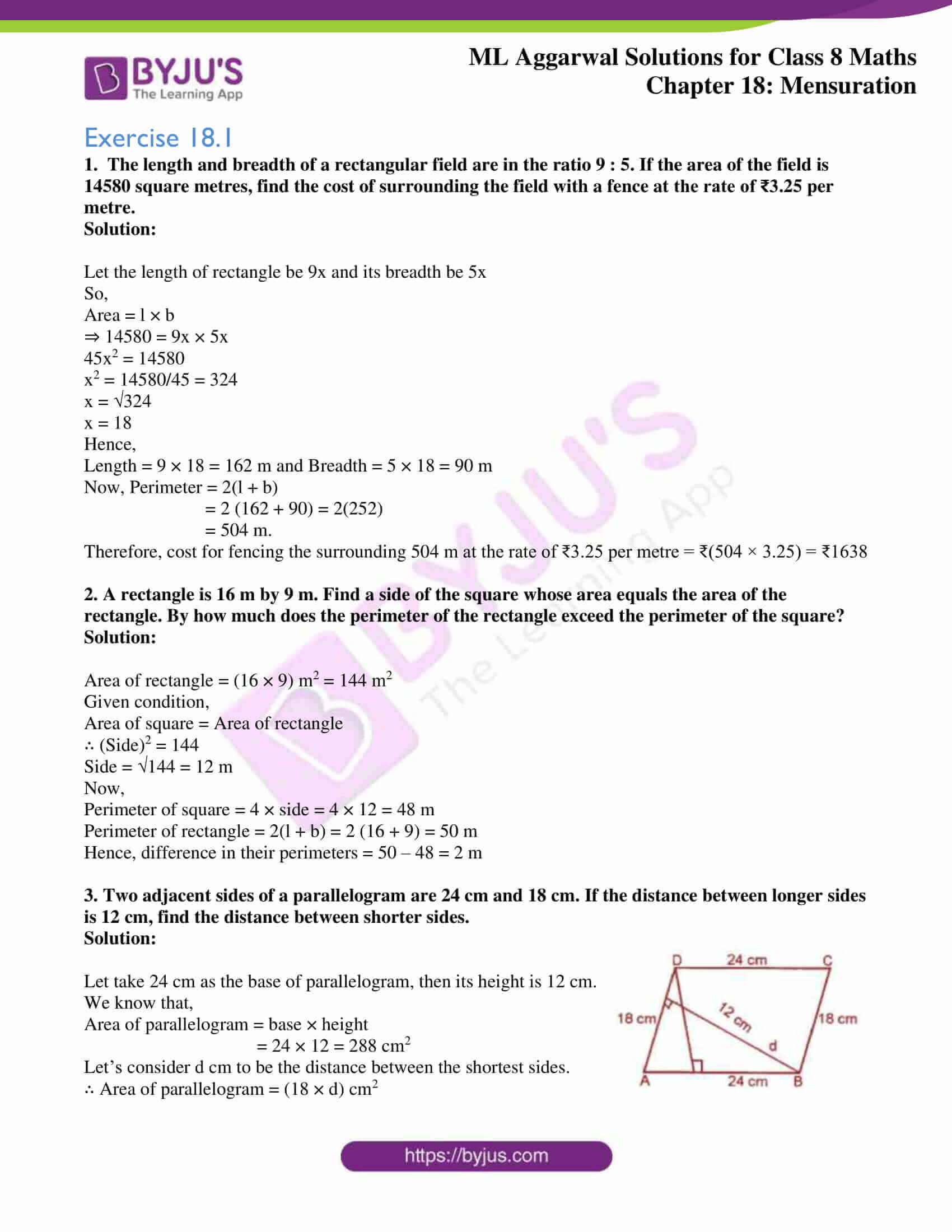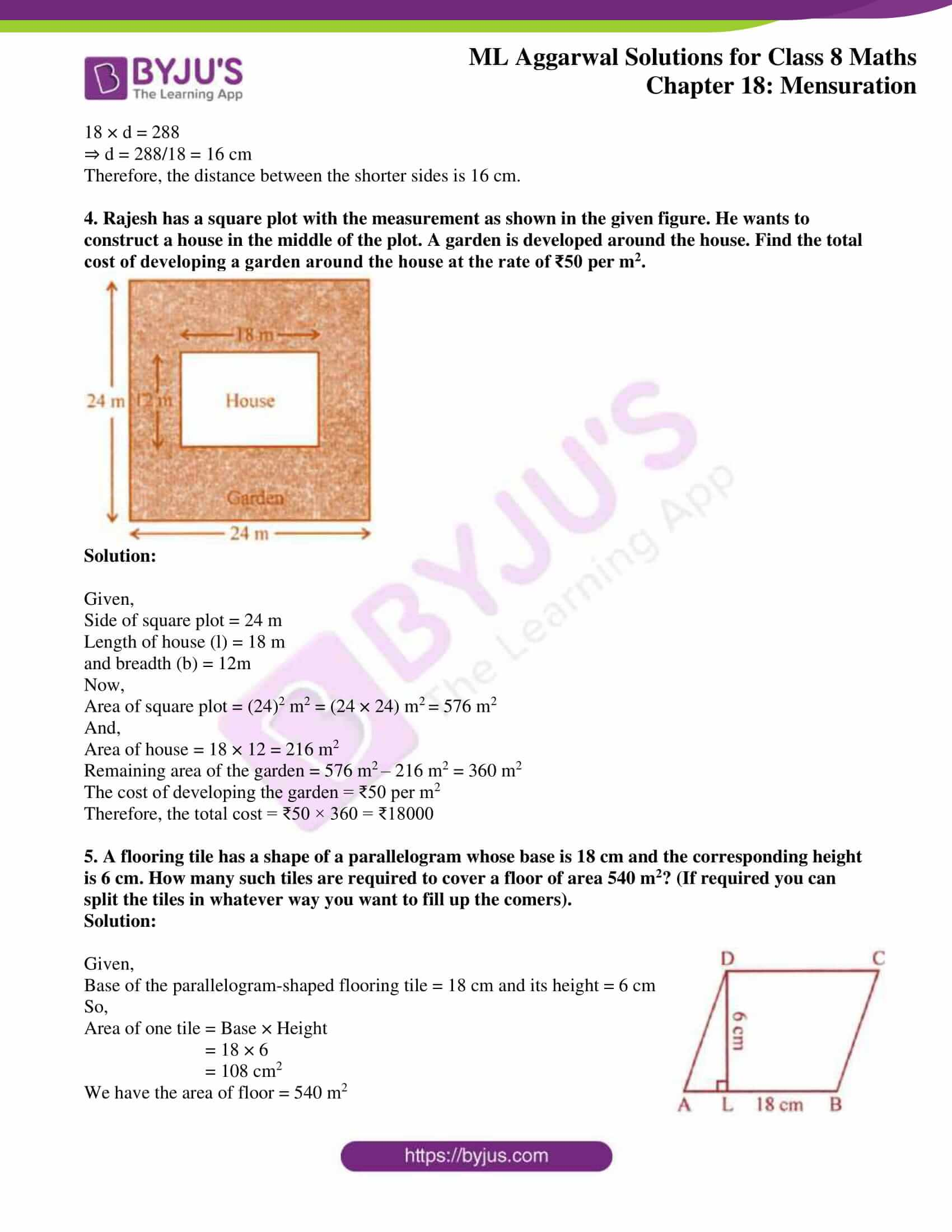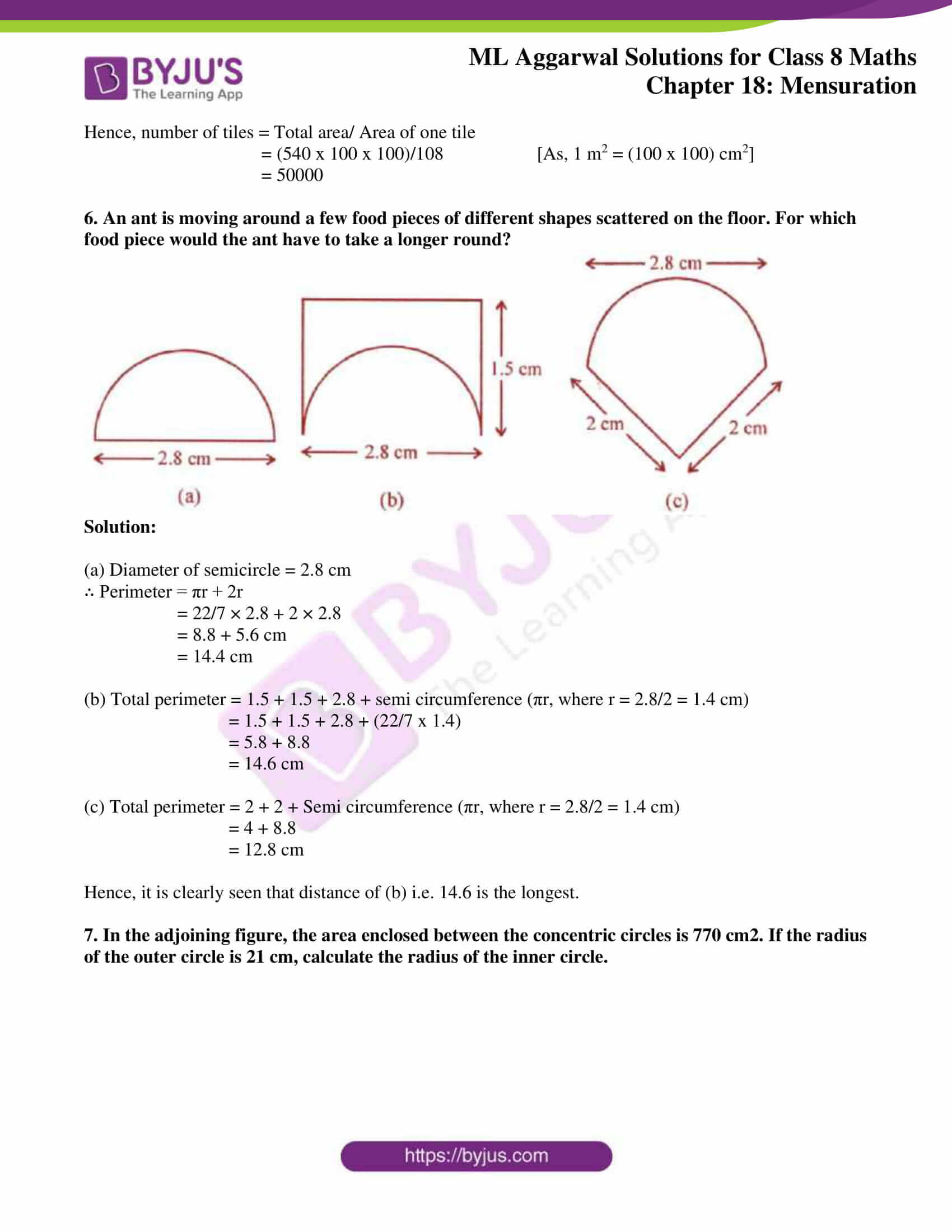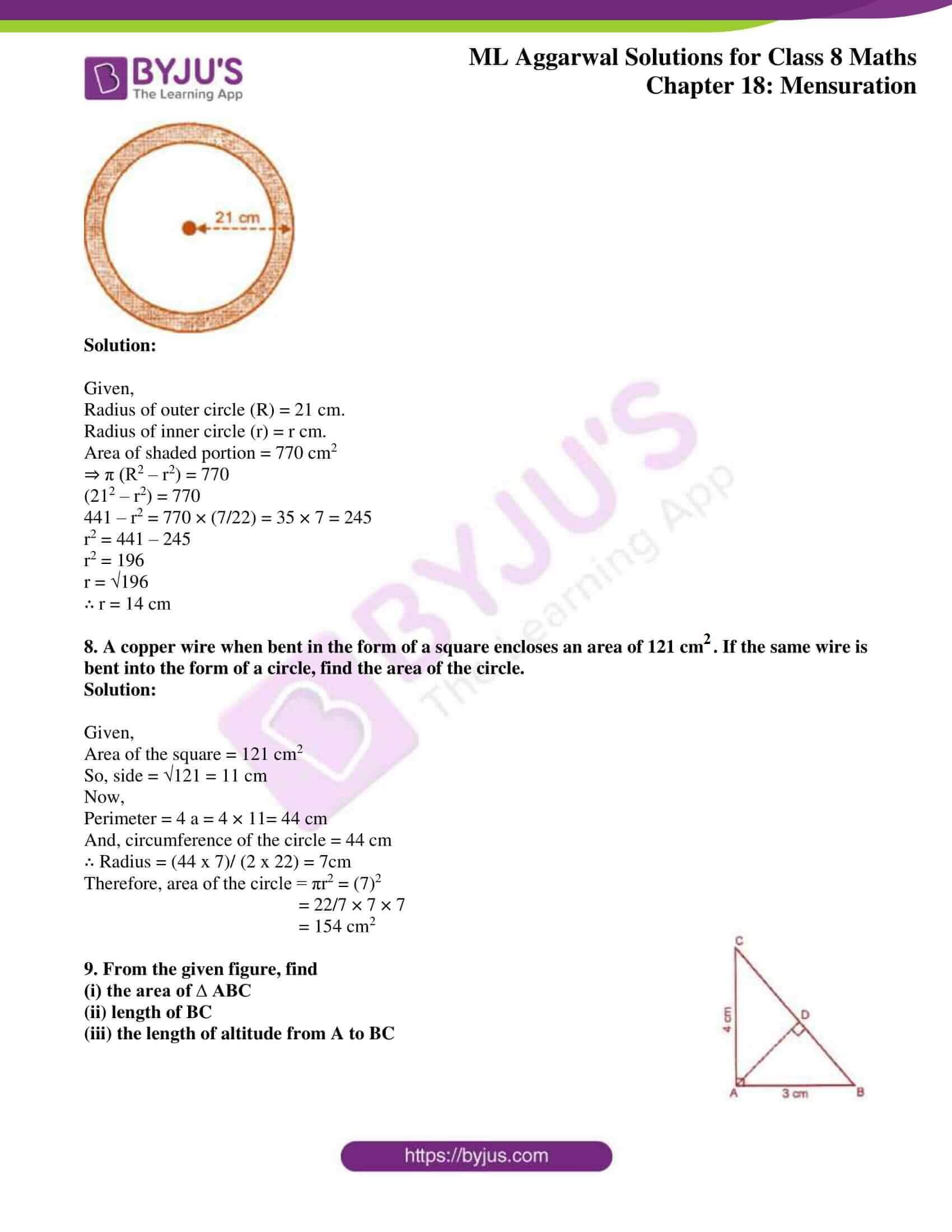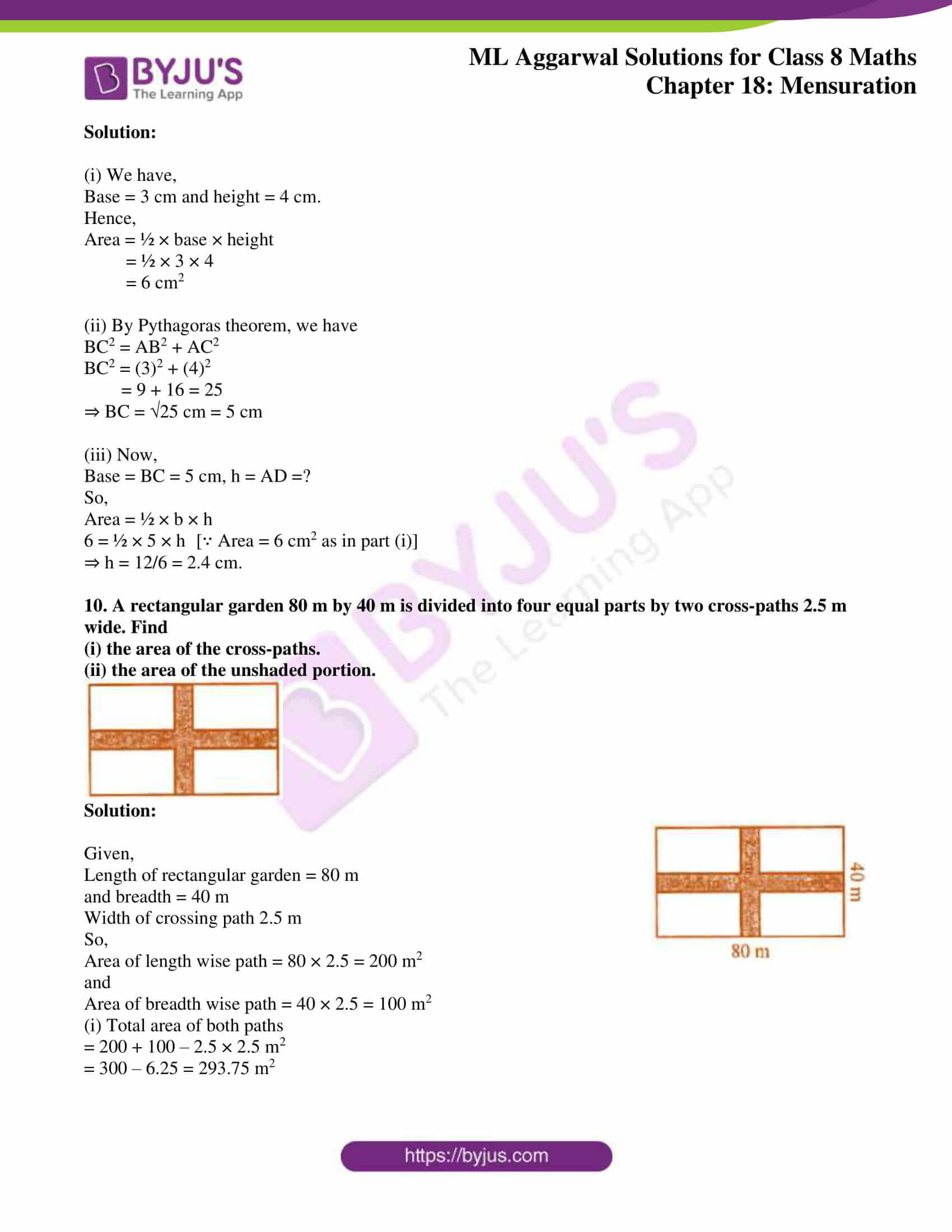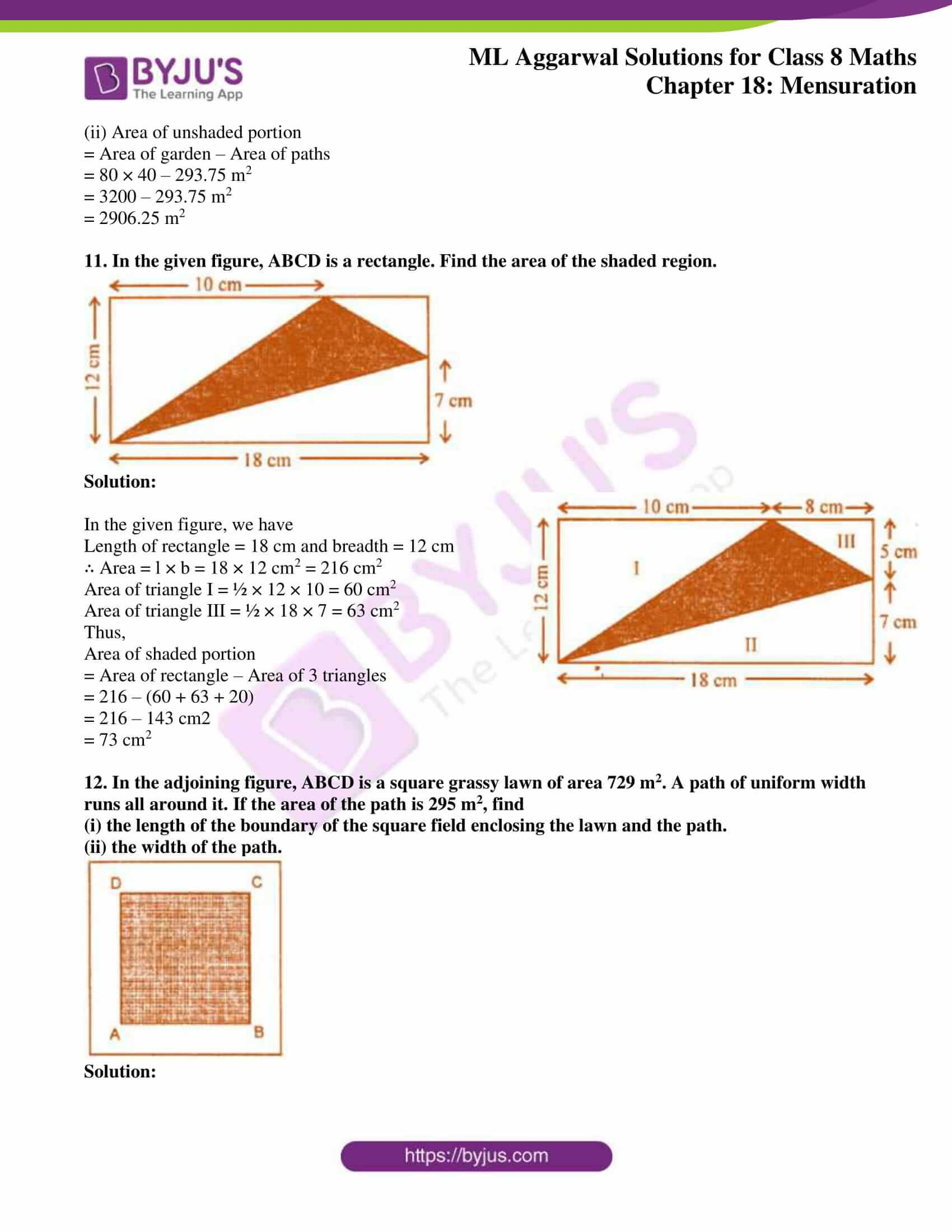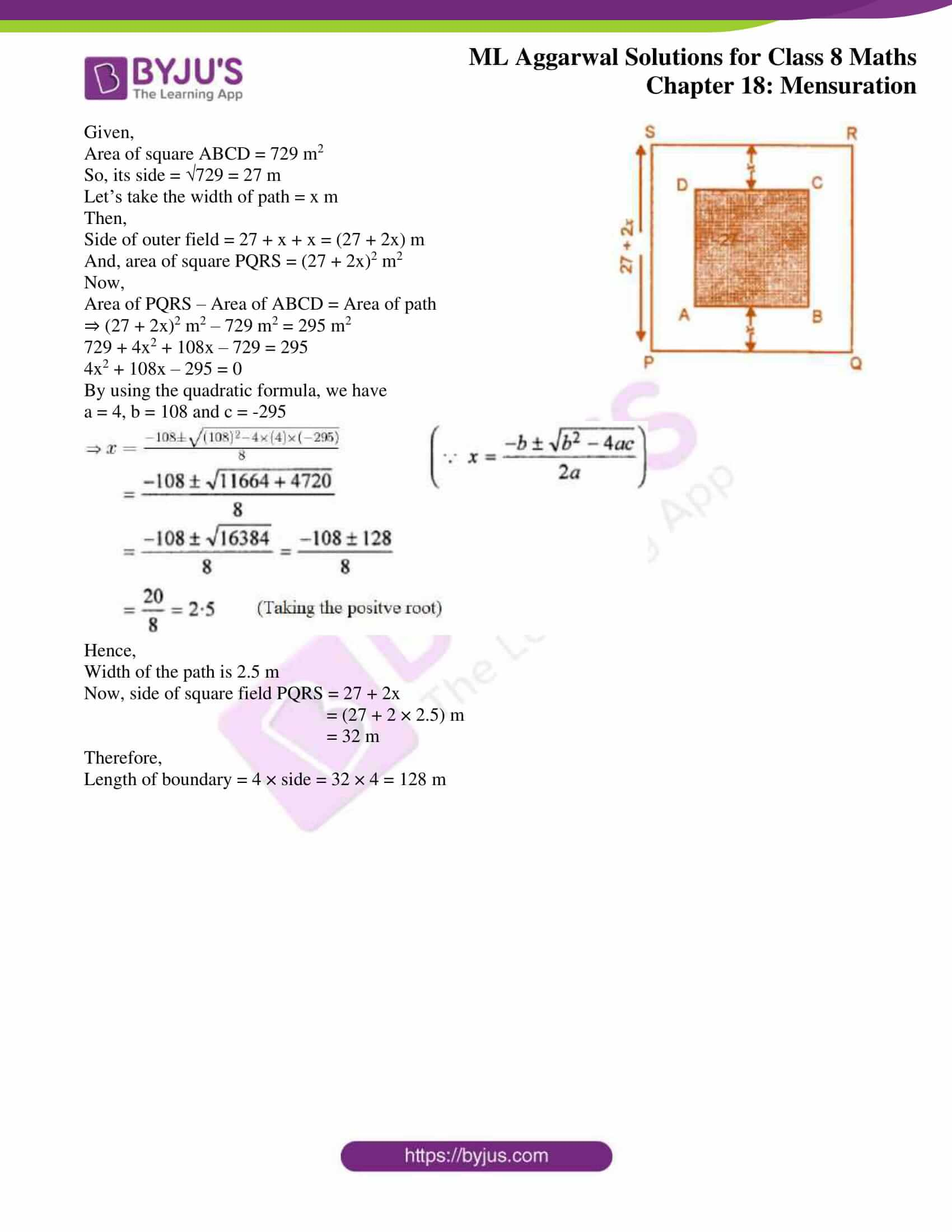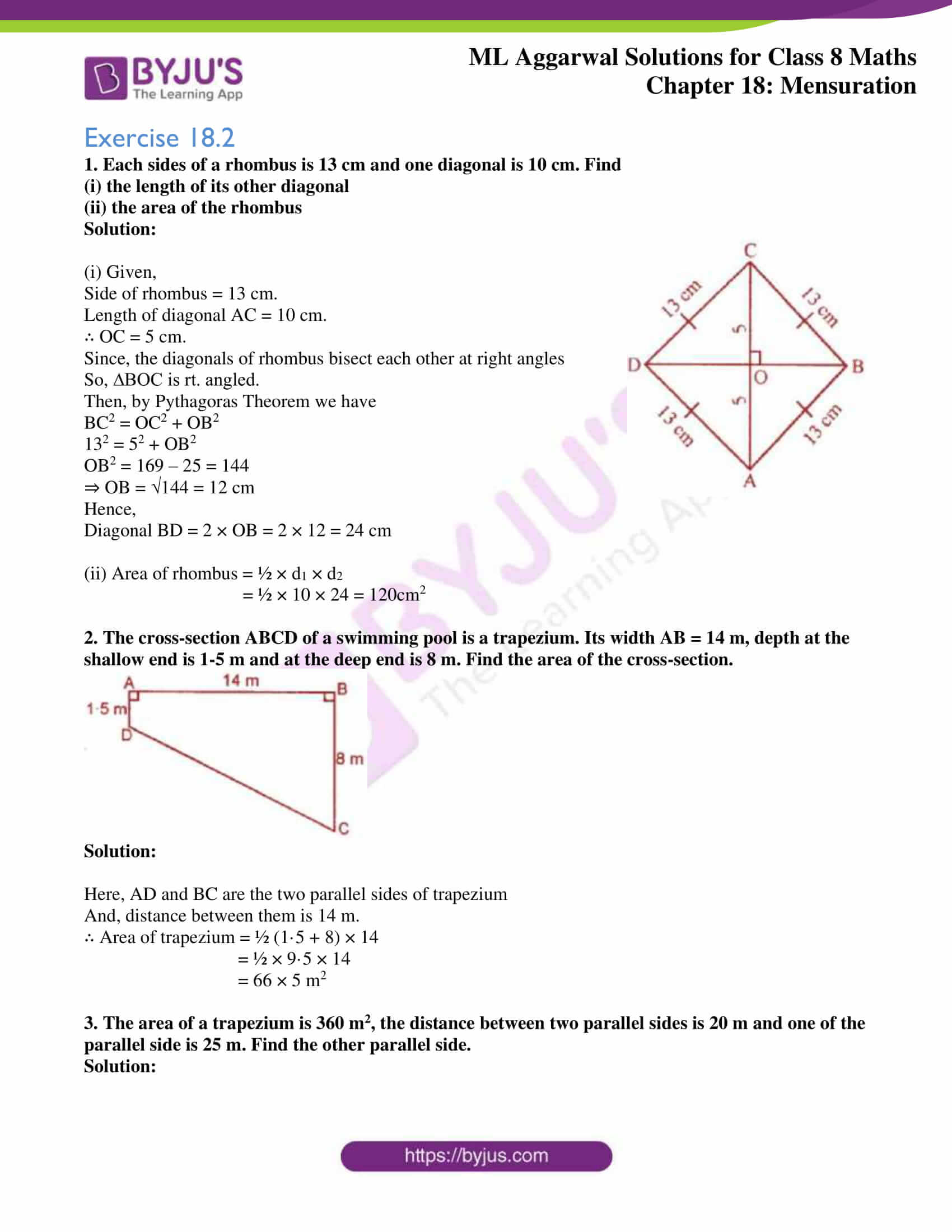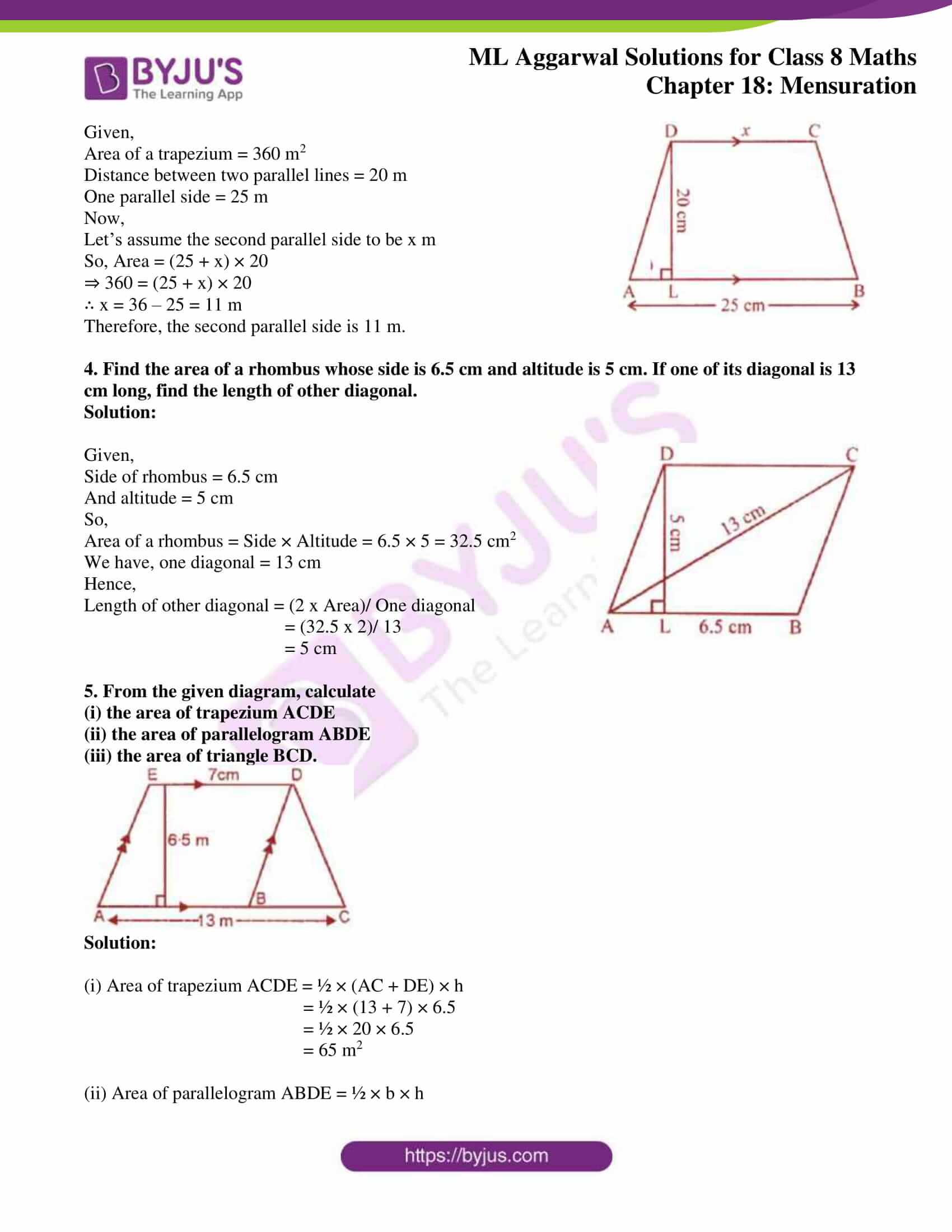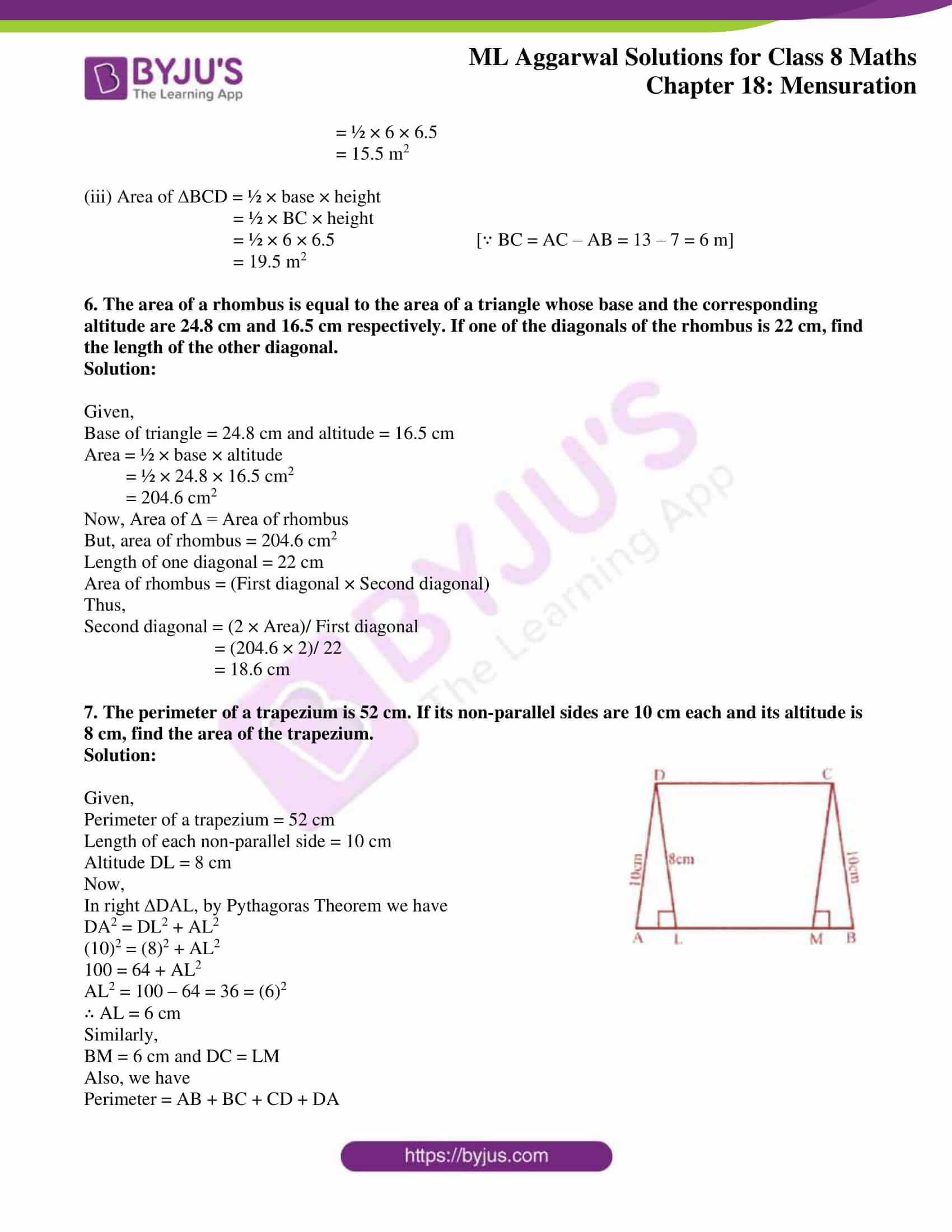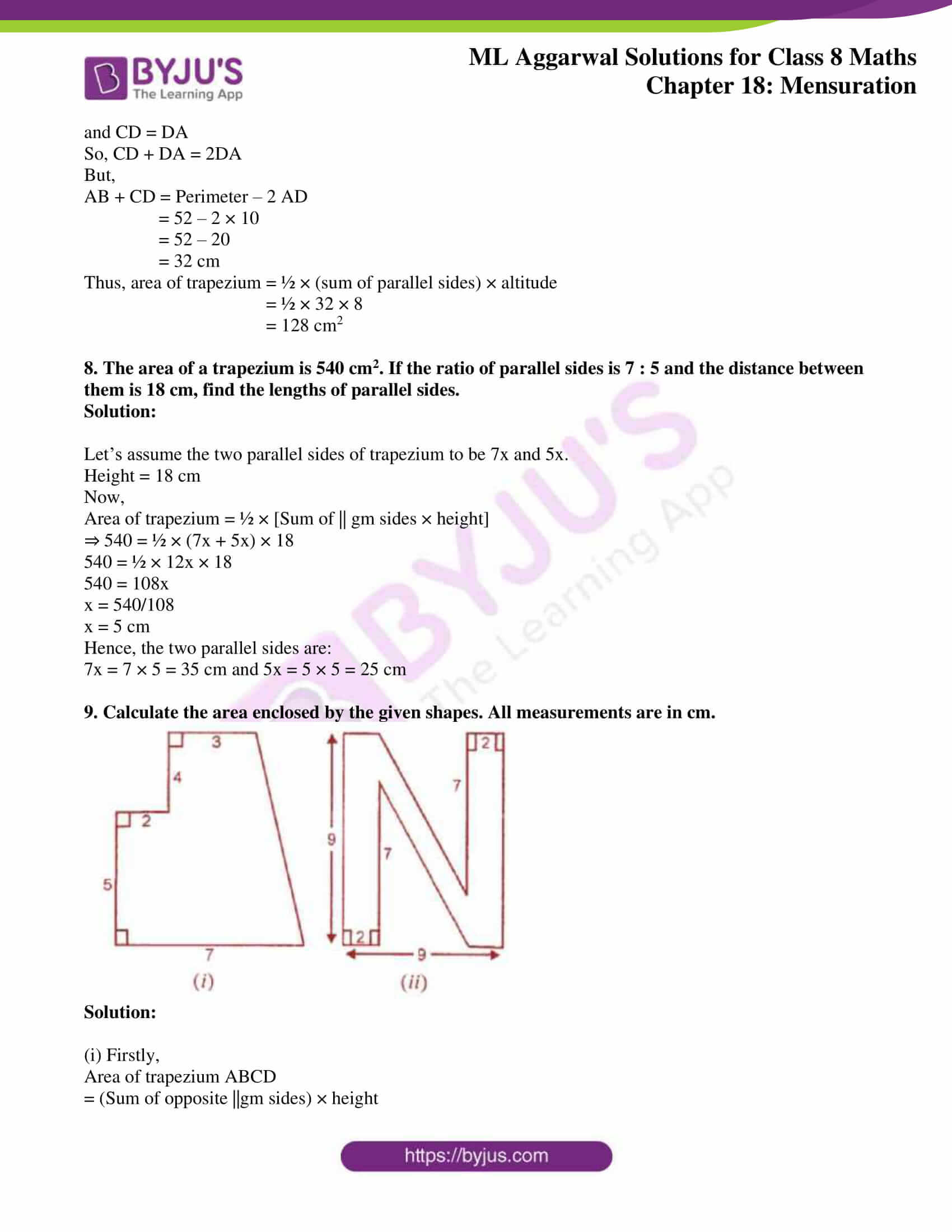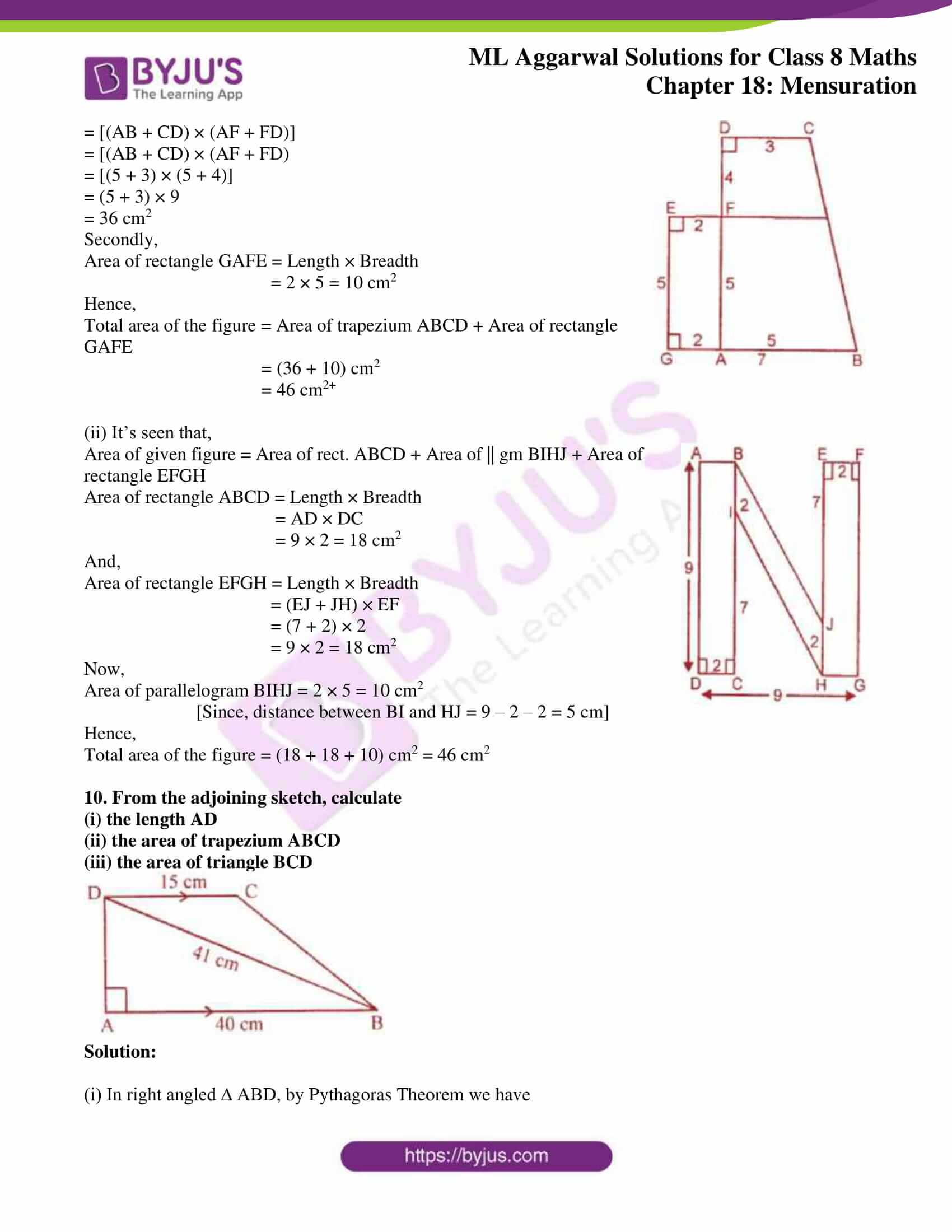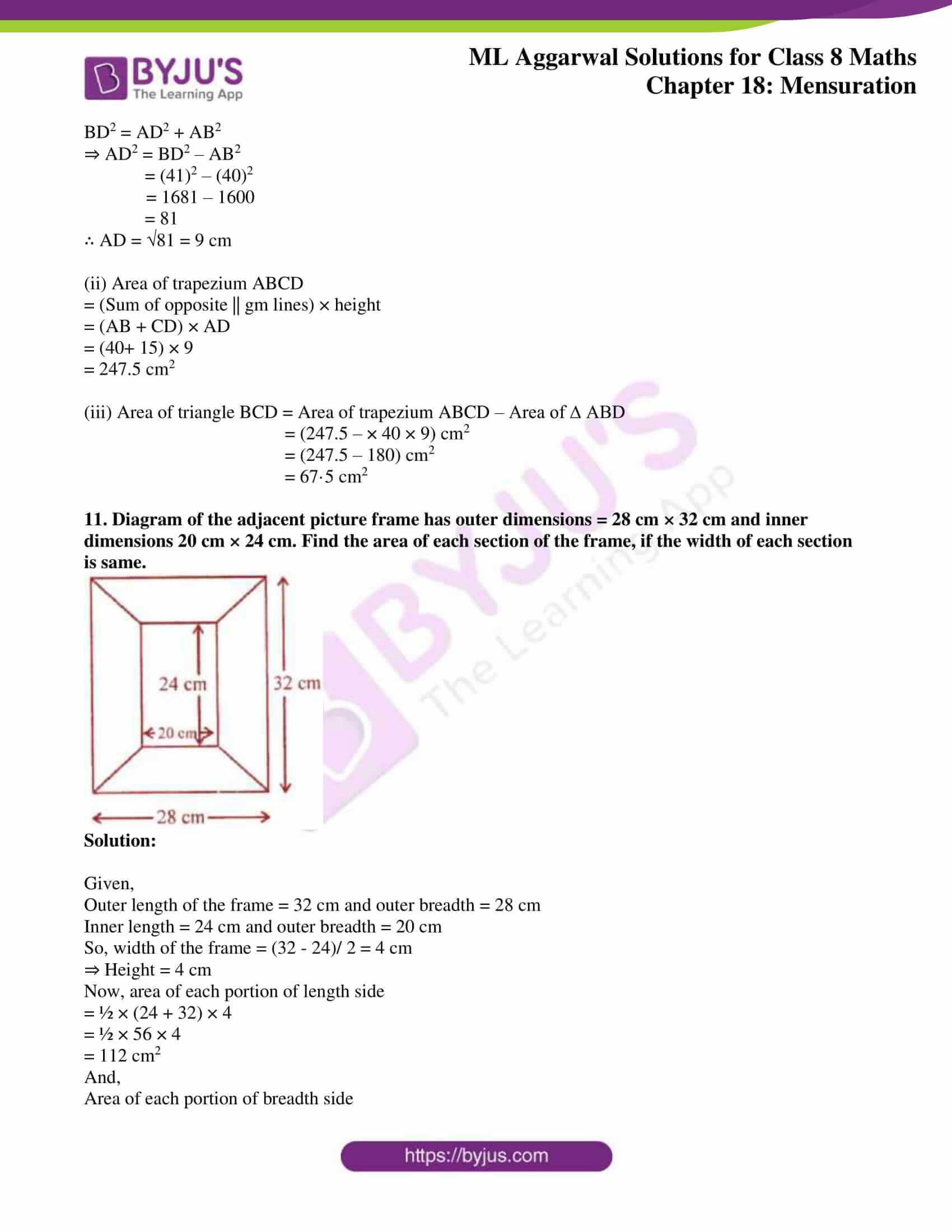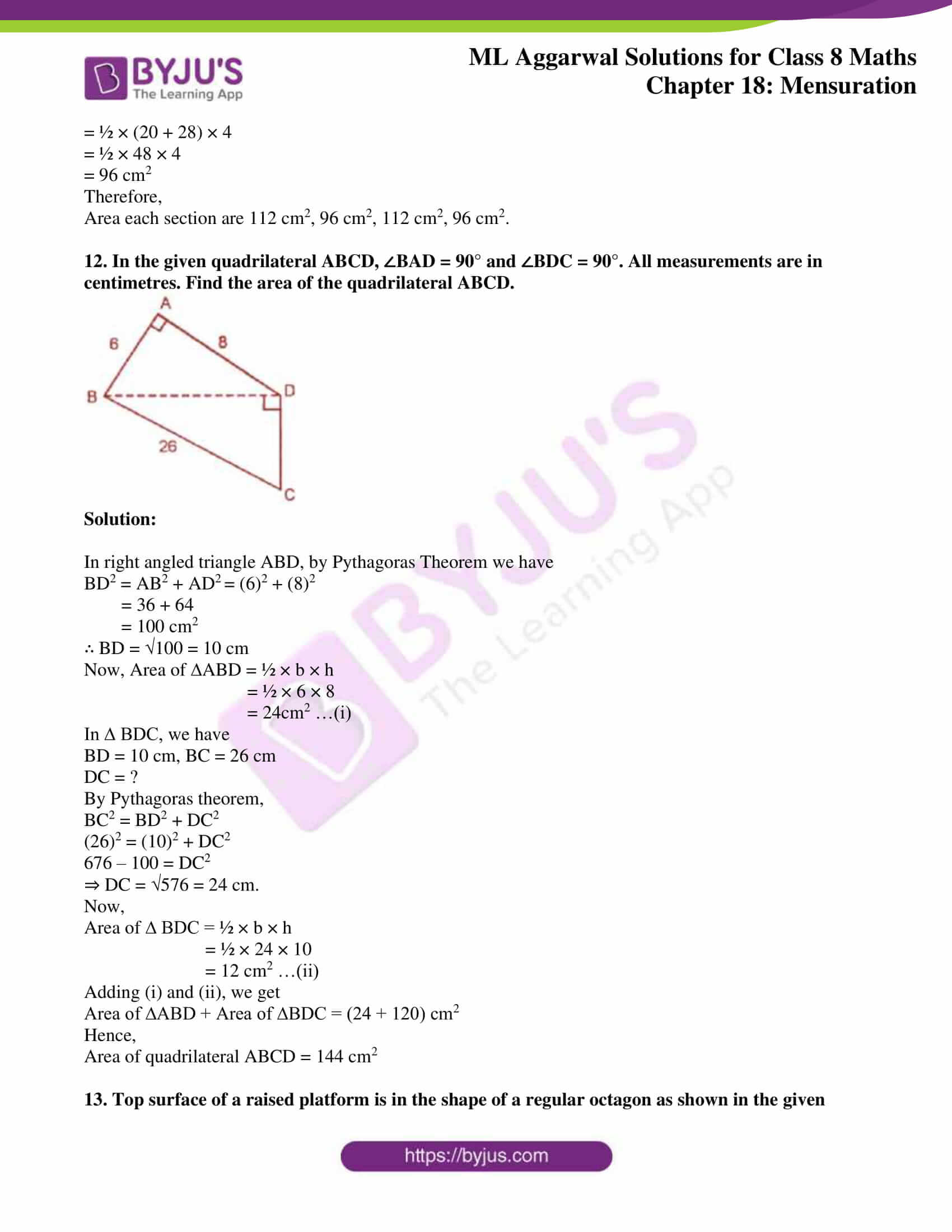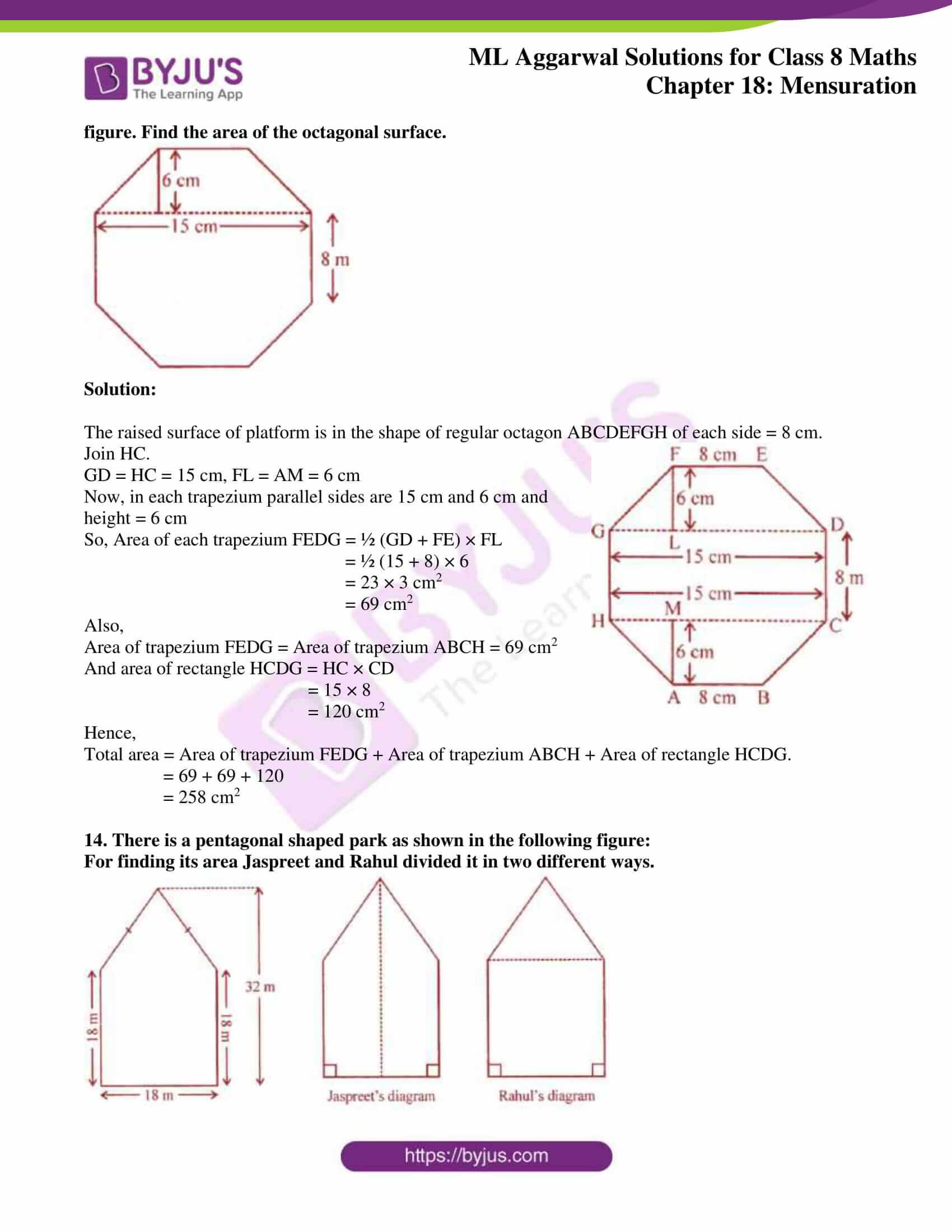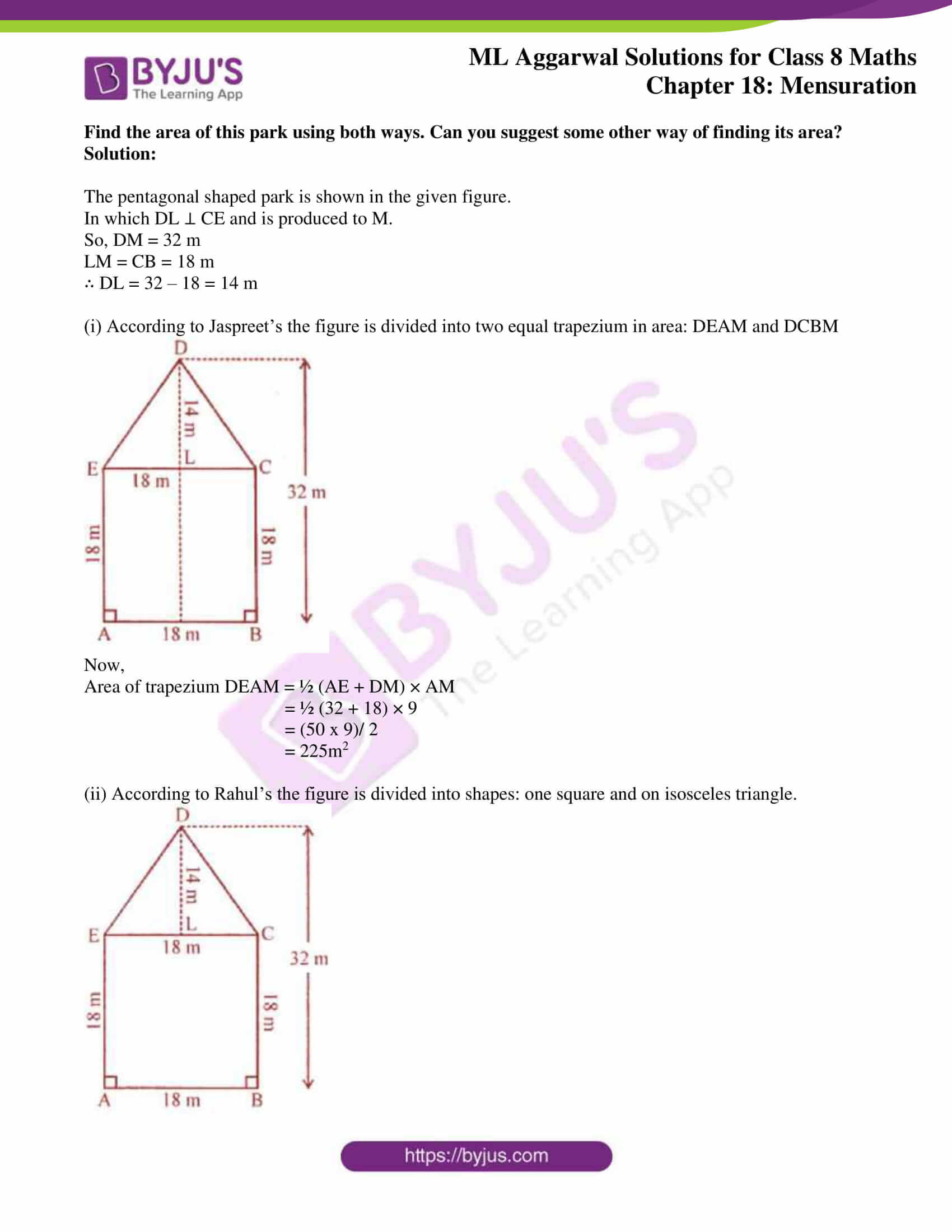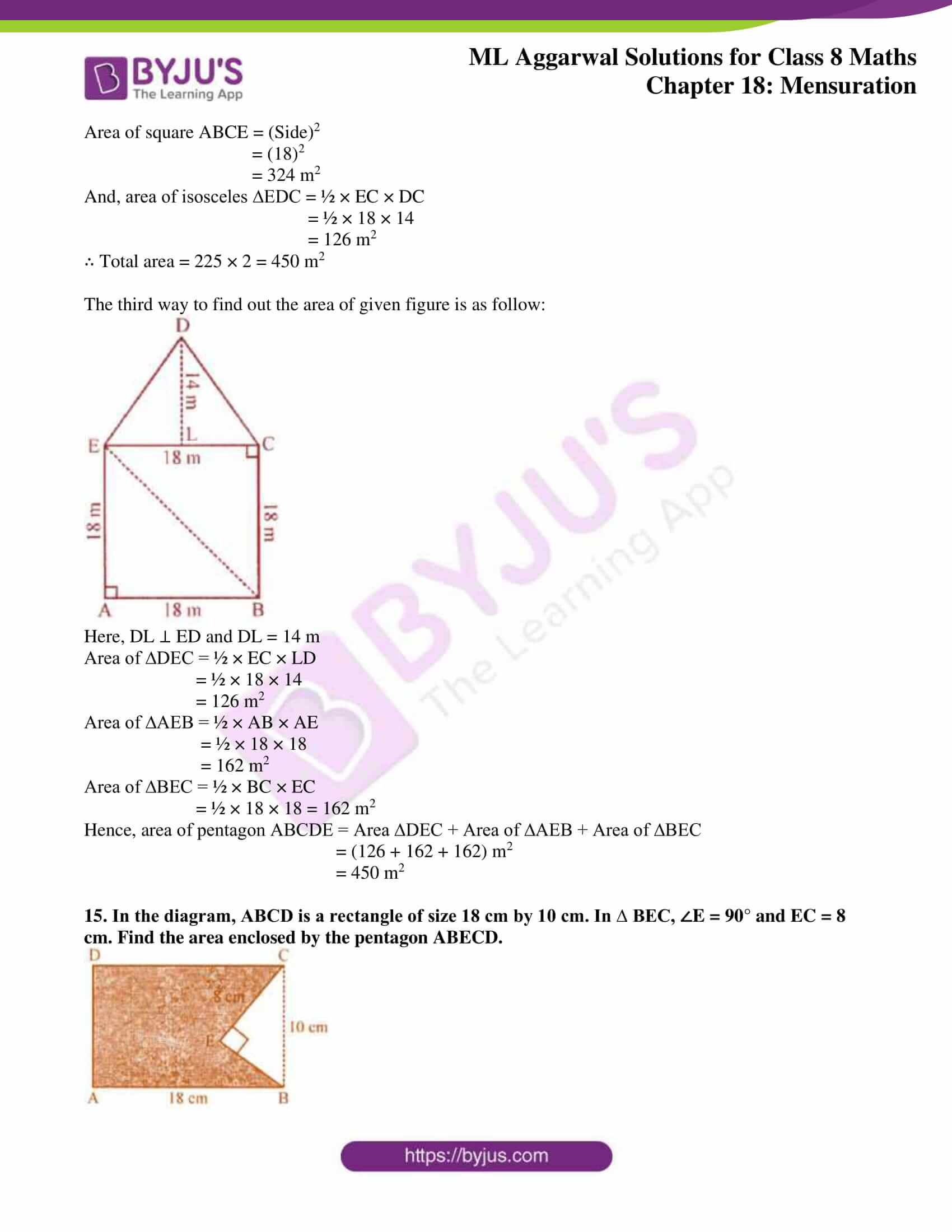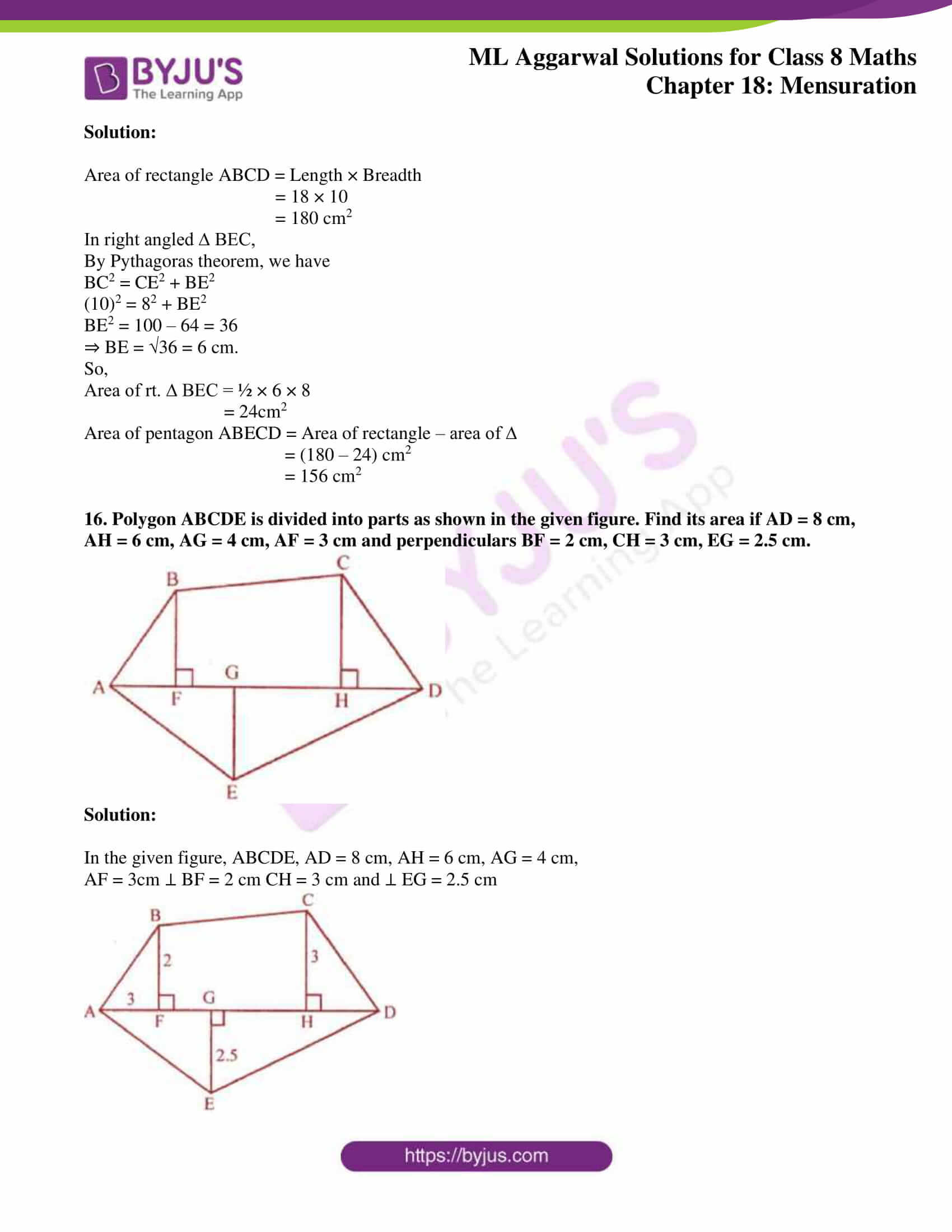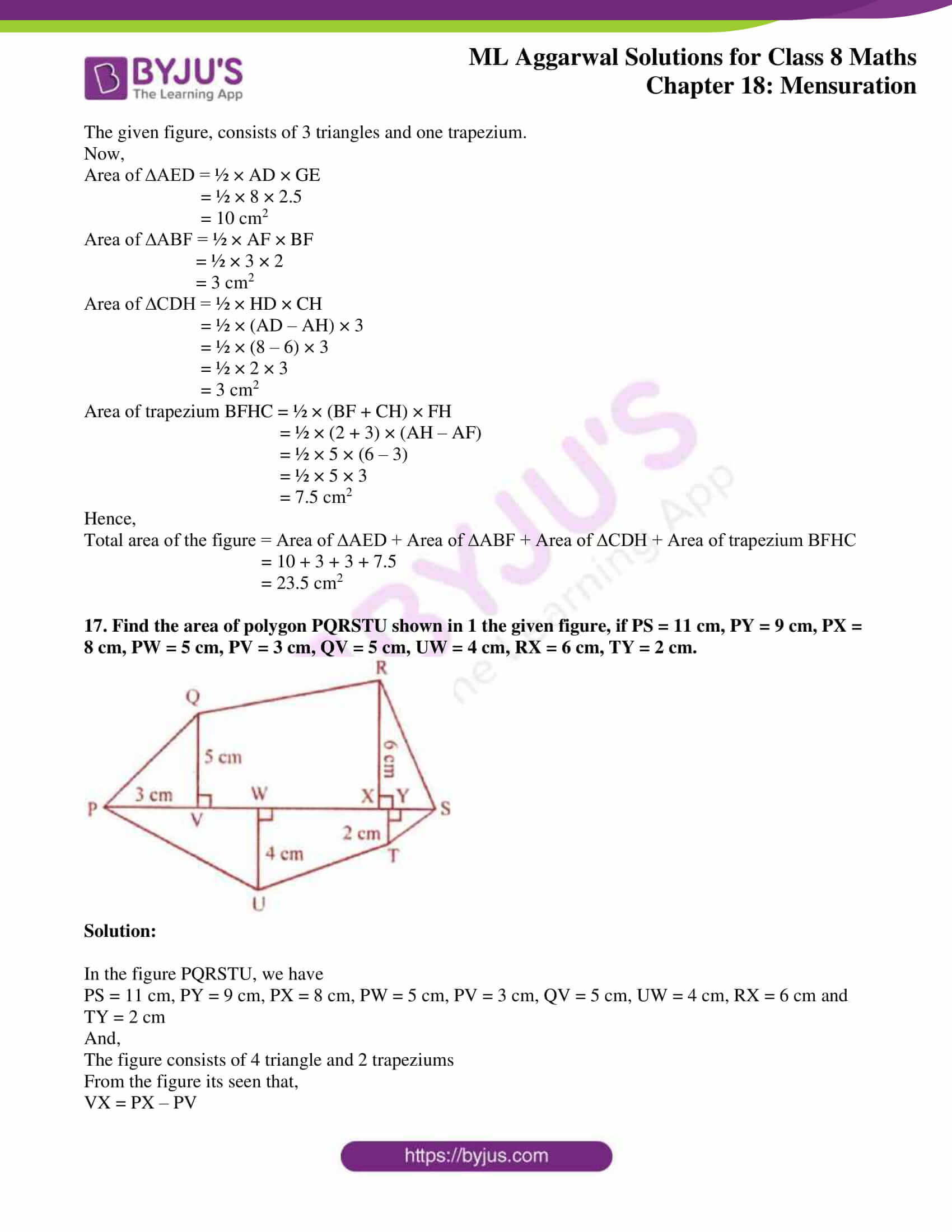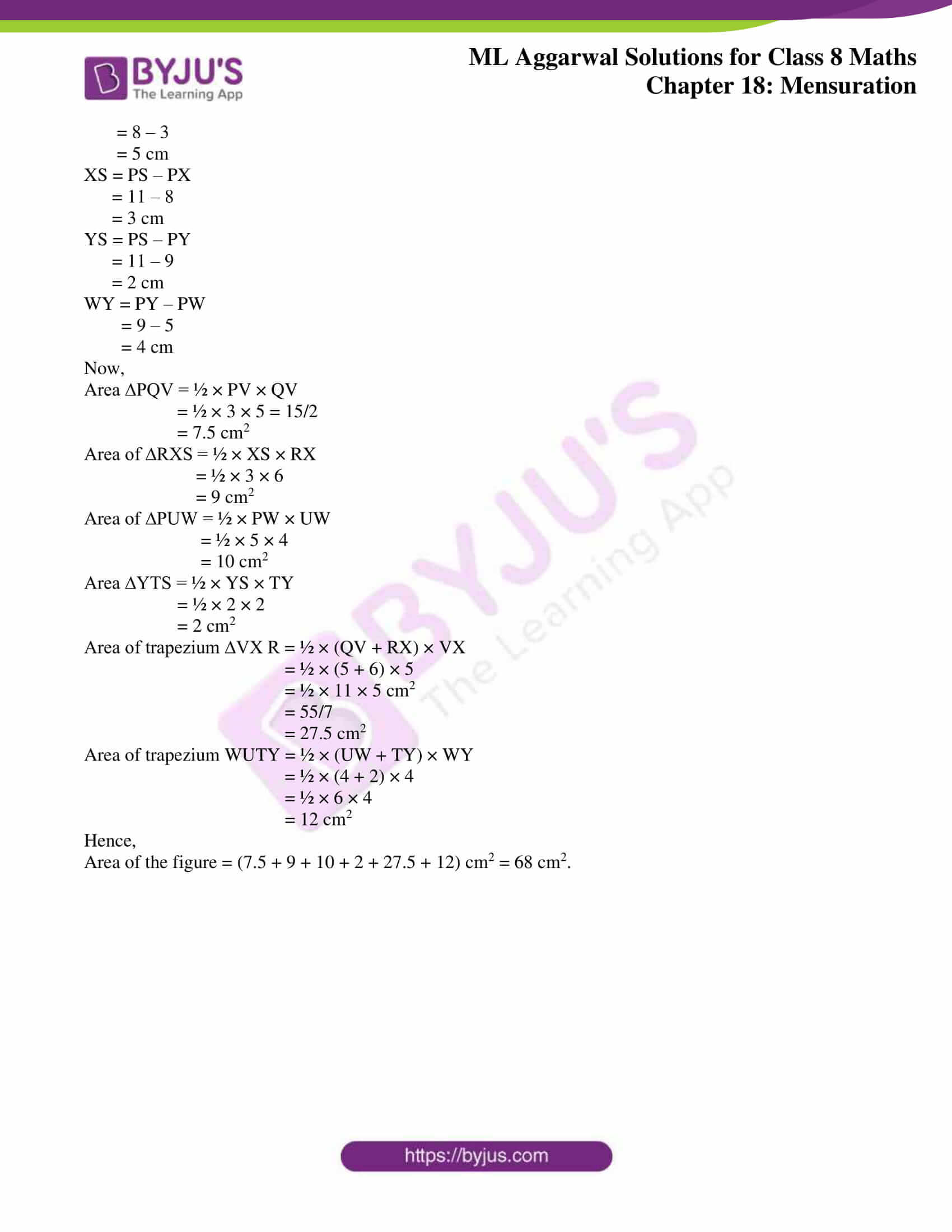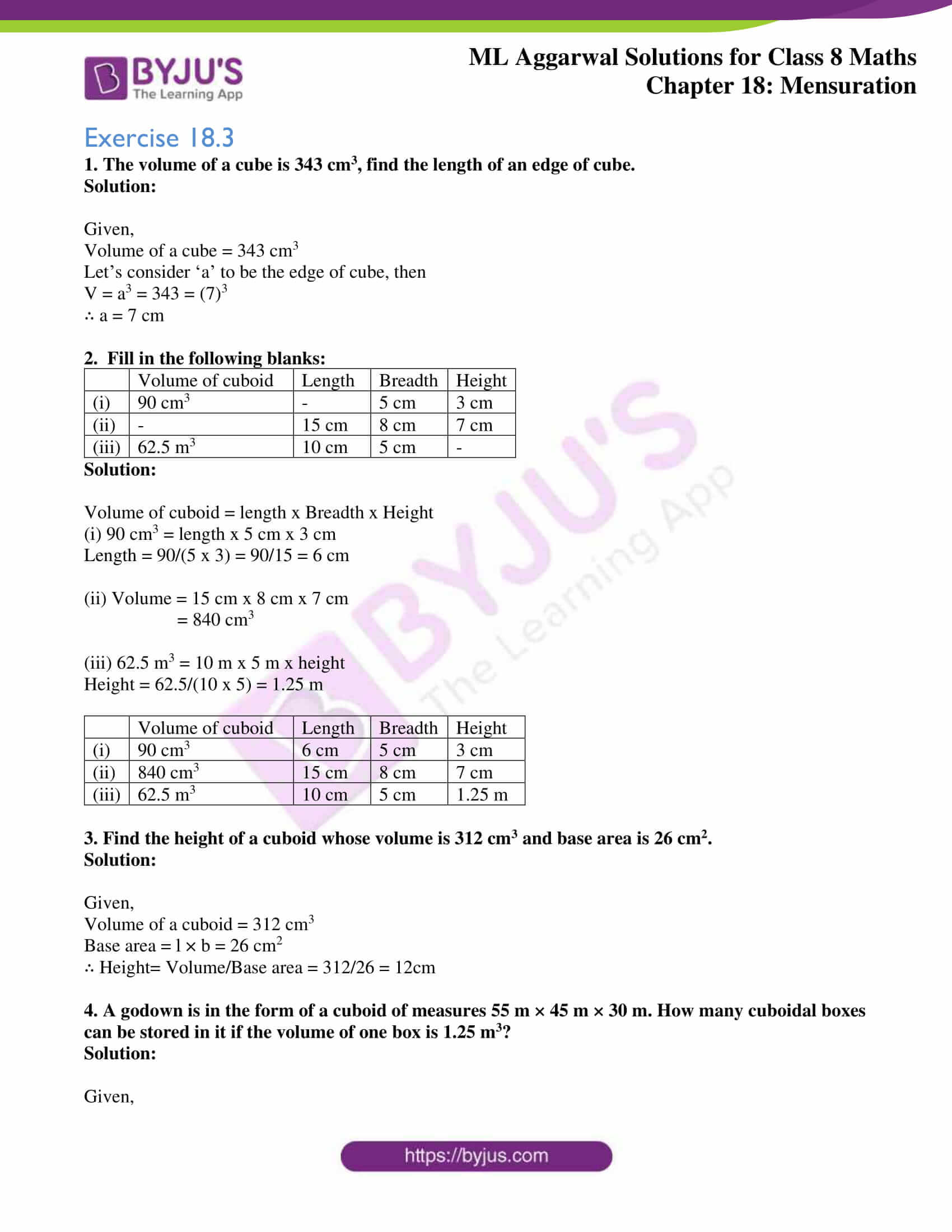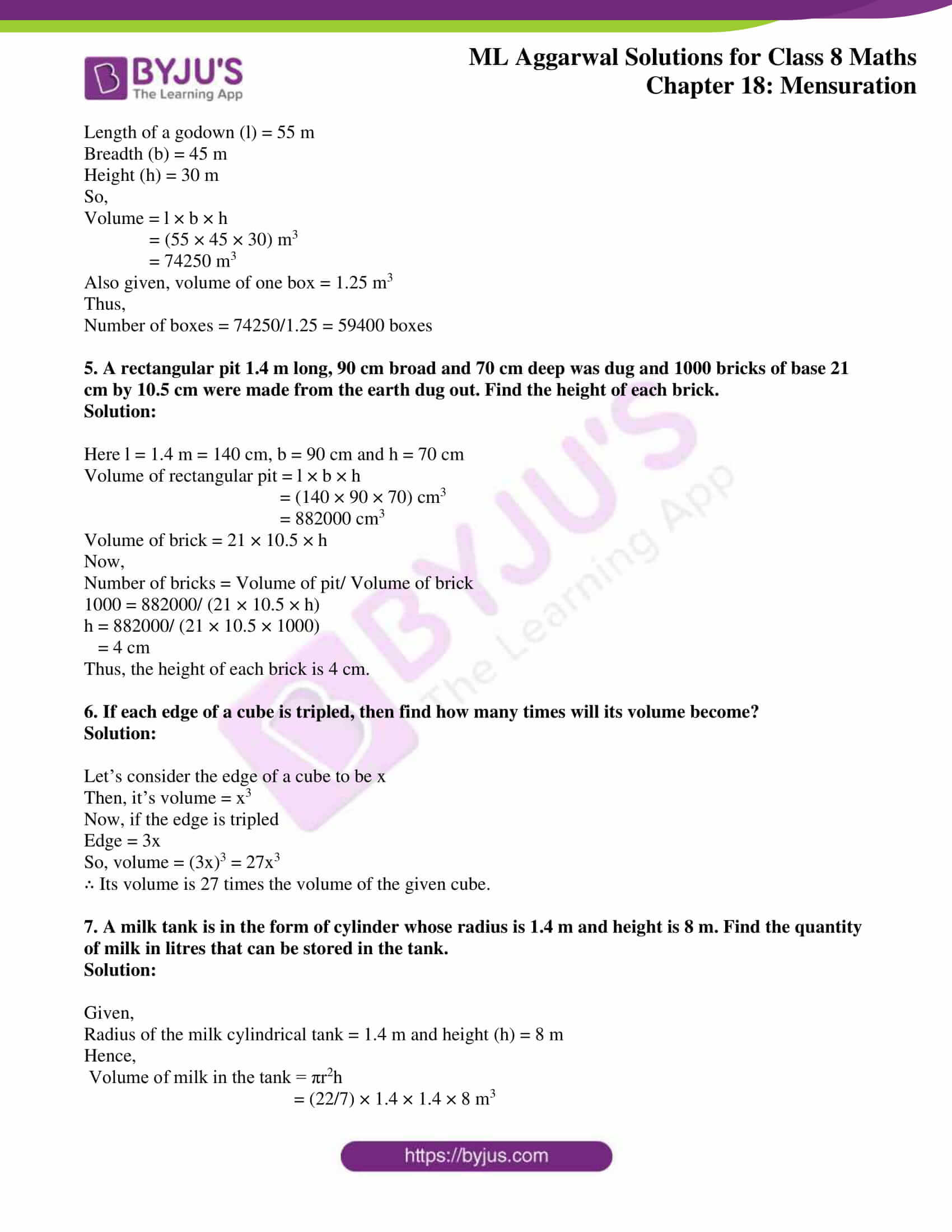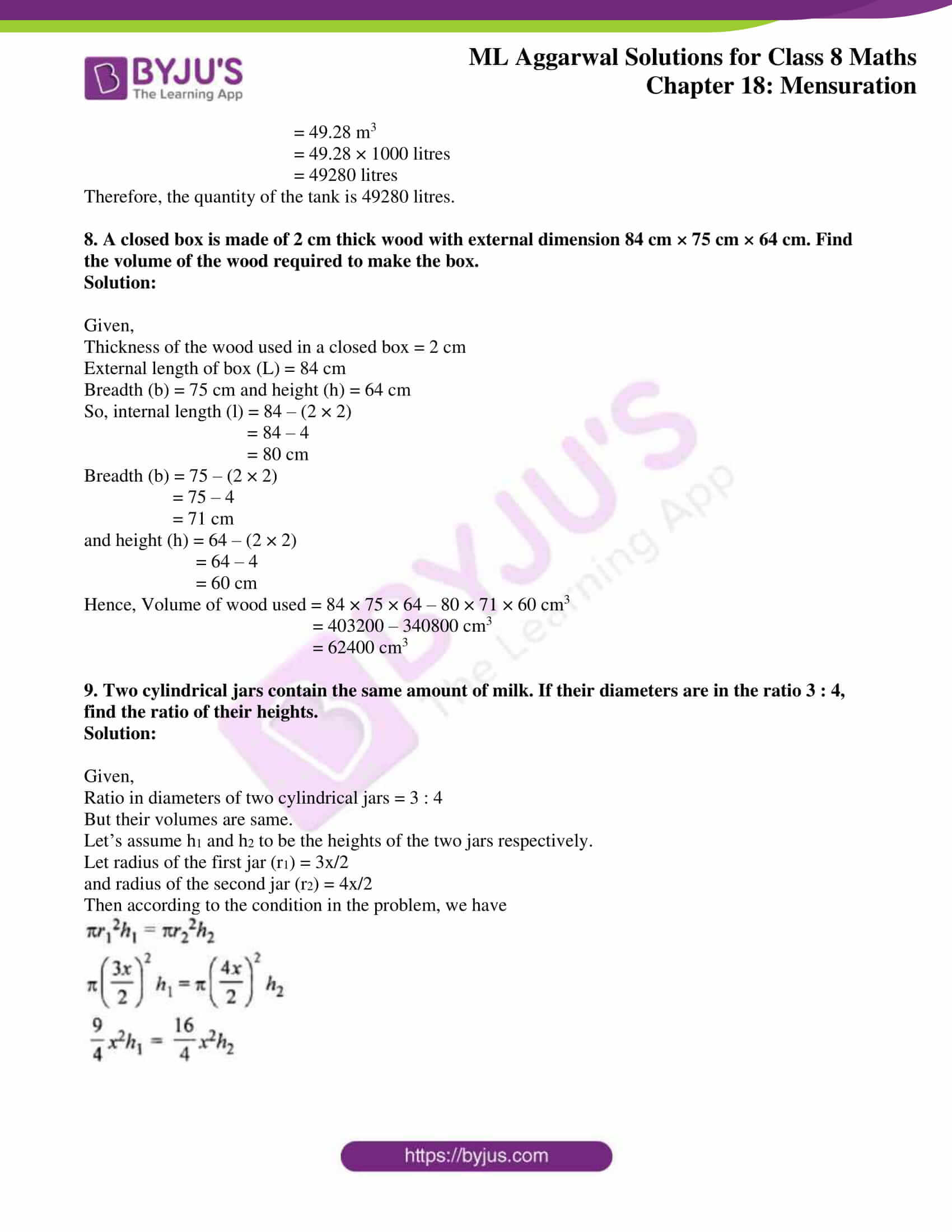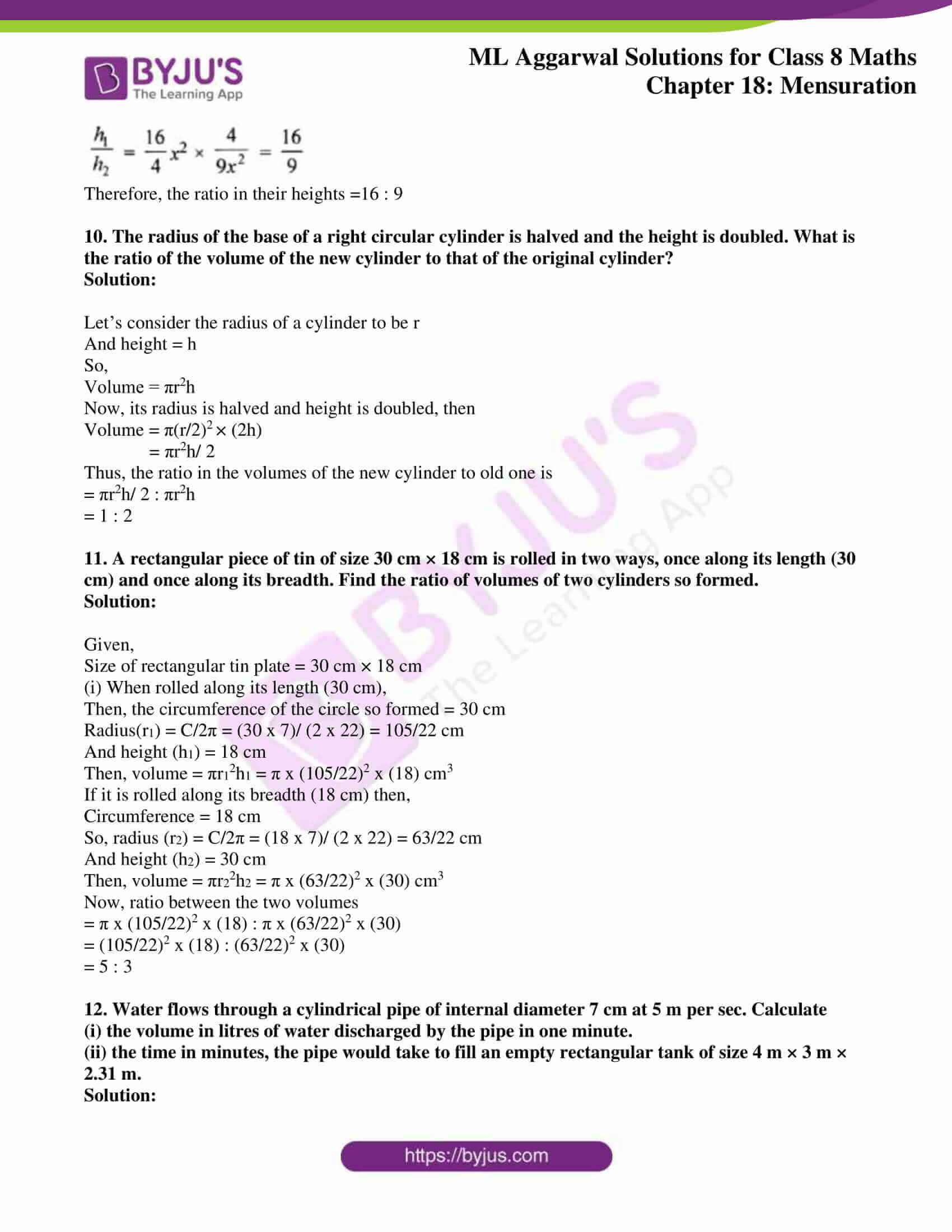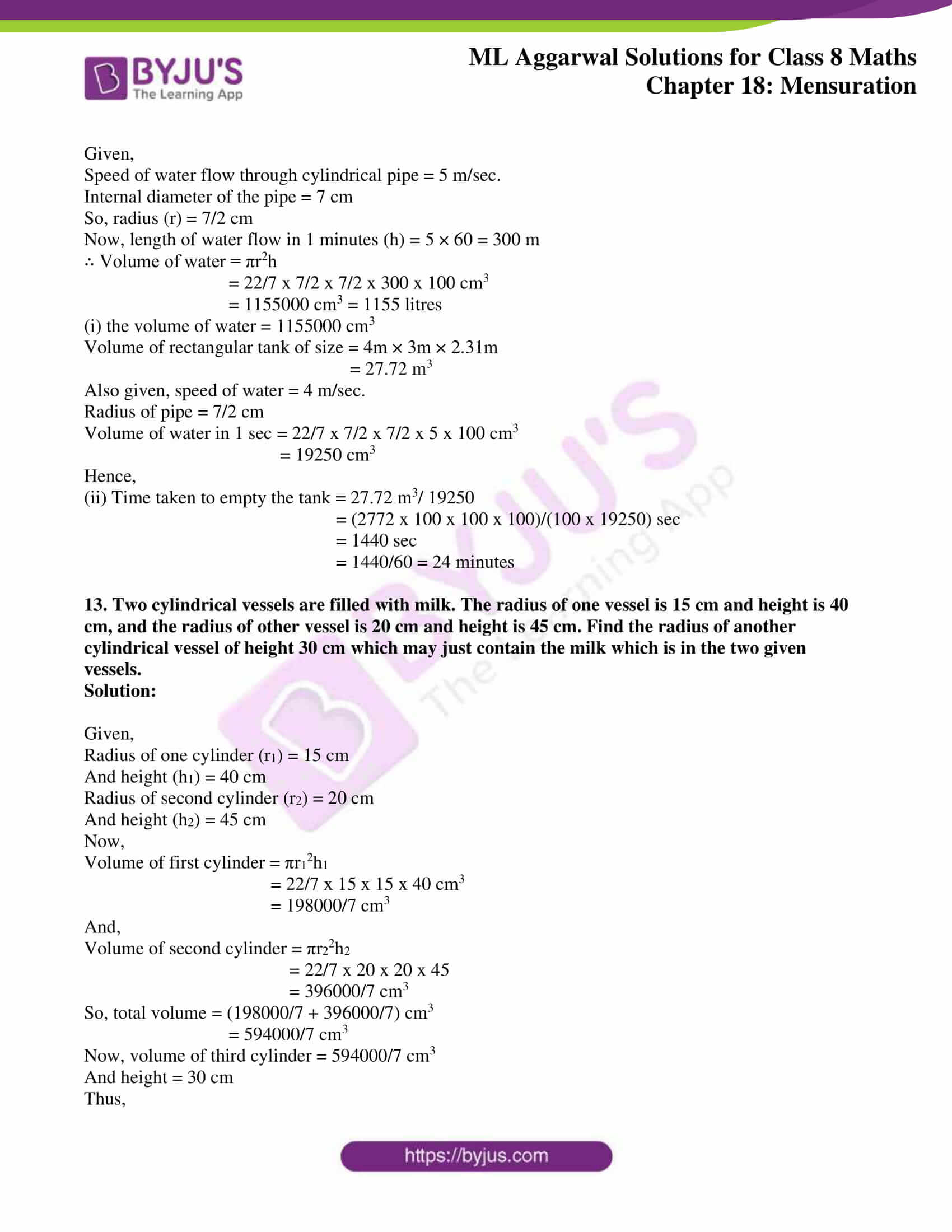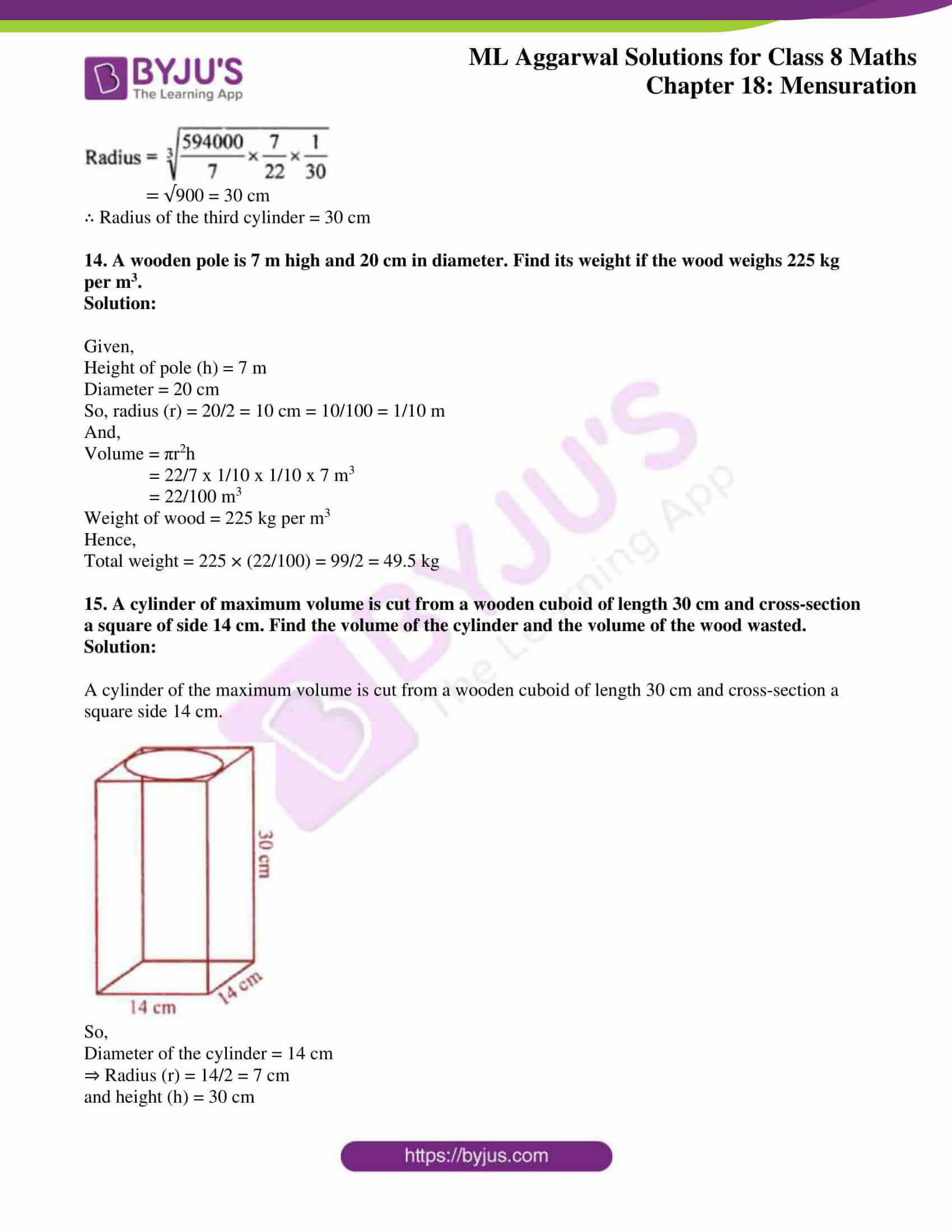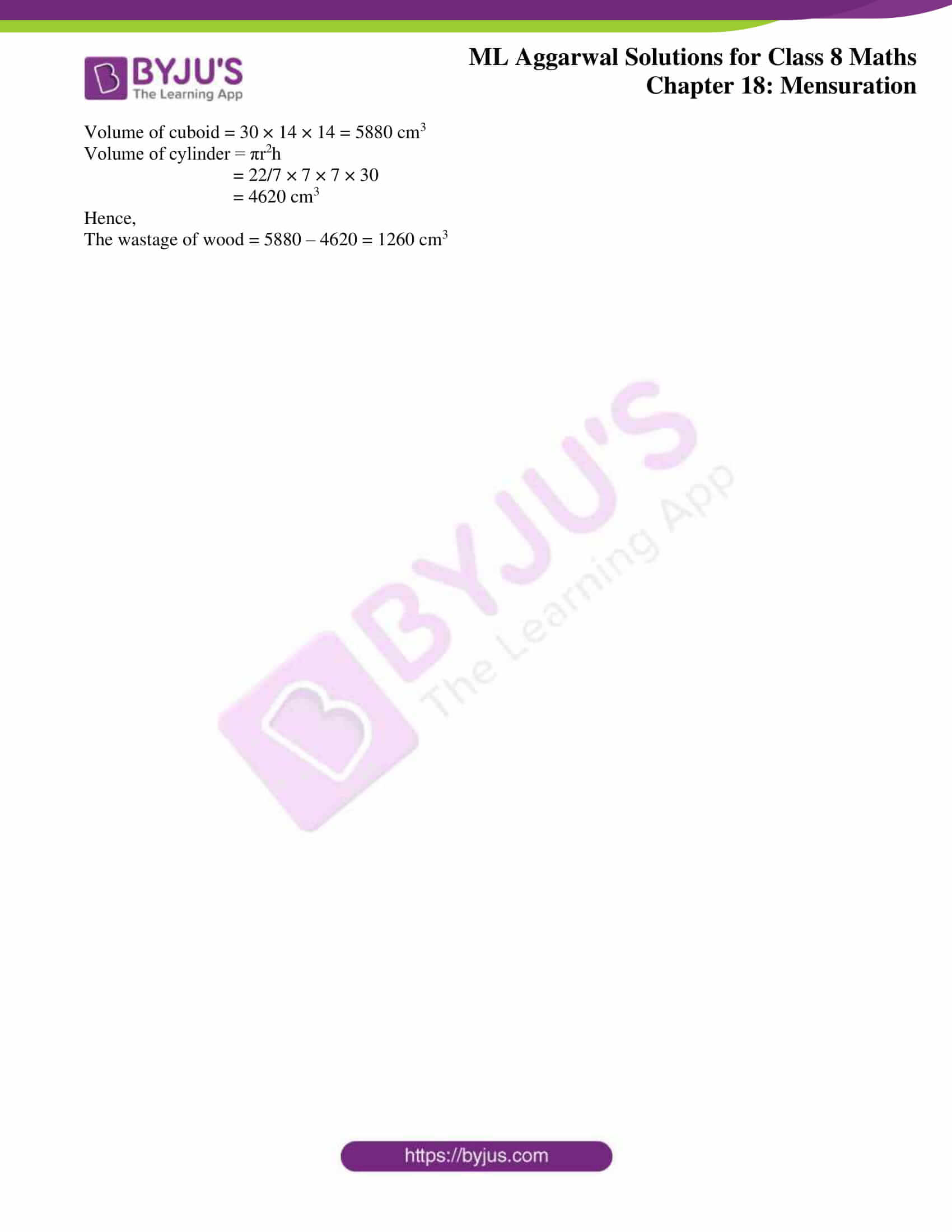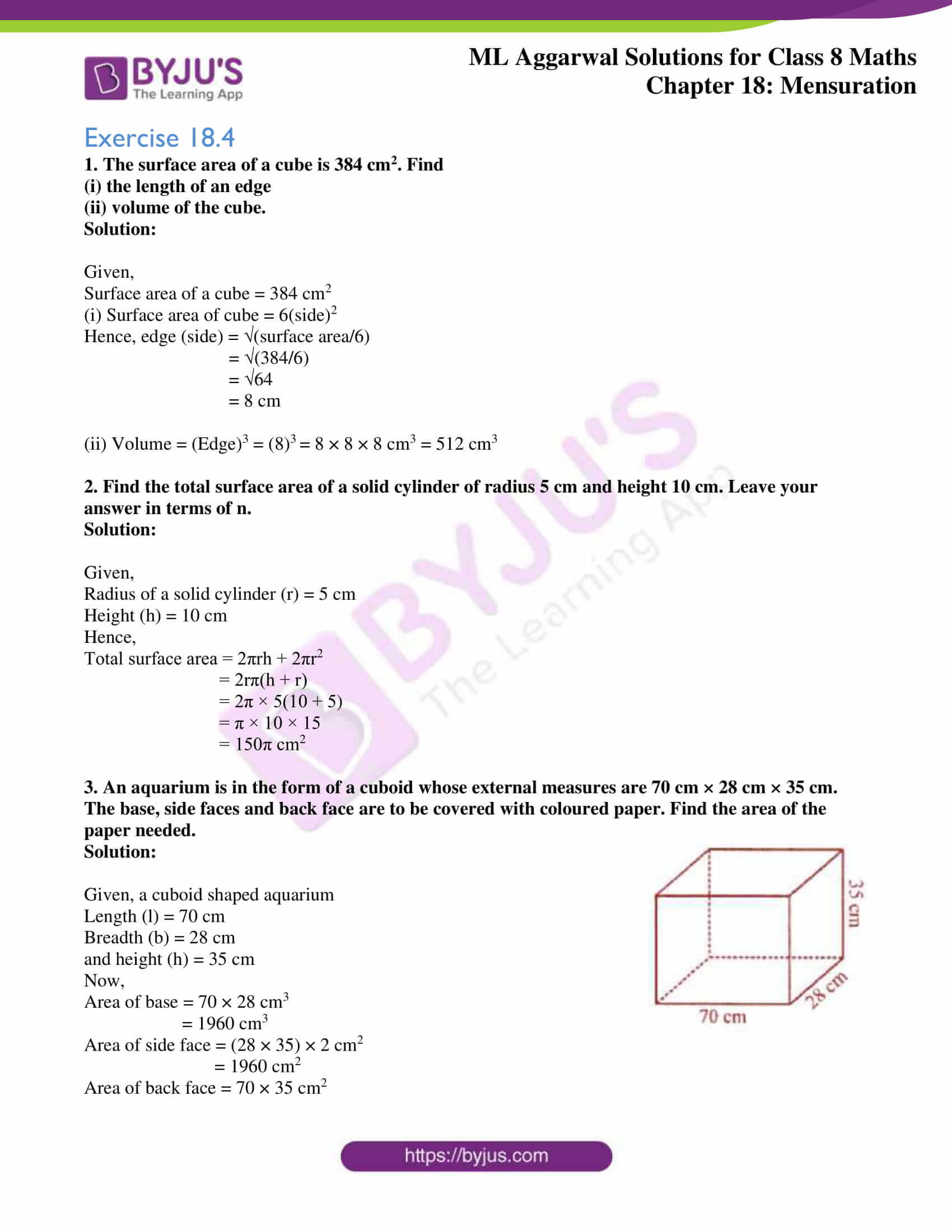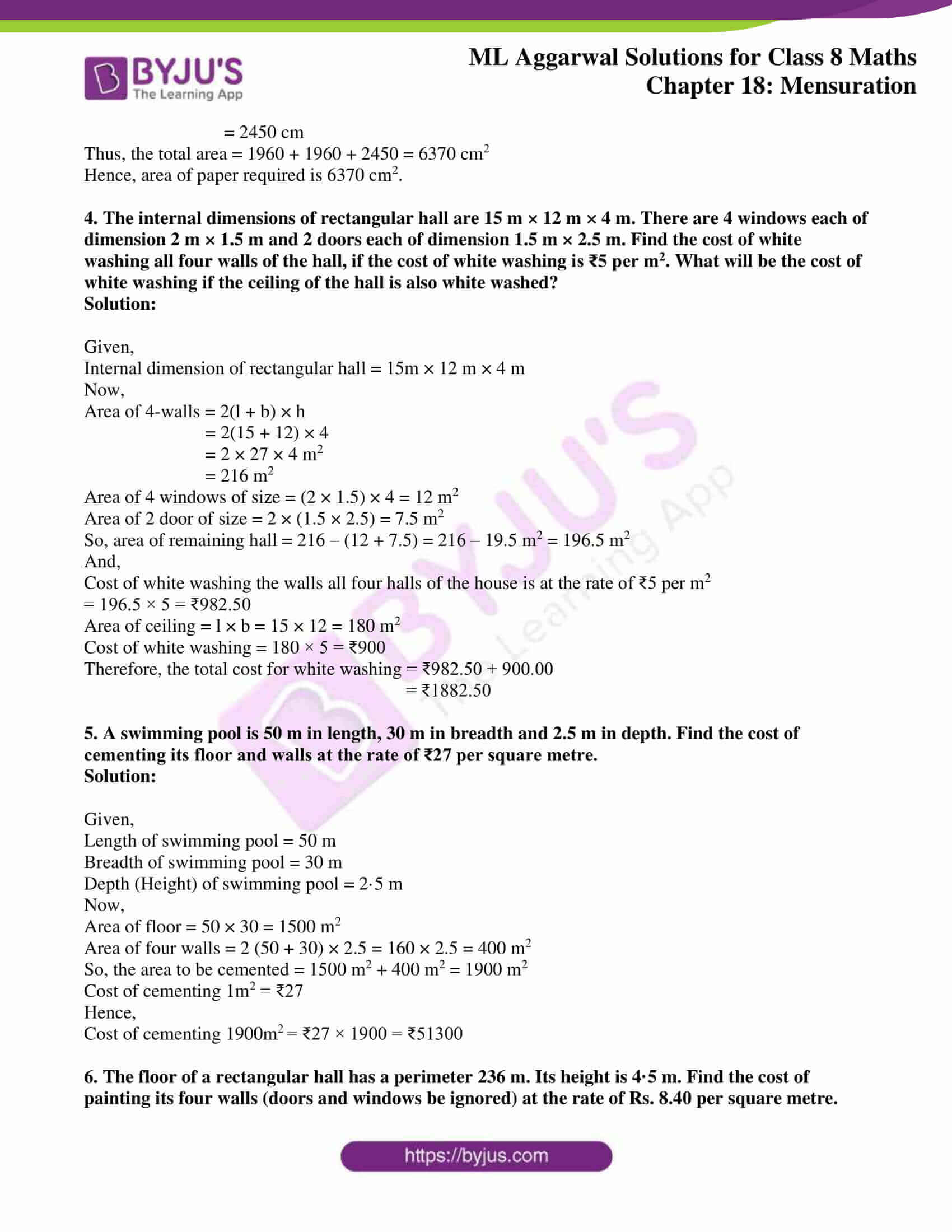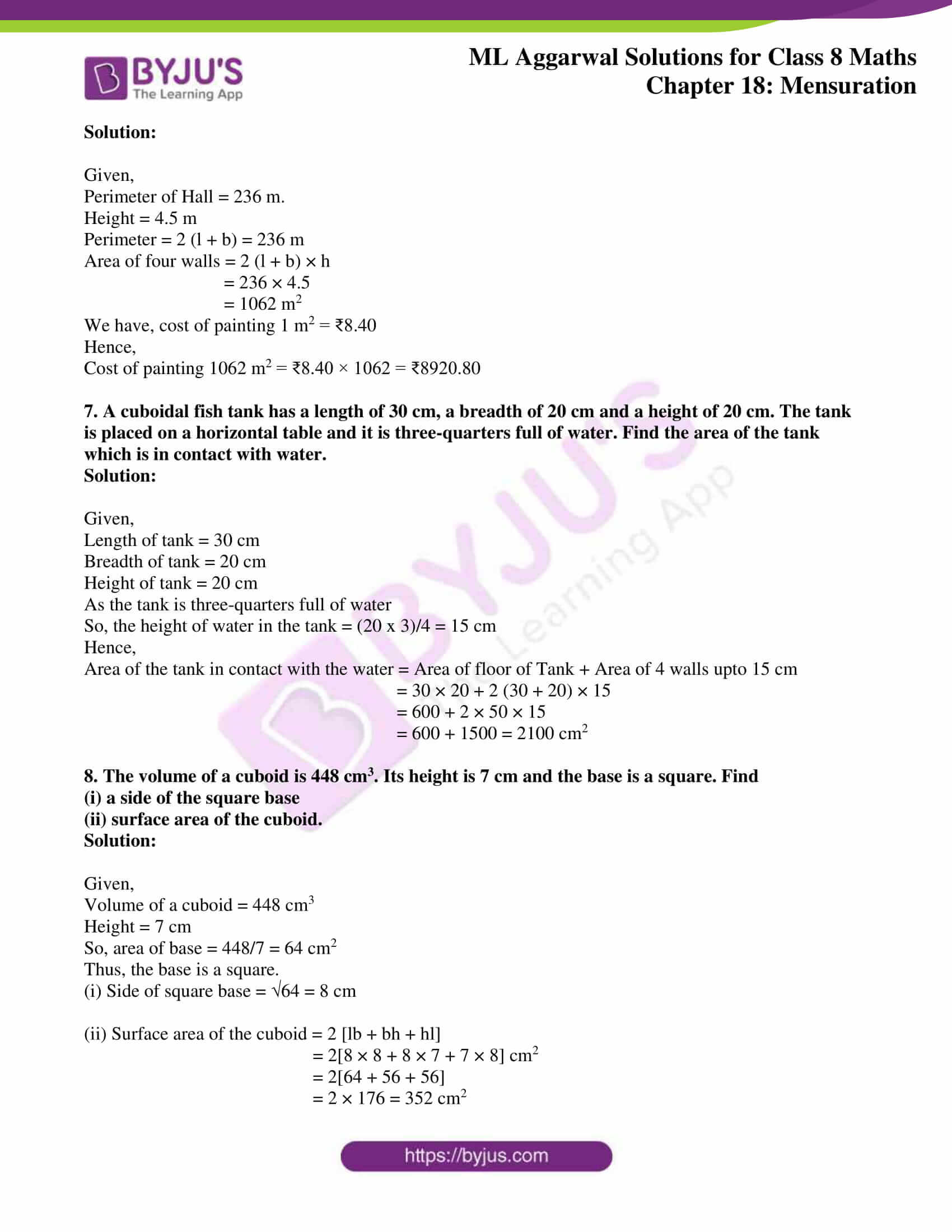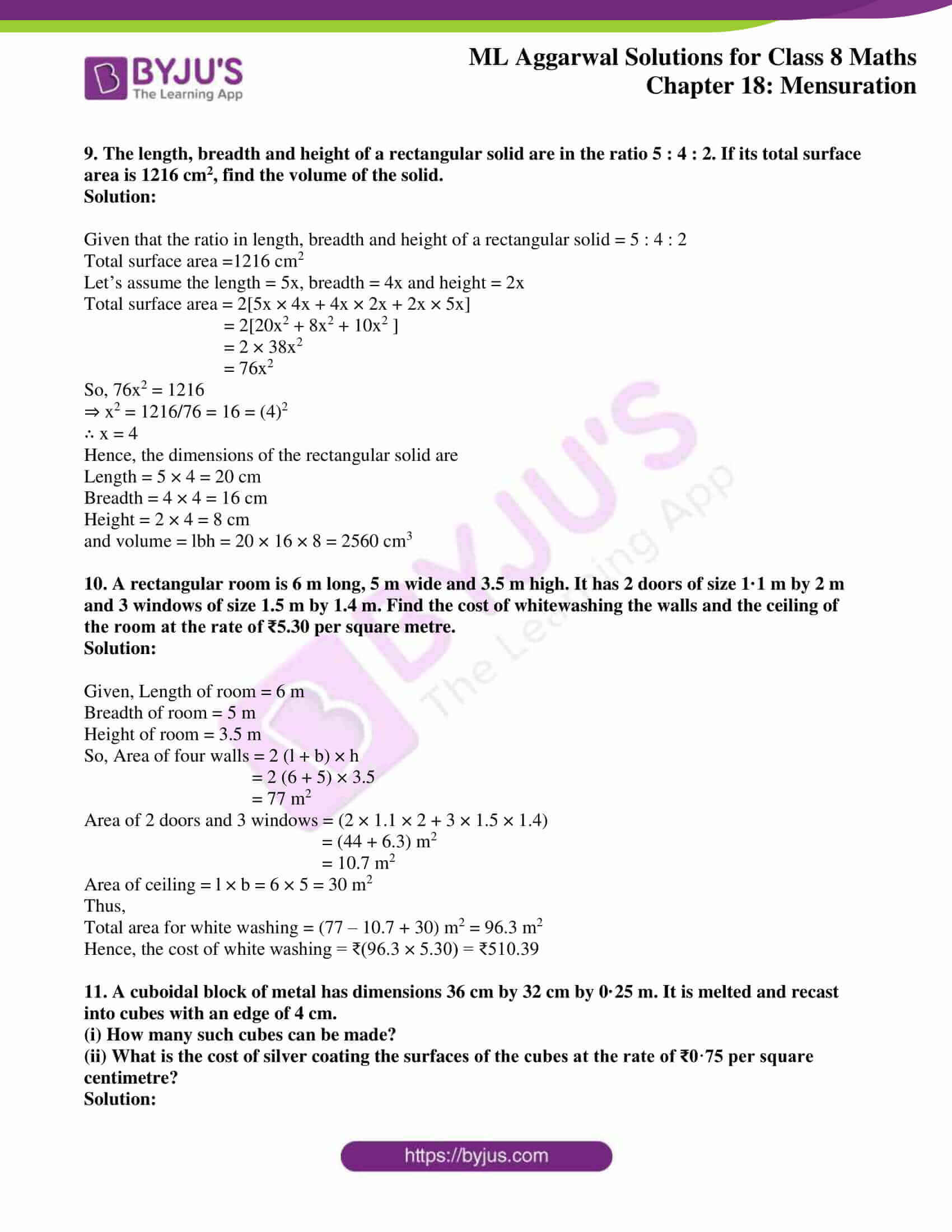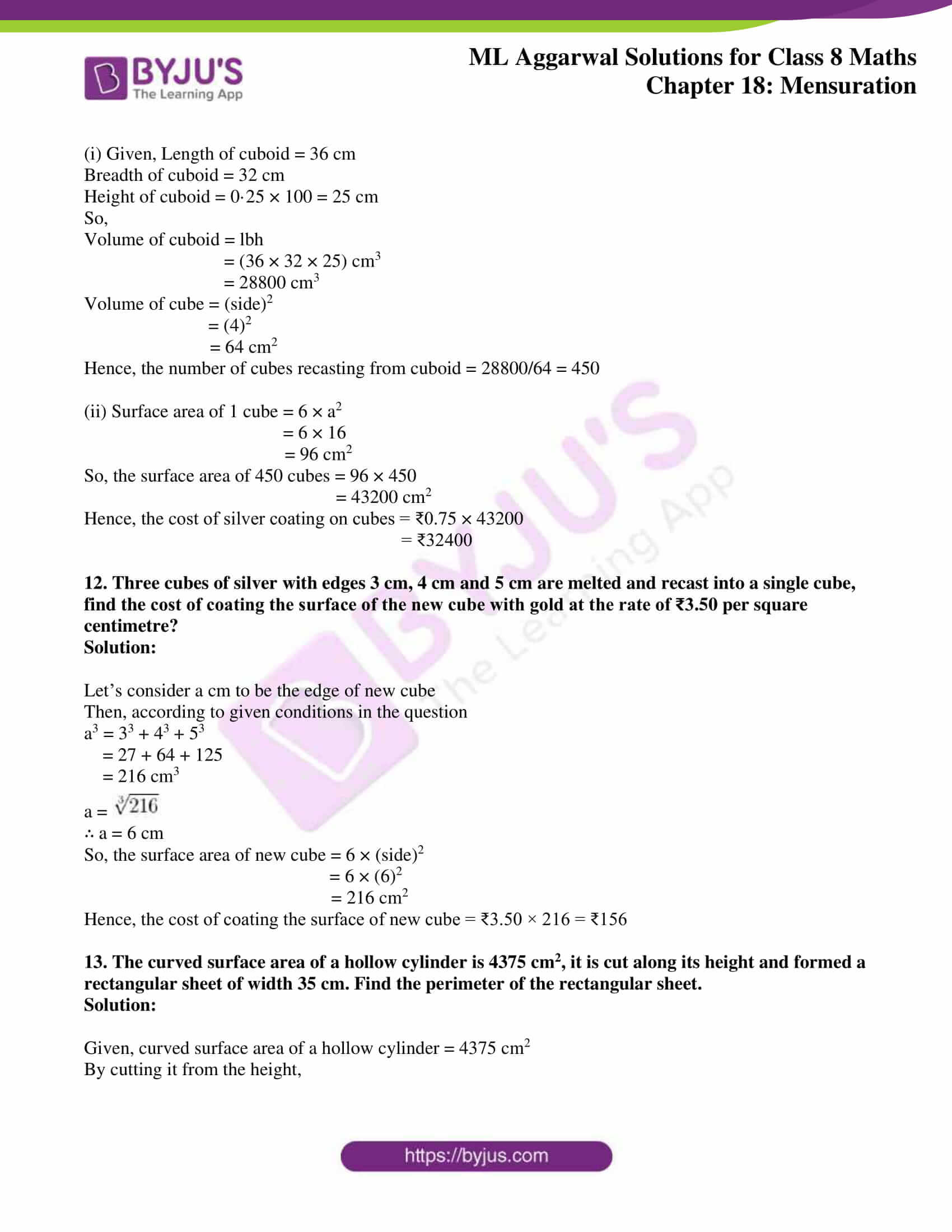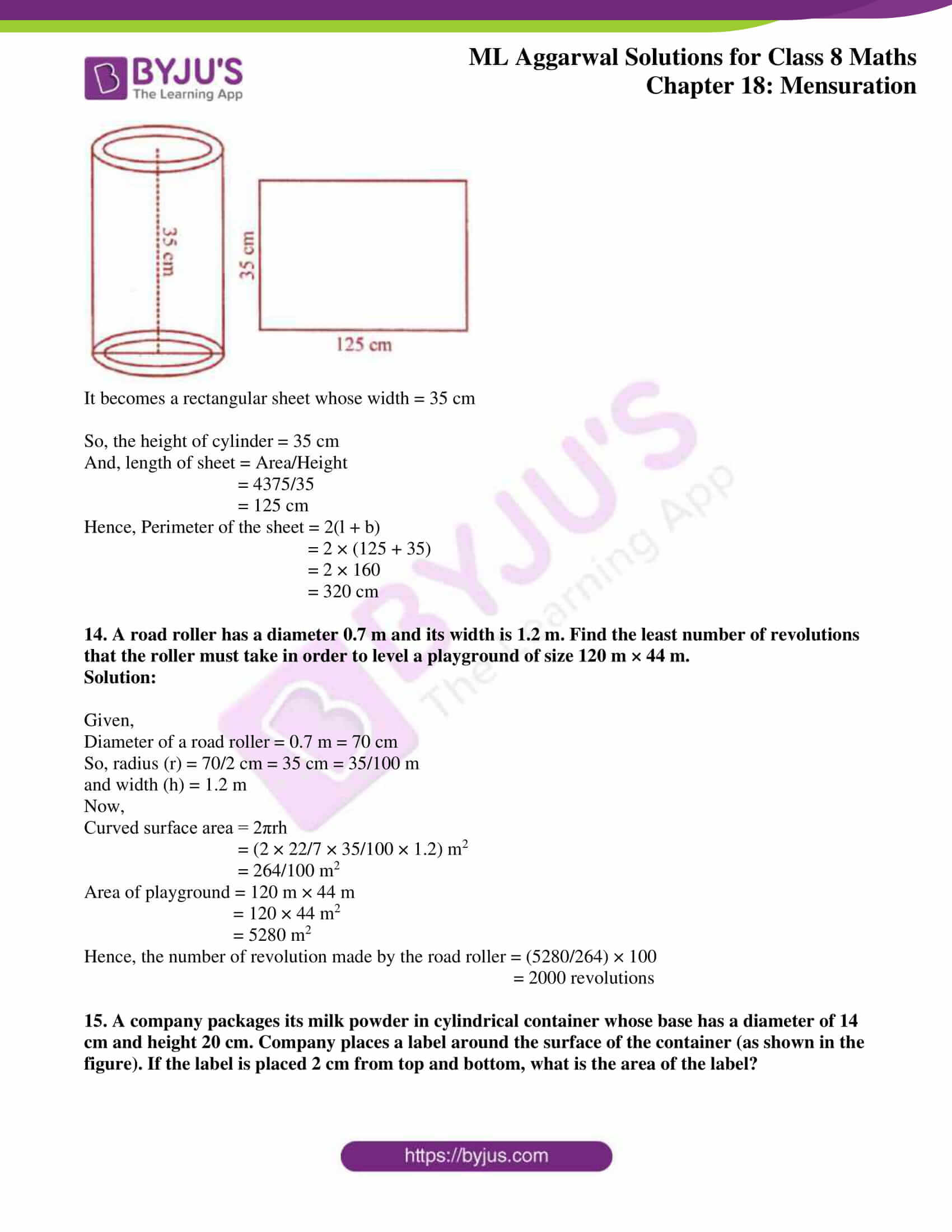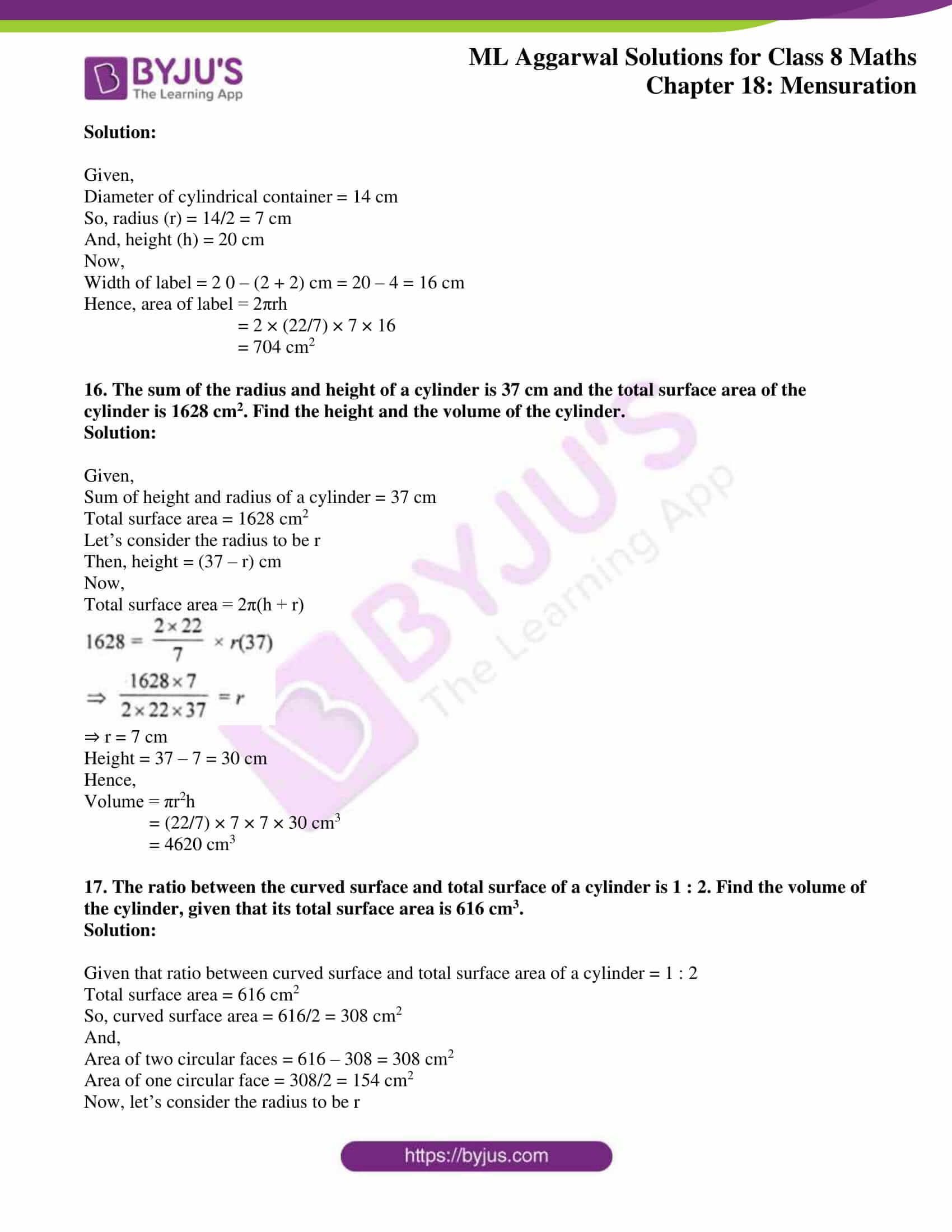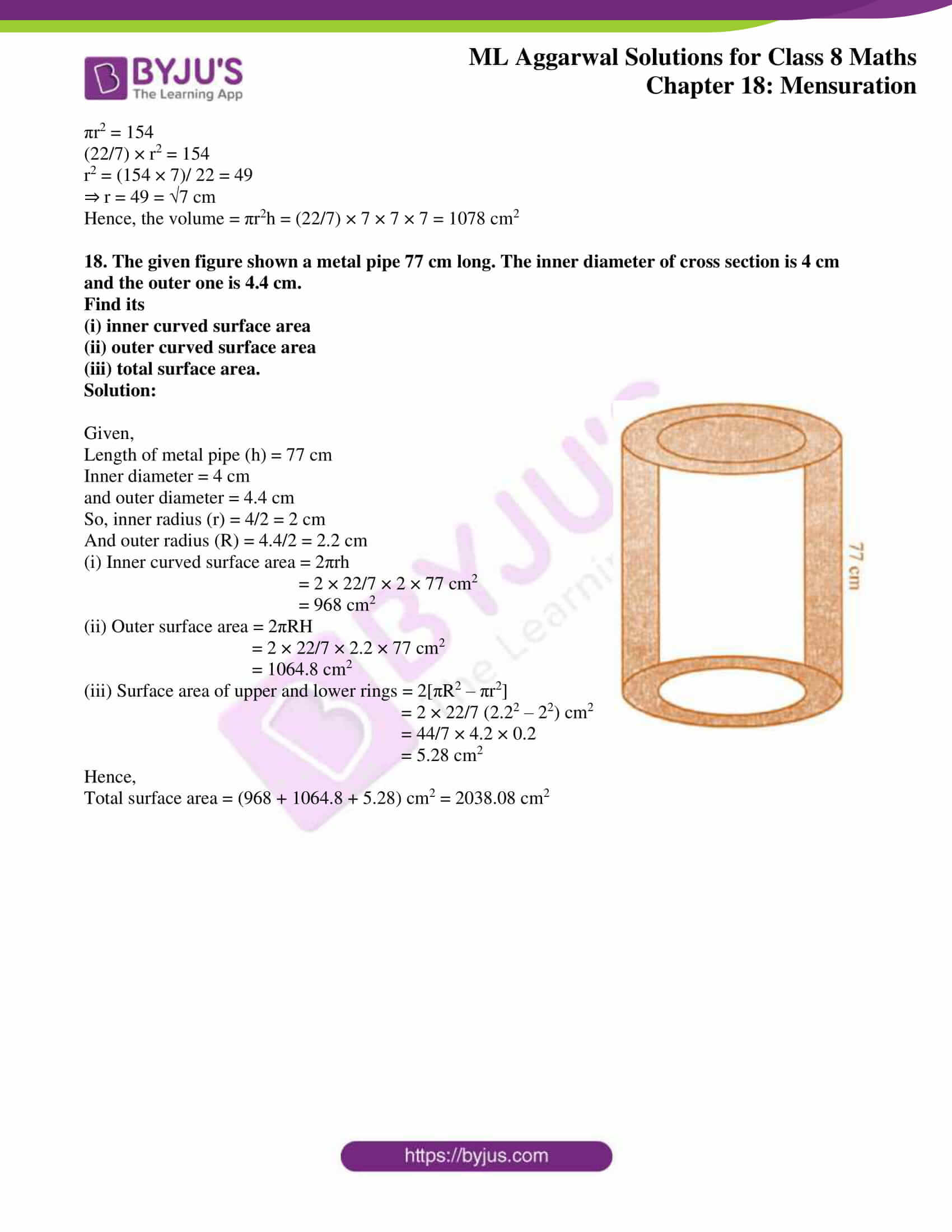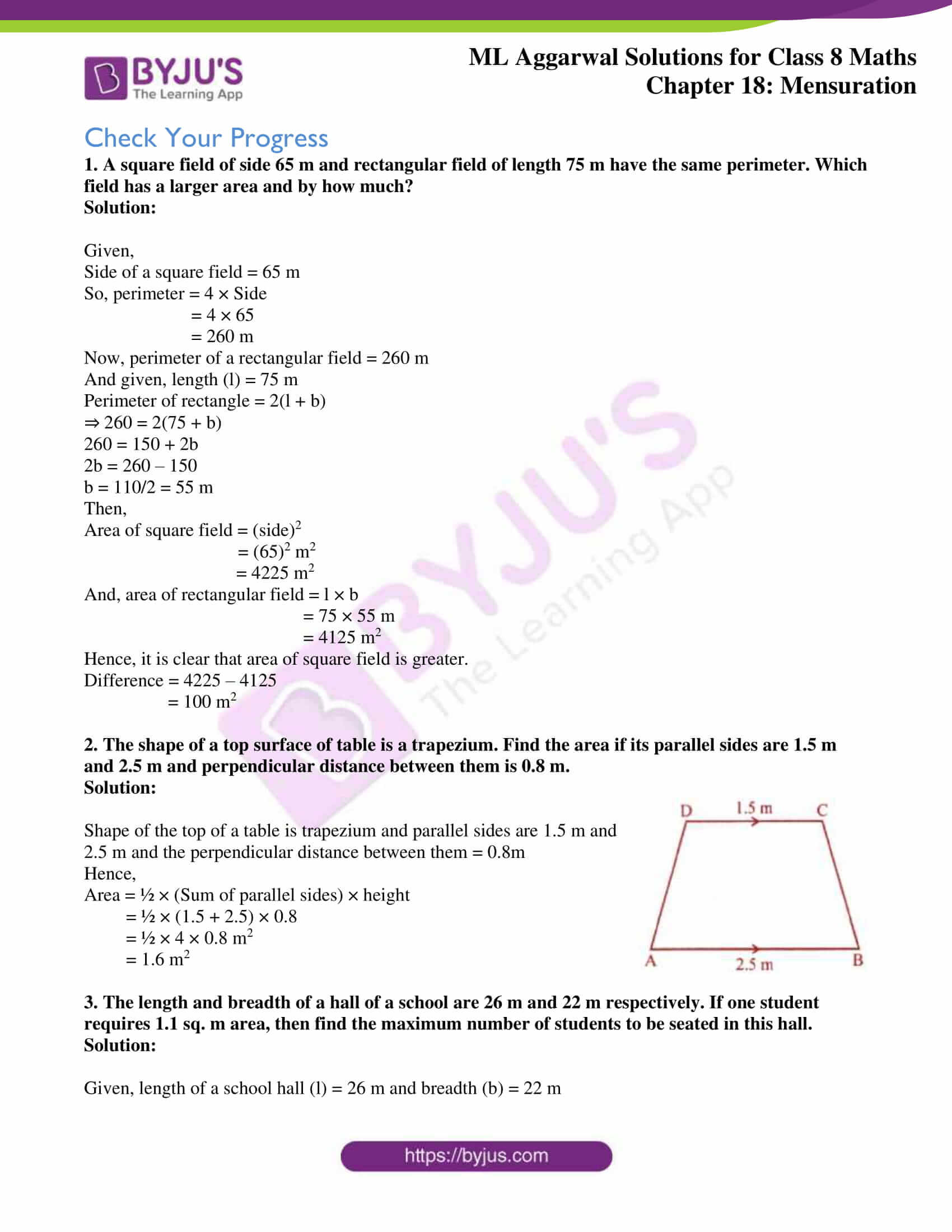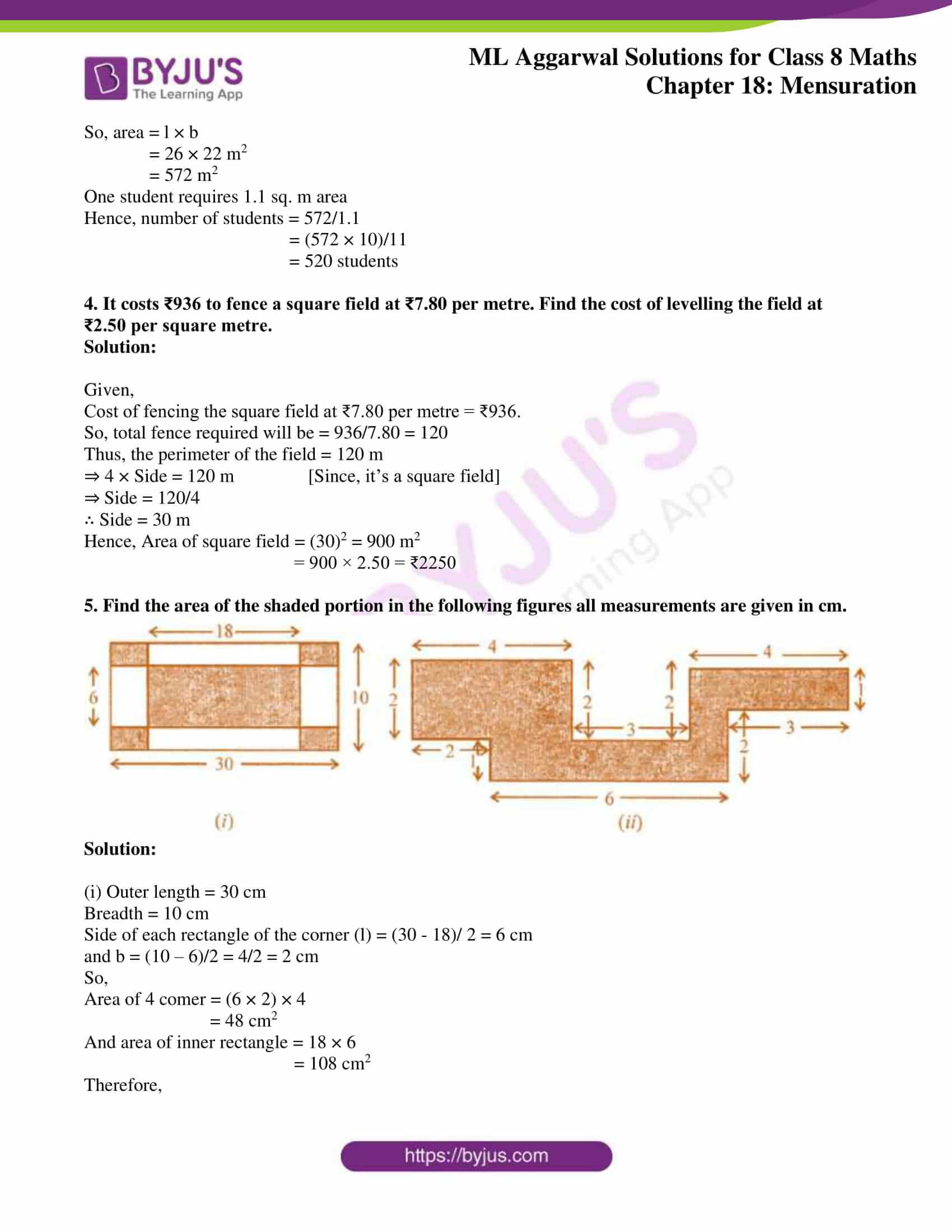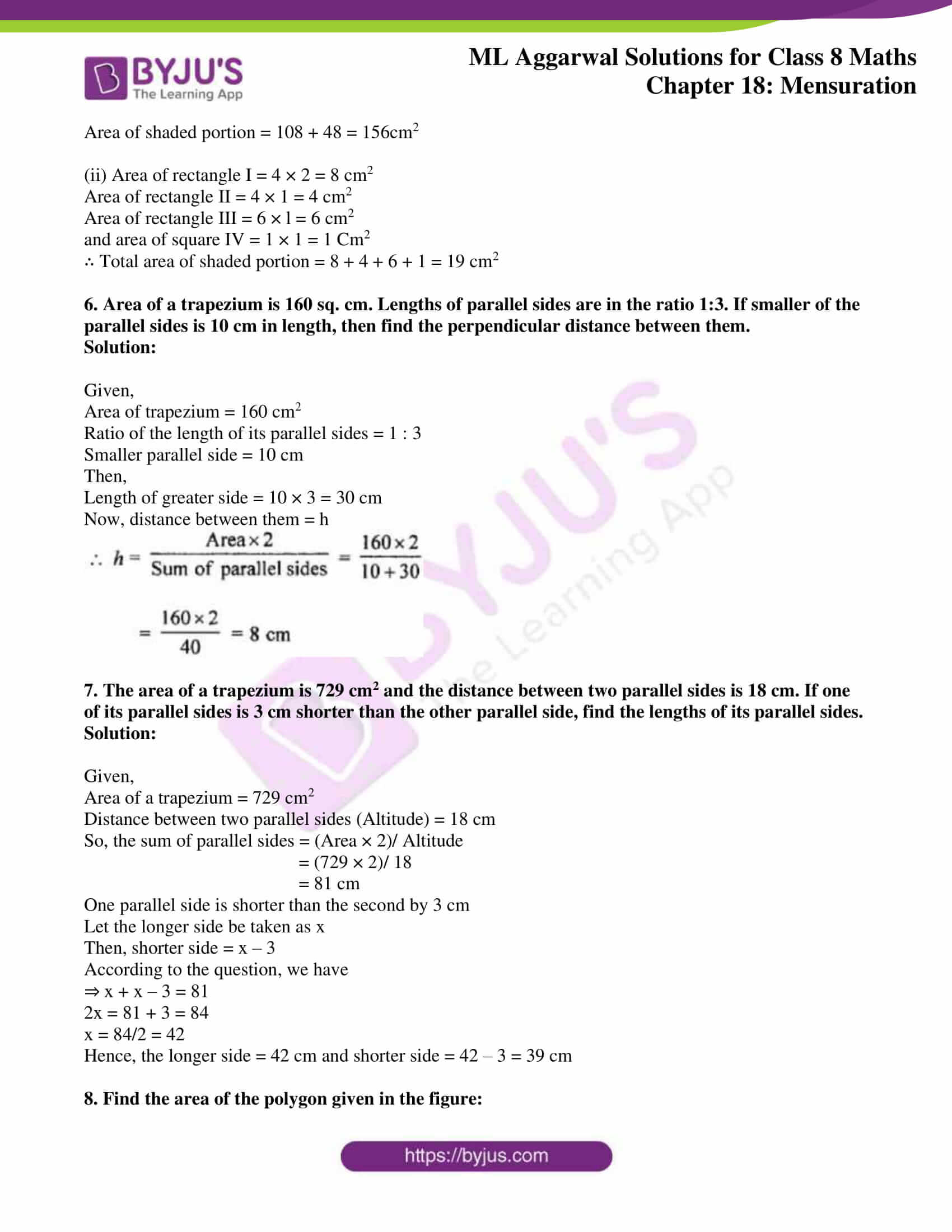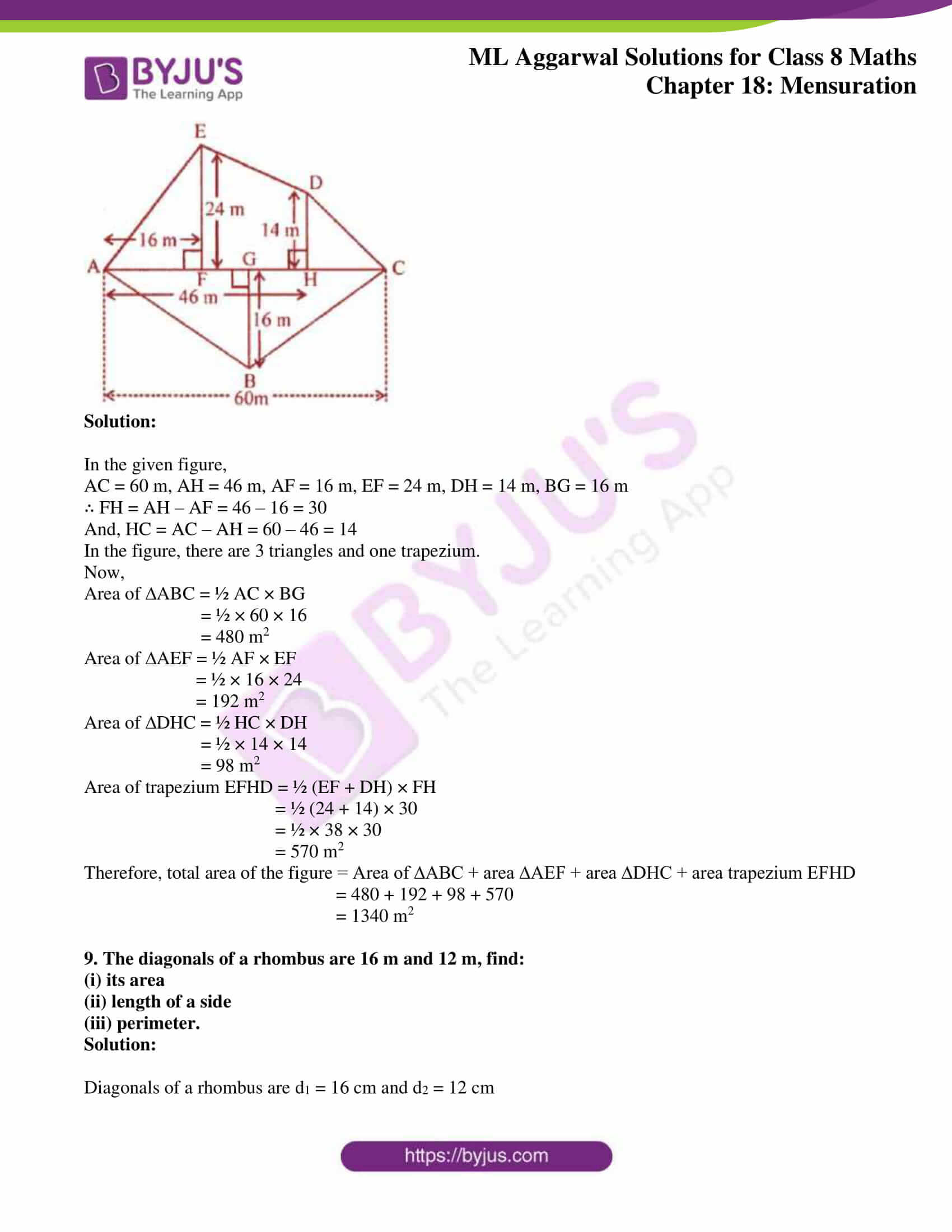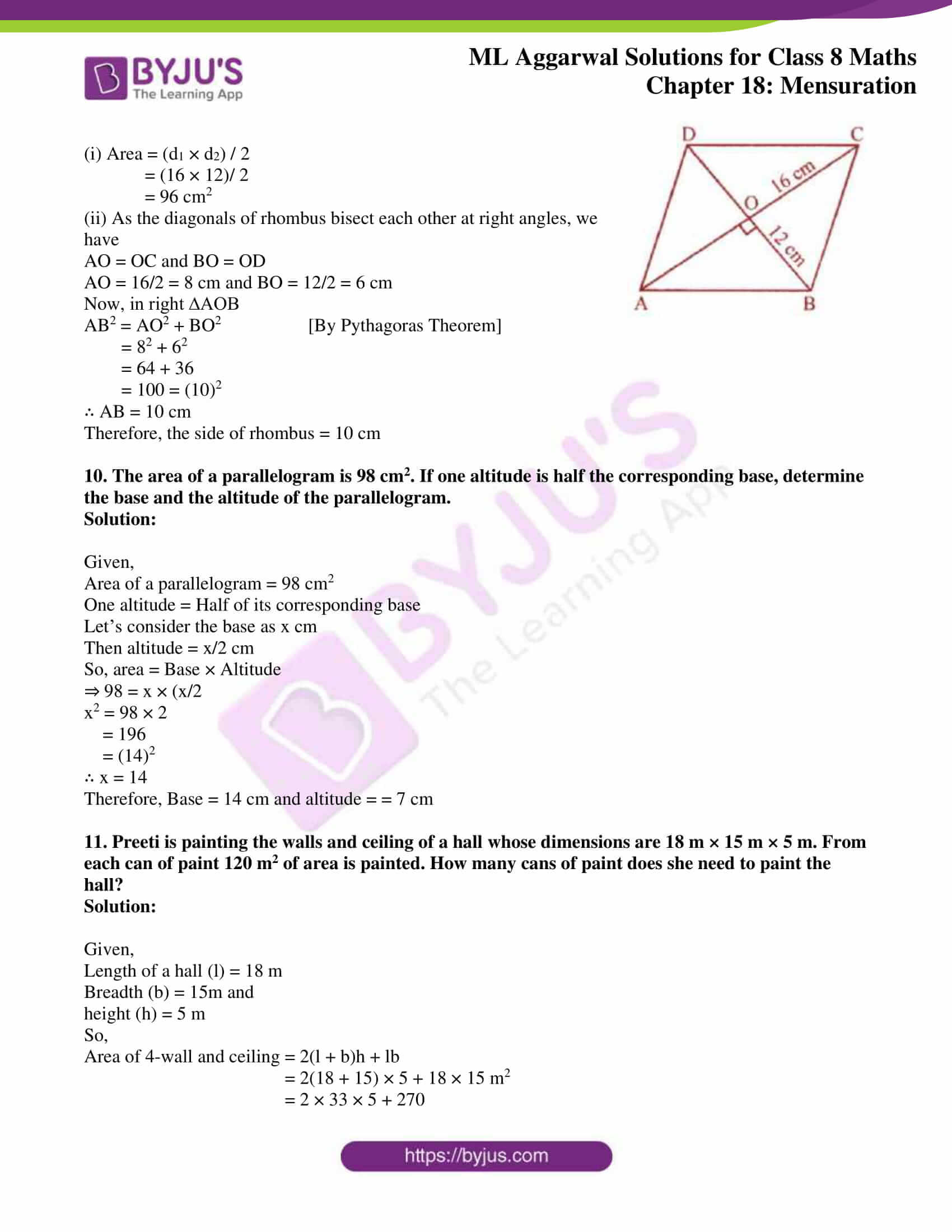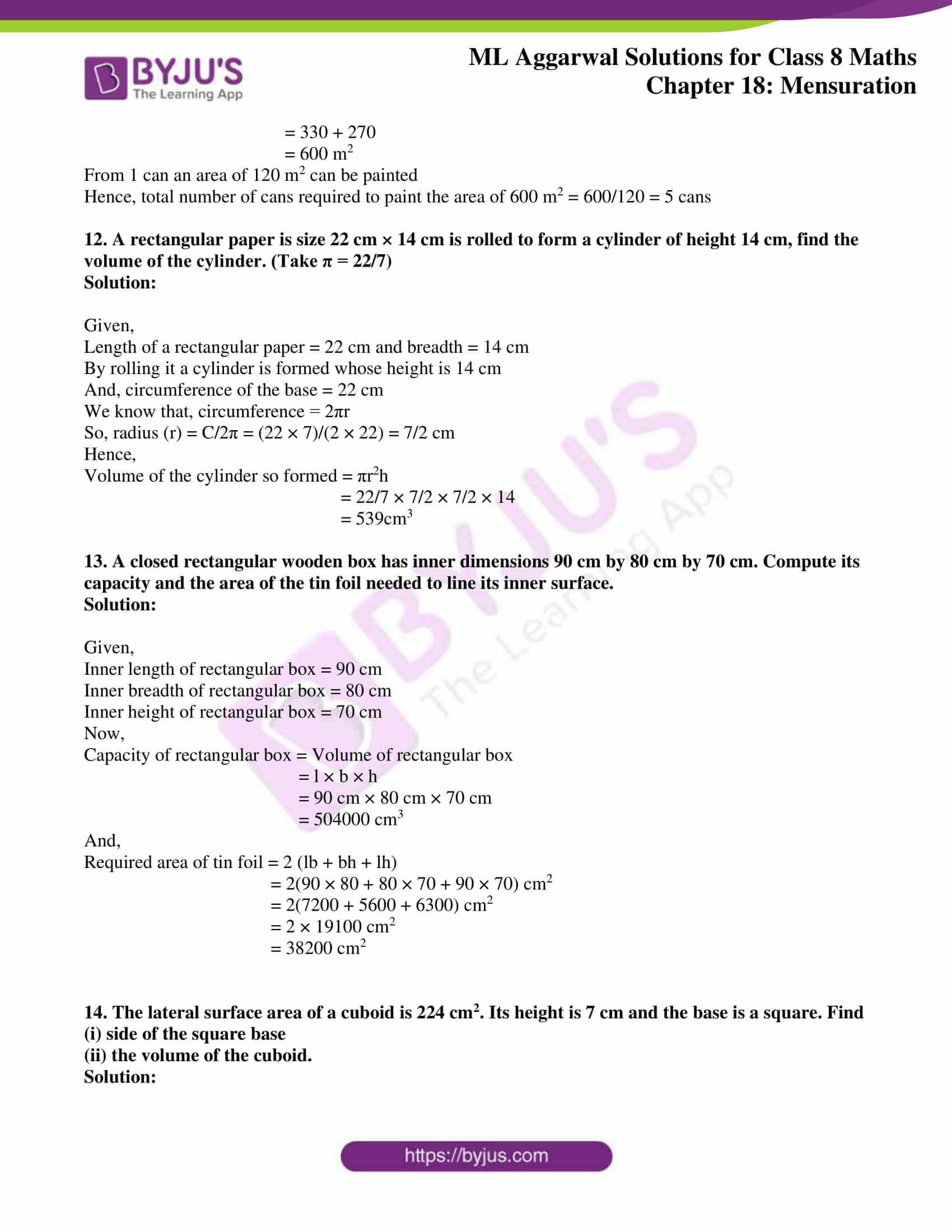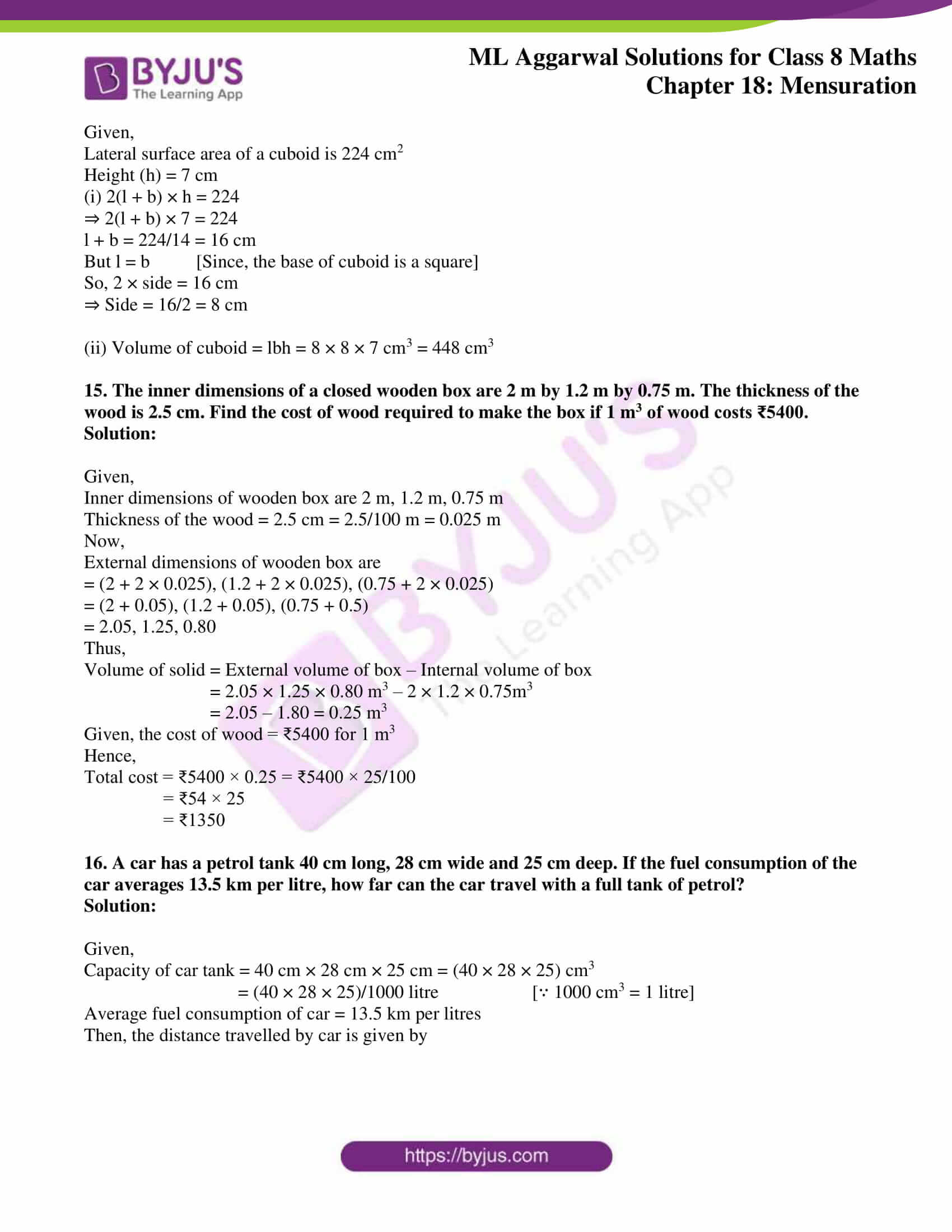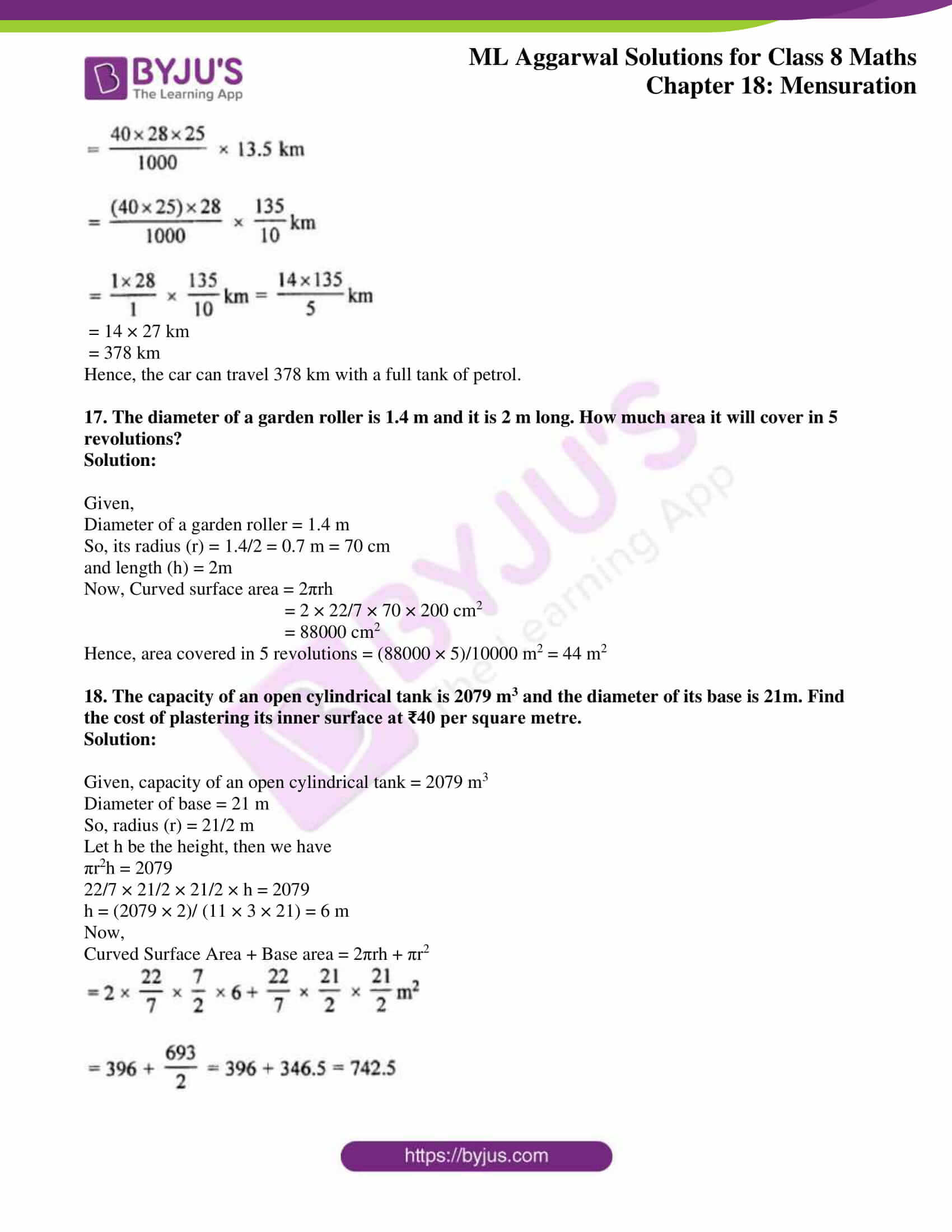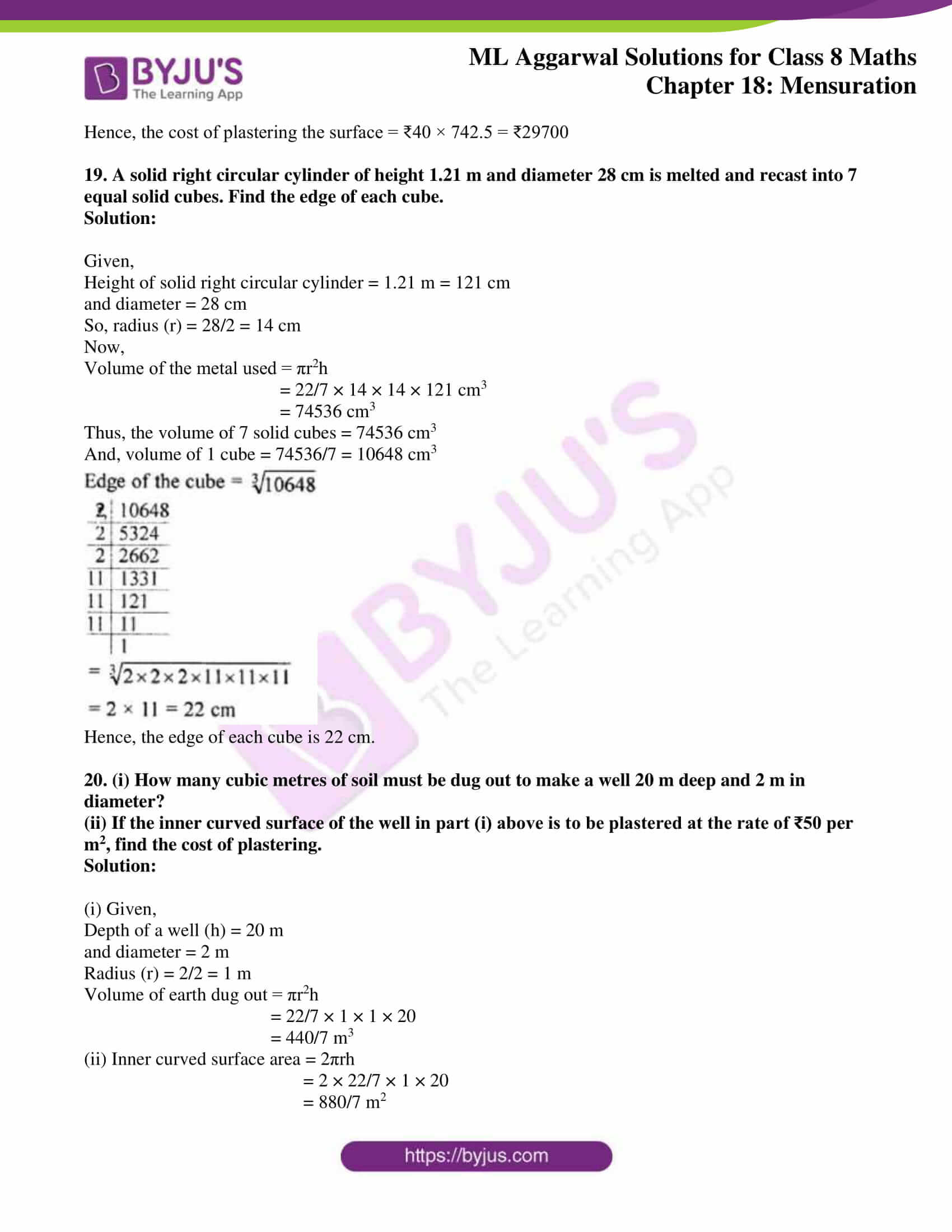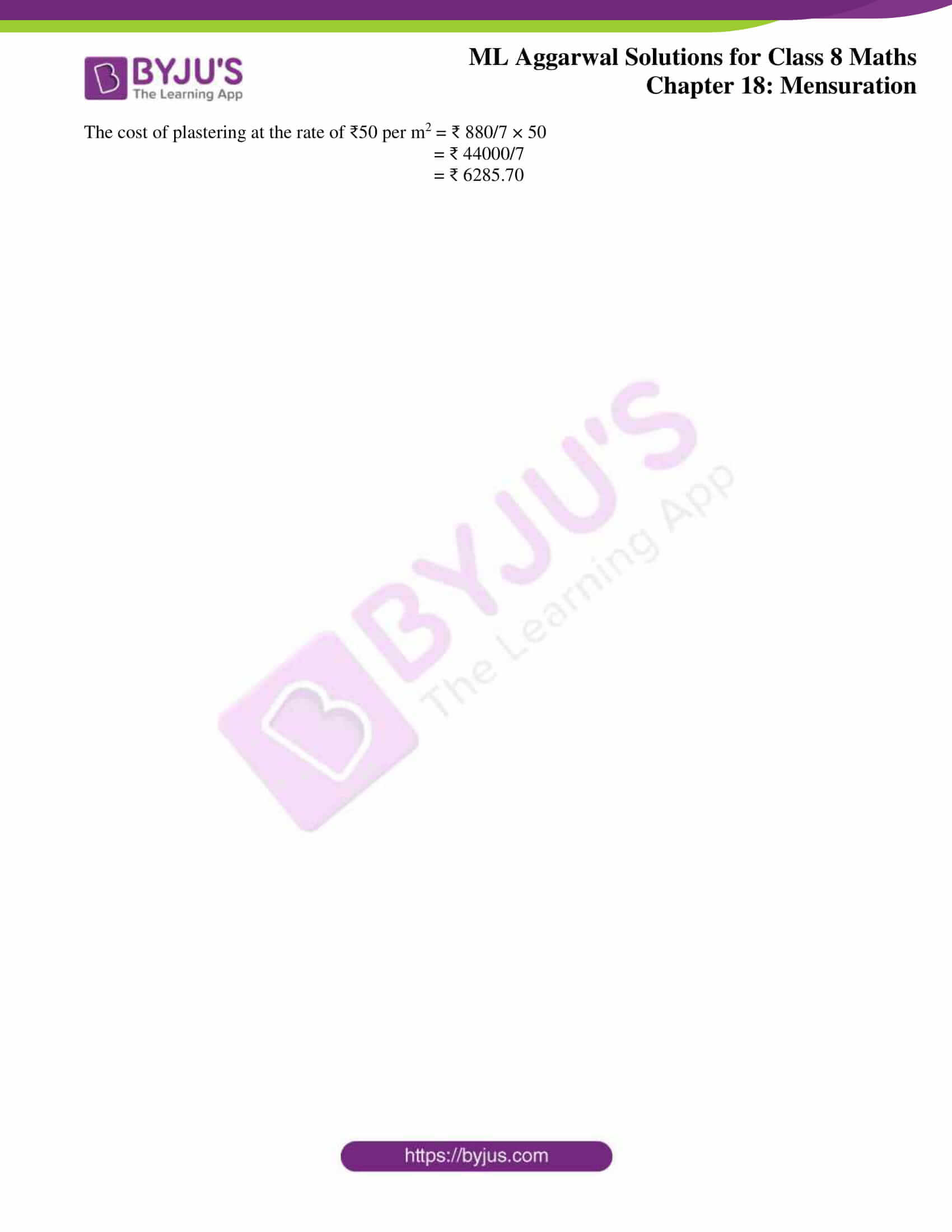### Access answers to ML Aggarwal Solutions for Class 8 Maths Chapter 18 Mensuration

Exercise 18.1

1. The length and breadth of a rectangular field are in the ratio 9 : 5. If the area of the field is 14580 square metres, find the cost of surrounding the field with a fence at the rate of ₹3.25 per metre.
Solution:

Let the length of rectangle be 9x and its breadth be 5x

So,

Area = l × b

⇒ 14580 = 9x × 5x

45x2 = 14580

x2 = 14580/45 = 324

x = √324

x = 18

Hence,

Length = 9 × 18 = 162 m and Breadth = 5 × 18 = 90 m

Now, Perimeter = 2(l + b)

= 2 (162 + 90) = 2(252)

= 504 m.

Therefore, cost for fencing the surrounding 504 m at the rate of ₹3.25 per metre = ₹(504 × 3.25) = ₹1638

2. A rectangle is 16 m by 9 m. Find a side of the square whose area equals the area of the rectangle. By how much does the perimeter of the rectangle exceed the perimeter of the square?
Solution:

Area of rectangle = (16 × 9) m2 = 144 m2

Given condition,

Area of square = Area of rectangle

∴ (Side)2 = 144

Side = √144 = 12 m

Now,

Perimeter of square = 4 × side = 4 × 12 = 48 m

Perimeter of rectangle = 2(l + b) = 2 (16 + 9) = 50 m

Hence, difference in their perimeters = 50 – 48 = 2 m

3. Two adjacent sides of a parallelogram are 24 cm and 18 cm. If the distance between longer sides is 12 cm, find the distance between shorter sides.
Solution: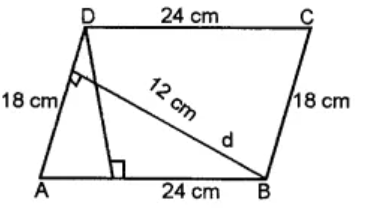Let take 24 cm as the base of parallelogram, then its height is 12 cm.

We know that,

Area of parallelogram = base × height

= 24 × 12 = 288 cm2

Let’s consider d cm to be the distance between the shortest sides.

∴ Area of parallelogram = (18 × d) cm2

18 × d = 288

⇒ d = 288/18 = 16 cm

Therefore, the distance between the shorter sides is 16 cm.

4. Rajesh has a square plot with the measurement as shown in the given figure. He wants to construct a house in the middle of the plot. A garden is developed around the house. Find the total cost of developing a garden around the house at the rate of ₹50 per m2.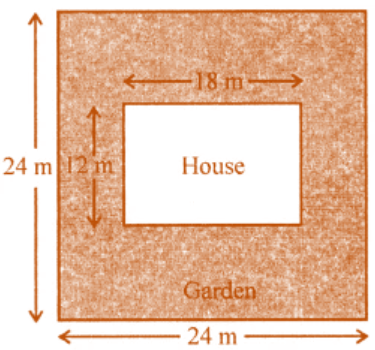Solution:

Given,

Side of square plot = 24 m

Length of house (l) = 18 m

Now,

Area of square plot = (24)2 m2 = (24 × 24) m2 = 576 m2

And,

Area of house = 18 × 12 = 216 m2

Remaining area of the garden = 576 m2 – 216 m2 = 360 m2

The cost of developing the garden = ₹50 per m2

Therefore, the total cost = ₹50 × 360 = ₹18000

5. A flooring tile has a shape of a parallelogram whose base is 18 cm and the corresponding height is 6 cm. How many such tiles are required to cover a floor of area 540 m2? (If required you can split the tiles in whatever way you want to fill up the comers).
Solution:

Given,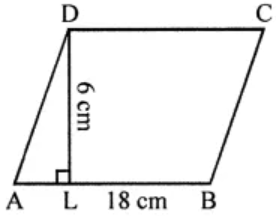Base of the parallelogram-shaped flooring tile = 18 cm and its height = 6 cm

So,

Area of one tile = Base × Height

= 18 × 6

= 108 cm2

We have the area of floor = 540 m2

Hence, number of tiles = Total area/ Area of one tile

= (540 x 100 x 100)/108  [As, 1 m2 = (100 x 100) cm2]

= 50000

6. An ant is moving around a few food pieces of different shapes scattered on the floor. For which food piece would the ant have to take a longer round?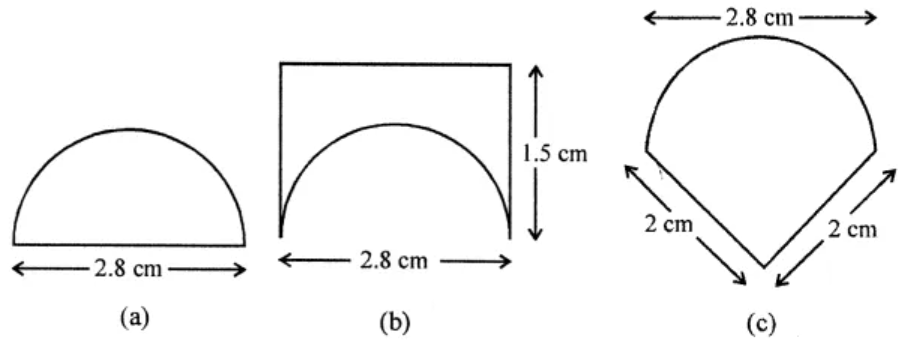Solution:

(a) Diameter of semicircle = 2.8 cm

∴ Perimeter = πr + 2r

= 22/7 × 2.8 + 2 × 2.8

= 8.8 + 5.6 cm

= 14.4 cm

(b) Total perimeter = 1.5 + 1.5 + 2.8 + semi circumference (πr, where r = 2.8/2 = 1.4 cm)

= 1.5 + 1.5 + 2.8 + (22/7 x 1.4)

= 5.8 + 8.8

= 14.6 cm

(c) Total perimeter = 2 + 2 + Semi circumference (πr, where r = 2.8/2 = 1.4 cm)

= 4 + 8.8

= 12.8 cm

Hence, it is clearly seen that distance of (b) i.e. 14.6 is the longest.

7. In the adjoining figure, the area enclosed between the concentric circles is 770 cm2. If the radius of the outer circle is 21 cm, calculate the radius of the inner circle.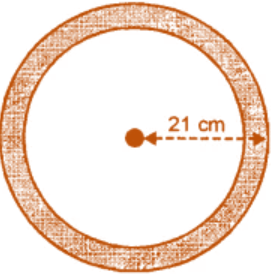Solution:

Given,

Radius of outer circle (R) = 21 cm.

Radius of inner circle (r) = r cm.

Area of shaded portion = 770 cm2

⇒ π (R2 – r2) = 770

(212 – r2) = 770

441 – r2 = 770 × (7/22) = 35 × 7 = 245

r2 = 441 – 245

r2 = 196

r = √196

∴ r = 14 cm

8. A copper wire when bent in the form of a square encloses an area of 121 cm2. If the same wire is bent into the form of a circle, find the area of the circle.
Solution:

Given,

Area of the square = 121 cm2

So, side = √121 = 11 cm

Now,

Perimeter = 4 a = 4 × 11= 44 cm

And, circumference of the circle = 44 cm

∴ Radius = (44 x 7)/ (2 x 22) = 7cm

Therefore, area of the circle = πr2 = (7)2

= 22/7 × 7 × 7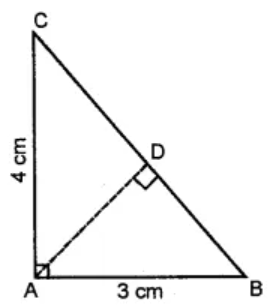= 154 cm2

9. From the given figure, find
(i) the area of ∆ ABC
(ii) length of BC
(iii) the length of altitude from A to BC

Solution:

(i) We have,

Base = 3 cm and height = 4 cm.

Hence,

Area = ½ × base × height

= ½ × 3 × 4

= 6 cm2

(ii) By Pythagoras theorem, we have

BC2 = AB2 + AC2

BC2 = (3)2 + (4)2

= 9 + 16 = 25

⇒ BC = √25 cm = 5 cm

(iii) Now,

Base = BC = 5 cm, h = AD =?

So,

Area = ½ × b × h

6 = ½ × 5 × h [∵ Area = 6 cm2 as in part (i)]

⇒ h = 12/6 = 2.4 cm.

10. A rectangular garden 80 m by 40 m is divided into four equal parts by two cross-paths 2.5 m wide. Find
(i) the area of the cross-paths.
(ii) the area of the unshaded portion.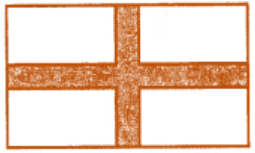Solution: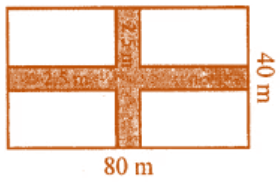Given,

Length of rectangular garden = 80 m

Width of crossing path 2.5 m

So,

Area of length wise path = 80 × 2.5 = 200 m2

and

Area of breadth wise path = 40 × 2.5 = 100 m2

(i) Total area of both paths

= 200 + 100 – 2.5 × 2.5 m2

= 300 – 6.25 = 293.75 m2

= Area of garden – Area of paths

= 80 × 40 – 293.75 m2

= 3200 – 293.75 m2

= 2906.25 m2

11. In the given figure, ABCD is a rectangle. Find the area of the shaded region.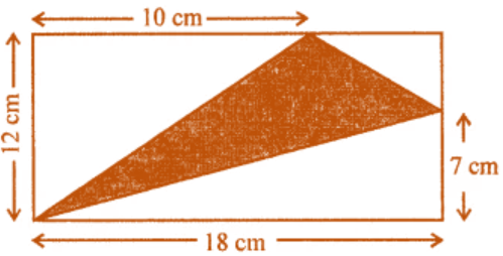Solution: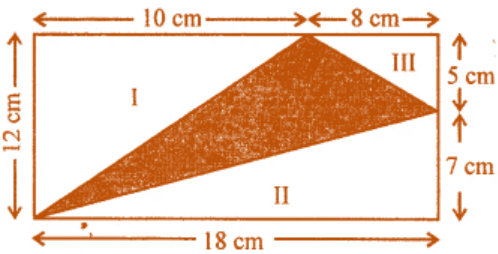In the given figure, we have

Length of rectangle = 18 cm and breadth = 12 cm

∴ Area = l × b = 18 × 12 cm2 = 216 cm2

Area of triangle I = ½ × 12 × 10 = 60 cm2

Area of triangle III = ½ × 18 × 7 = 63 cm2

Thus,

= Area of rectangle – Area of 3 triangles

= 216 – (60 + 63 + 20)

= 216 – 143 cm2

= 73 cm2

12. In the adjoining figure, ABCD is a square grassy lawn of area 729 m2. A path of uniform width runs all around it. If the area of the path is 295 m2, find
(i) the length of the boundary of the square field enclosing the lawn and the path.
(ii) the width of the path.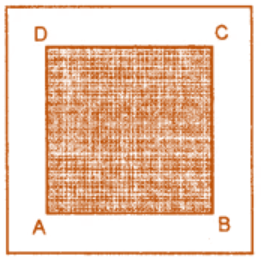Solution: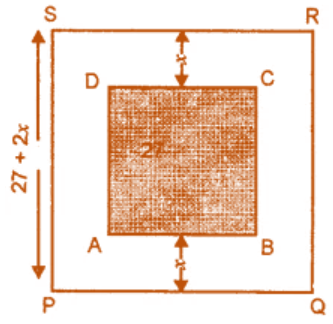Given,

Area of square ABCD = 729 m2

So, its side = √729 = 27 m

Let’s take the width of path = x m

Then,

Side of outer field = 27 + x + x = (27 + 2x) m

And, area of square PQRS = (27 + 2x)2 m2

Now,

Area of PQRS – Area of ABCD = Area of path

⇒ (27 + 2x)2 m2 – 729 m2 = 295 m2

729 + 4x2 + 108x – 729 = 295

4x2 + 108x – 295 = 0

By using the quadratic formula, we have

a = 4, b = 108 and c = -295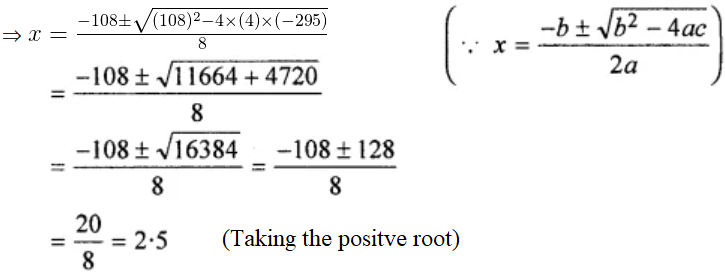Hence,

Width of the path is 2.5 m

Now, side of square field PQRS = 27 + 2x

= (27 + 2 × 2.5) m

= 32 m

Therefore,

Length of boundary = 4 × side = 32 × 4 = 128 m

Exercise 18.2

1. Each sides of a rhombus is 13 cm and one diagonal is 10 cm. Find
(i) the length of its other diagonal
(ii) the area of the rhombus
Solution: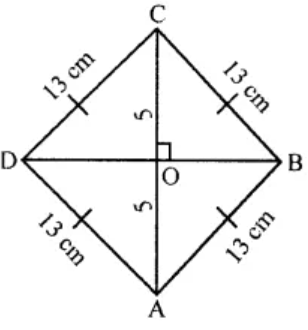(i) Given,

Side of rhombus = 13 cm.

Length of diagonal AC = 10 cm.

∴ OC = 5 cm.

Since, the diagonals of rhombus bisect each other at right angles

So, ∆BOC is rt. angled.

Then, by Pythagoras Theorem we have

BC2 = OC2 + OB2

132 = 52 + OB2

OB2 = 169 – 25 = 144

⇒ OB = √144 = 12 cm

Hence,

Diagonal BD = 2 × OB = 2 × 12 = 24 cm

(ii) Area of rhombus = ½ × d1 × d2

= ½ × 10 × 24 = 120cm2

2. The cross-section ABCD of a swimming pool is a trapezium. Its width AB = 14 m, depth at the shallow end is 1-5 m and at the deep end is 8 m. Find the area of the cross-section.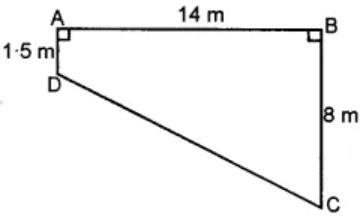Solution:

Here, AD and BC are the two parallel sides of trapezium

And, distance between them is 14 m.

∴ Area of trapezium = ½ (1·5 + 8) × 14

= ½ × 9·5 × 14

= 66 × 5 m2

3. The area of a trapezium is 360 m2, the distance between two parallel sides is 20 m and one of the parallel side is 25 m. Find the other parallel side.
Solution: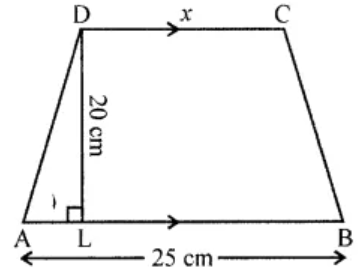Given,

Area of a trapezium = 360 m2

Distance between two parallel lines = 20 m

One parallel side = 25 m

Now,

Let’s assume the second parallel side to be x m

So, Area = (25 + x) × 20

⇒ 360 = (25 + x) × 20

∴ x = 36 – 25 = 11 m

Therefore, the second parallel side is 11 m.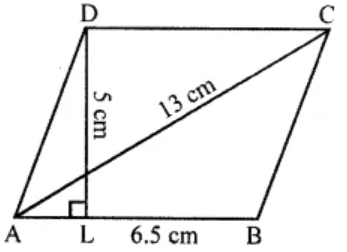4. Find the area of a rhombus whose side is 6.5 cm and altitude is 5 cm. If one of its diagonal is 13 cm long, find the length of other diagonal.
Solution:

Given,

Side of rhombus = 6.5 cm

And altitude = 5 cm

So,

Area of a rhombus = Side × Altitude = 6.5 × 5 = 32.5 cm2

We have, one diagonal = 13 cm

Hence,

Length of other diagonal = (2 x Area)/ One diagonal

= (32.5 x 2)/ 13

= 5 cm

5. From the given diagram, calculate
(i) the area of trapezium ACDE
(ii) the area of parallelogram ABDE
(iii) the area of triangle BCD.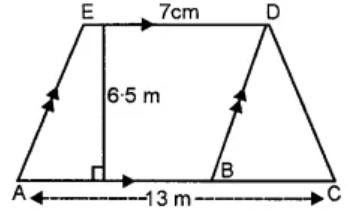Solution:

(i) Area of trapezium ACDE = ½ × (AC + DE) × h

= ½ × (13 + 7) × 6.5

= ½ × 20 × 6.5

= 65 m2

(ii) Area of parallelogram ABDE = ½ × b × h

= ½ × 6 × 6.5

= 15.5 m2

(iii) Area of ∆BCD = ½ × base × height

= ½ × BC × height

= ½ × 6 × 6.5 [∵ BC = AC – AB = 13 – 7 = 6 m]

= 19.5 m2

6. The area of a rhombus is equal to the area of a triangle whose base and the corresponding altitude are 24.8 cm and 16.5 cm respectively. If one of the diagonals of the rhombus is 22 cm, find the length of the other diagonal.
Solution:

Given,

Base of triangle = 24.8 cm and altitude = 16.5 cm

Area = ½ × base × altitude

= ½ × 24.8 × 16.5 cm2

= 204.6 cm2

Now, Area of ∆ = Area of rhombus

But, area of rhombus = 204.6 cm2

Length of one diagonal = 22 cm

Area of rhombus = (First diagonal × Second diagonal)

Thus,

Second diagonal = (2 × Area)/ First diagonal

= (204.6 × 2)/ 22

= 18.6 cm

7. The perimeter of a trapezium is 52 cm. If its non-parallel sides are 10 cm each and its altitude is 8 cm, find the area of the trapezium.
Solution: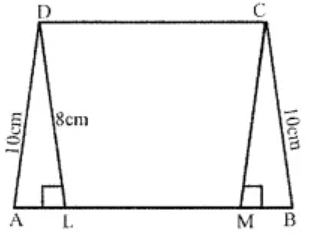Given,

Perimeter of a trapezium = 52 cm

Length of each non-parallel side = 10 cm

Altitude DL = 8 cm

Now,

In right ∆DAL, by Pythagoras Theorem we have

DA2 = DL2 + AL2

(10)2 = (8)2 + AL2

100 = 64 + AL2

AL2 = 100 – 64 = 36 = (6)2

∴ AL = 6 cm

Similarly,

BM = 6 cm and DC = LM

Also, we have

Perimeter = AB + BC + CD + DA

and CD = DA

So, CD + DA = 2DA

But,

AB + CD = Perimeter – 2 AD

= 52 – 2 × 10

= 52 – 20

= 32 cm

Thus, area of trapezium = ½ × (sum of parallel sides) × altitude

= ½ × 32 × 8

= 128 cm2

8. The area of a trapezium is 540 cm2. If the ratio of parallel sides is 7 : 5 and the distance between them is 18 cm, find the lengths of parallel sides.
Solution:

Let’s assume the two parallel sides of trapezium to be 7x and 5x.

Height = 18 cm

Now,

Area of trapezium = ½ × [Sum of || gm sides × height]

⇒ 540 = ½ × (7x + 5x) × 18

540 = ½ × 12x × 18

540 = 108x

x = 540/108

x = 5 cm

Hence, the two parallel sides are:

7x = 7 × 5 = 35 cm and 5x = 5 × 5 = 25 cm

9. Calculate the area enclosed by the given shapes. All measurements are in cm.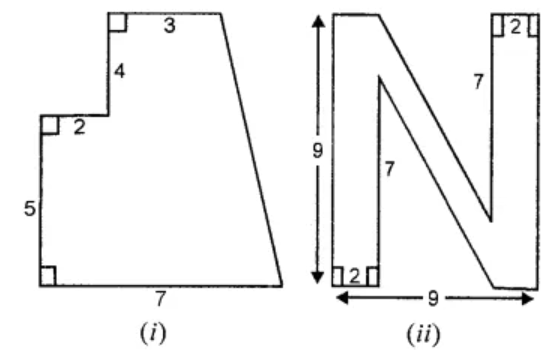Solution:

(i) Firstly,

Area of trapezium ABCD

= (Sum of opposite ||gm sides) × height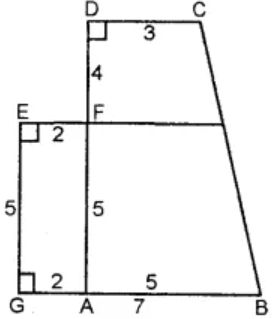= [(AB + CD) × (AF + FD)]

= [(AB + CD) × (AF + FD)

= [(5 + 3) × (5 + 4)]

= (5 + 3) × 9

= 36 cm2

Secondly,

Area of rectangle GAFE = Length × Breadth

= 2 × 5 = 10 cm2

Hence,

Total area of the figure = Area of trapezium ABCD + Area of rectangle GAFE

= (36 + 10) cm2

= 46 cm2+

(ii) It’s seen that,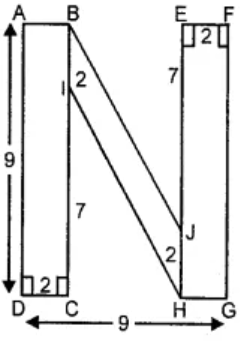Area of given figure = Area of rect. ABCD + Area of || gm BIHJ + Area of rectangle EFGH

Area of rectangle ABCD = Length × Breadth

= 9 × 2 = 18 cm2

And,

Area of rectangle EFGH = Length × Breadth

= (EJ + JH) × EF

= (7 + 2) × 2

= 9 × 2 = 18 cm2

Now,

Area of parallelogram BIHJ = 2 × 5 = 10 cm2

[Since, distance between BI and HJ = 9 – 2 – 2 = 5 cm]

Hence,

Total area of the figure = (18 + 18 + 10) cm2 = 46 cm2

10. From the adjoining sketch, calculate
(ii) the area of trapezium ABCD
(iii) the area of triangle BCD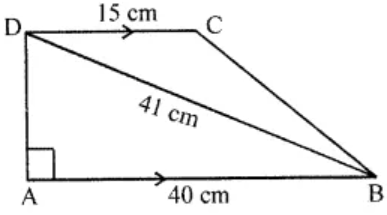Solution:

(i) In right angled ∆ ABD, by Pythagoras Theorem we have

⇒ AD2 = BD2 – AB2

= (41)2 – (40)

= 1681 – 1600

= 81

∴ AD = √81 = 9 cm

(ii) Area of trapezium ABCD

= (Sum of opposite || gm lines) × height

= (AB + CD) × AD

= (40+ 15) × 9

= 247.5 cm2

(iii) Area of triangle BCD = Area of trapezium ABCD – Area of ∆ ABD

= (247.5 – × 40 × 9) cm2

= (247.5 – 180) cm2

= 67·5 cm2

11. Diagram of the adjacent picture frame has outer dimensions = 28 cm × 32 cm and inner dimensions 20 cm × 24 cm. Find the area of each section of the frame, if the width of each section is same.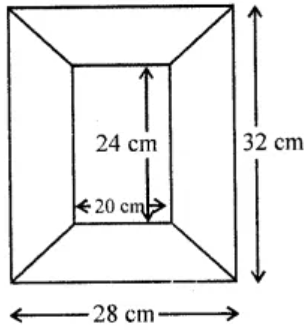Solution:

Given,

Outer length of the frame = 32 cm and outer breadth = 28 cm

Inner length = 24 cm and outer breadth = 20 cm

So, width of the frame = (32 – 24)/ 2 = 4 cm

⇒ Height = 4 cm

Now, area of each portion of length side

= ½ × (24 + 32) × 4

= ½ × 56 × 4

= 112 cm2

And,

Area of each portion of breadth side

= ½ × (20 + 28) × 4

= ½ × 48 × 4

= 96 cm2

Therefore,

Area each section are 112 cm2, 96 cm2, 112 cm2, 96 cm2.

12. In the given quadrilateral ABCD, ∠BAD = 90° and ∠BDC = 90°. All measurements are in centimetres. Find the area of the quadrilateral ABCD.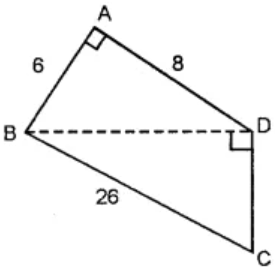Solution:

In right angled triangle ABD, by Pythagoras Theorem we have

BD2 = AB2 + AD= (6)2 + (8)2

= 36 + 64

= 100 cm2

∴ BD = √100 = 10 cm

Now, Area of ∆ABD = ½ × b × h

= ½ × 6 × 8

= 24cm2 …(i)

In ∆ BDC, we have

BD = 10 cm, BC = 26 cm

DC = ?

By Pythagoras theorem,

BC2 = BD2 + DC2

(26)2 = (10)2 + DC2

676 – 100 = DC2

⇒ DC = √576 = 24 cm.

Now,

Area of ∆ BDC = ½ × b × h

= ½ × 24 × 10

= 12 cm2 …(ii)

Adding (i) and (ii), we get

Area of ∆ABD + Area of ∆BDC = (24 + 120) cm2

Hence,

Area of quadrilateral ABCD = 144 cm2

13. Top surface of a raised platform is in the shape of a regular octagon as shown in the given figure. Find the area of the octagonal surface.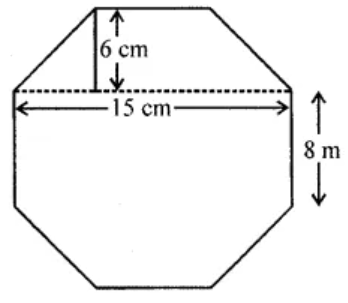Solution:

The raised surface of platform is in the shape of regular octagon ABCDEFGH of each side = 8 cm.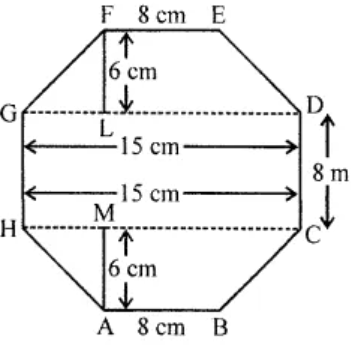Join HC.

GD = HC = 15 cm, FL = AM = 6 cm

Now, in each trapezium parallel sides are 15 cm and 6 cm and height = 6 cm

So, Area of each trapezium FEDG = ½ (GD + FE) × FL

= ½ (15 + 8) × 6

= 23 × 3 cm2

= 69 cm2

Also,

Area of trapezium FEDG = Area of trapezium ABCH = 69 cm2

And area of rectangle HCDG = HC × CD

= 15 × 8

= 120 cm2

Hence,

Total area = Area of trapezium FEDG + Area of trapezium ABCH + Area of rectangle HCDG.

= 69 + 69 + 120

= 258 cm2

14. There is a pentagonal shaped park as shown in the following figure:
For finding its area Jaspreet and Rahul divided it in two different ways.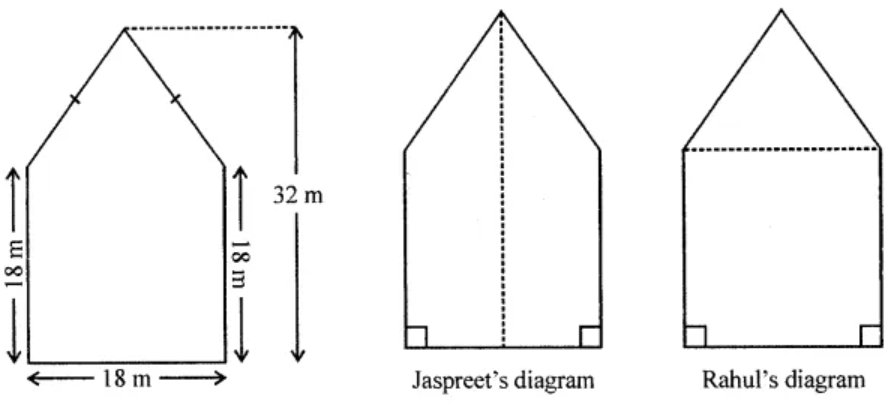Find the area of this park using both ways. Can you suggest some other way of finding its area?
Solution:

The pentagonal shaped park is shown in the given figure.

In which DL ⊥ CE and is produced to M.

So, DM = 32 m

LM = CB = 18 m

∴ DL = 32 – 18 = 14 m

(i) According to Jaspreet’s the figure is divided into two equal trapezium in area: DEAM and DCBM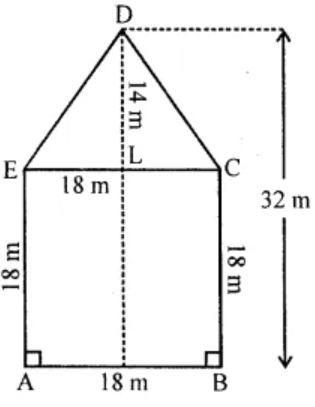Now,

Area of trapezium DEAM = ½ (AE + DM) × AM

= ½ (32 + 18) × 9

= (50 x 9)/ 2

= 225m2

(ii) According to Rahul’s the figure is divided into shapes: one square and on isosceles triangle.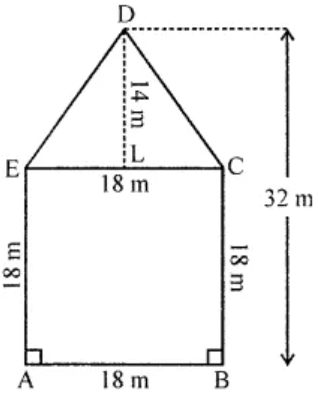Area of square ABCE = (Side)

= (18)2

= 324 m2

And, area of isosceles ∆EDC = ½ × EC × DC

= ½ × 18 × 14

= 126 m2

∴ Total area = 225 × 2 = 450 m2

The third way to find out the area of given figure is as follow: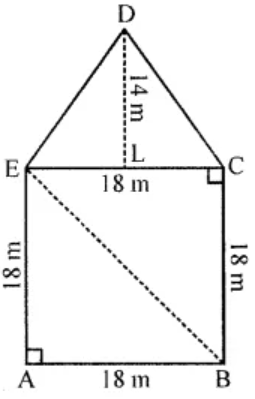Here, DL ⊥ ED and DL = 14 m

Area of ∆DEC = ½ × EC × LD

= ½ × 18 × 14

= 126 m2

Area of ∆AEB = ½ × AB × AE

= ½ × 18 × 18

= 162 m2

Area of ∆BEC = ½ × BC × EC

= ½ × 18 × 18 = 162 m2

Hence, area of pentagon ABCDE = Area ∆DEC + Area of ∆AEB + Area of ∆BEC

= (126 + 162 + 162) m2

= 450 m2

15. In the diagram, ABCD is a rectangle of size 18 cm by 10 cm. In ∆ BEC, ∠E = 90° and EC = 8 cm. Find the area enclosed by the pentagon ABECD.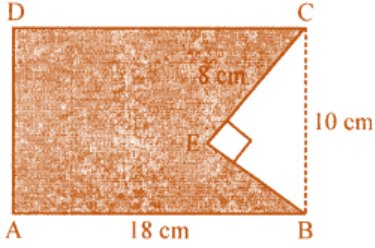Solution:

Area of rectangle ABCD = Length × Breadth

= 18 × 10

= 180 cm2

In right angled ∆ BEC,

By Pythagoras theorem, we have

BC2 = CE2 + BE2

(10)2 = 82 + BE2

BE2 = 100 – 64 = 36

⇒ BE = √36 = 6 cm.

So,

Area of rt. ∆ BEC = ½ × 6 × 8

= 24cm2

Area of pentagon ABECD = Area of rectangle – area of ∆

= (180 – 24) cm2

= 156 cm2

16. Polygon ABCDE is divided into parts as shown in the given figure. Find its area if AD = 8 cm, AH = 6 cm, AG = 4 cm, AF = 3 cm and perpendiculars BF = 2 cm, CH = 3 cm, EG = 2.5 cm.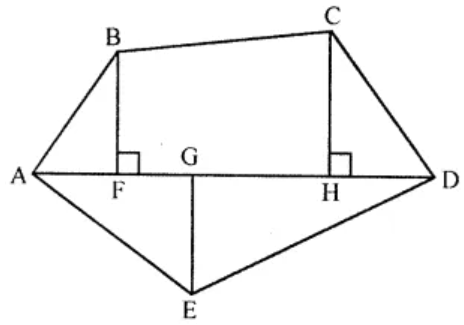Solution:

In the given figure, ABCDE, AD = 8 cm, AH = 6 cm, AG = 4 cm,

AF = 3cm ⊥ BF = 2 cm CH = 3 cm and ⊥ EG = 2.5 cm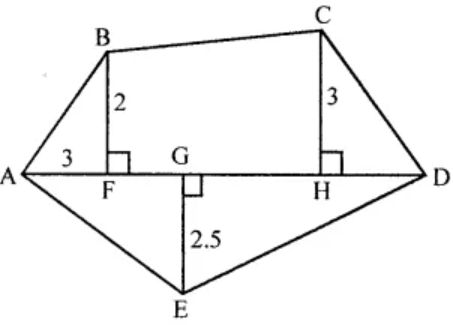The given figure, consists of 3 triangles and one trapezium.

Now,

Area of ∆AED = ½ × AD × GE

= ½ × 8 × 2.5

= 10 cm2

Area of ∆ABF = ½ × AF × BF

= ½ × 3 × 2

= 3 cm2

Area of ∆CDH = ½ × HD × CH

= ½ × (AD – AH) × 3

= ½ × (8 – 6) × 3

= ½ × 2 × 3

= 3 cm2

Area of trapezium BFHC = ½ × (BF + CH) × FH

= ½ × (2 + 3) × (AH – AF)

= ½ × 5 × (6 – 3)

= ½ × 5 × 3

= 7.5 cm2

Hence,

Total area of the figure = Area of ∆AED + Area of ∆ABF + Area of ∆CDH + Area of trapezium BFHC

= 10 + 3 + 3 + 7.5

= 23.5 cm2

17. Find the area of polygon PQRSTU shown in 1 the given figure, if PS = 11 cm, PY = 9 cm, PX = 8 cm, PW = 5 cm, PV = 3 cm, QV = 5 cm, UW = 4 cm, RX = 6 cm, TY = 2 cm.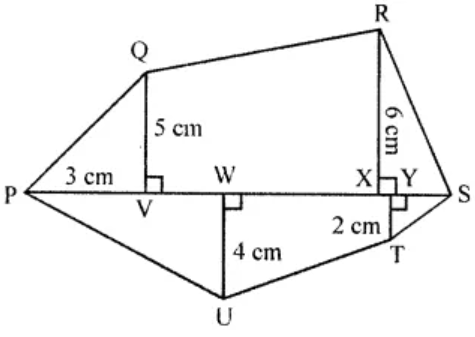Solution:

In the figure PQRSTU, we have

PS = 11 cm, PY = 9 cm, PX = 8 cm, PW = 5 cm, PV = 3 cm, QV = 5 cm, UW = 4 cm, RX = 6 cm and TY = 2 cm

And,

The figure consists of 4 triangle and 2 trapeziums

From the figure its seen that,

VX = PX – PV

= 8 – 3

= 5 cm

XS = PS – PX

= 11 – 8

= 3 cm

YS = PS – PY

= 11 – 9

= 2 cm

WY = PY – PW

= 9 – 5

= 4 cm

Now,

Area ∆PQV = ½ × PV × QV

= ½ × 3 × 5 = 15/2

= 7.5 cm2

Area of ∆RXS = ½ × XS × RX

= ½ × 3 × 6

= 9 cm2

Area of ∆PUW = ½ × PW × UW

= ½ × 5 × 4

= 10 cm2

Area ∆YTS = ½ × YS × TY

= ½ × 2 × 2

= 2 cm2

Area of trapezium ∆VX R = ½ × (QV + RX) × VX

= ½ × (5 + 6) × 5

= ½ × 11 × 5 cm2

= 55/7

= 27.5 cm2

Area of trapezium WUTY = ½ × (UW + TY) × WY

= ½ × (4 + 2) × 4

= ½ × 6 × 4

= 12 cm2

Hence,

Area of the figure = (7.5 + 9 + 10 + 2 + 27.5 + 12) cm2 = 68 cm2.

Exercise 18.3

1. The volume of a cube is 343 cm3, find the length of an edge of cube.
Solution:

Given,

Volume of a cube = 343 cm3

Let’s consider ‘a’ to be the edge of cube, then

V = a3 = 343 = (7)3

∴ a = 7 cm

2. Fill in the following blanks:

 Volume of cuboid Length Breadth Height (i) 90 cm3 – 5 cm 3 cm (ii) – 15 cm 8 cm 7 cm (iii) 62.5 m3 10 cm 5 cm –

Solution:

Volume of cuboid = length x Breadth x Height

(i) 90 cm3 = length x 5 cm x 3 cm

Length = 90/(5 x 3) = 90/15 = 6 cm

(ii) Volume = 15 cm x 8 cm x 7 cm

= 840 cm3

(iii) 62.5 m3 = 10 m x 5 m x height

Height = 62.5/(10 x 5) = 1.25 m

 Volume of cuboid Length Breadth Height (i) 90 cm3 6 cm 5 cm 3 cm (ii) 840 cm3 15 cm 8 cm 7 cm (iii) 62.5 m3 10 cm 5 cm 1.25 m

3. Find the height of a cuboid whose volume is 312 cm3 and base area is 26 cm2.
Solution:

Given,

Volume of a cuboid = 312 cm3

Base area = l × b = 26 cm2

∴ Height= Volume/Base area = 312/26 = 12cm

4. A godown is in the form of a cuboid of measures 55 m × 45 m × 30 m. How many cuboidal boxes can be stored in it if the volume of one box is 1.25 m3?
Solution:

Given,

Length of a godown (l) = 55 m

Height (h) = 30 m

So,

Volume = l × b × h

= (55 × 45 × 30) m3

= 74250 m3

Also given, volume of one box = 1.25 m3

Thus,

Number of boxes = 74250/1.25 = 59400 boxes

5. A rectangular pit 1.4 m long, 90 cm broad and 70 cm deep was dug and 1000 bricks of base 21 cm by 10.5 cm were made from the earth dug out. Find the height of each brick.
Solution:

Here l = 1.4 m = 140 cm, b = 90 cm and h = 70 cm

Volume of rectangular pit = l × b × h

= (140 × 90 × 70) cm3

= 882000 cm3

Volume of brick = 21 × 10.5 × h

Now,

Number of bricks = Volume of pit/ Volume of brick

1000 = 882000/ (21 × 10.5 × h)

h = 882000/ (21 × 10.5 × 1000)

= 4 cm

Thus, the height of each brick is 4 cm.

6. If each edge of a cube is tripled, then find how many times will its volume become?
Solution:

Let’s consider the edge of a cube to be x

Then, it’s volume = x3

Now, if the edge is tripled

Edge = 3x

So, volume = (3x)3 = 27x3

∴ Its volume is 27 times the volume of the given cube.

7. A milk tank is in the form of cylinder whose radius is 1.4 m and height is 8 m. Find the quantity of milk in litres that can be stored in the tank.
Solution:

Given,

Radius of the milk cylindrical tank = 1.4 m and height (h) = 8 m

Hence,

Volume of milk in the tank = πr2h

= (22/7) × 1.4 × 1.4 × 8 m3

= 49.28 m3

= 49.28 × 1000 litres

= 49280 litres

Therefore, the quantity of the tank is 49280 litres.

8. A closed box is made of 2 cm thick wood with external dimension 84 cm × 75 cm × 64 cm. Find the volume of the wood required to make the box.
Solution:

Given,

Thickness of the wood used in a closed box = 2 cm

External length of box (L) = 84 cm

Breadth (b) = 75 cm and height (h) = 64 cm

So, internal length (l) = 84 – (2 × 2)

= 84 – 4

= 80 cm

Breadth (b) = 75 – (2 × 2)

= 75 – 4

= 71 cm

and height (h) = 64 – (2 × 2)

= 64 – 4

= 60 cm

Hence, Volume of wood used = 84 × 75 × 64 – 80 × 71 × 60 cm3

= 403200 – 340800 cm3

= 62400 cm3

9. Two cylindrical jars contain the same amount of milk. If their diameters are in the ratio 3 : 4, find the ratio of their heights.
Solution:

Given,

Ratio in diameters of two cylindrical jars = 3 : 4

But their volumes are same.

Let’s assume h1 and h2 to be the heights of the two jars respectively.

Let radius of the first jar (r1) = 3x/2

and radius of the second jar (r2) = 4x/2

Then according to the condition in the problem, we have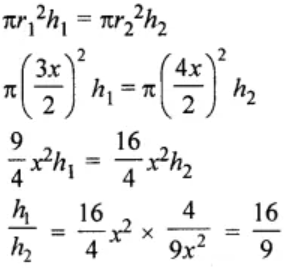Therefore, the ratio in their heights =16 : 9

10. The radius of the base of a right circular cylinder is halved and the height is doubled. What is the ratio of the volume of the new cylinder to that of the original cylinder?
Solution:

Let’s consider the radius of a cylinder to be r

And height = h

So,

Volume = πr2h

Now, its radius is halved and height is doubled, then

Volume = π(r/2)2 × (2h)

= πr2h/ 2

Thus, the ratio in the volumes of the new cylinder to old one is

= πr2h/ 2 : πr2h

= 1 : 2

11. A rectangular piece of tin of size 30 cm × 18 cm is rolled in two ways, once along its length (30 cm) and once along its breadth. Find the ratio of volumes of two cylinders so formed.
Solution:

Given,

Size of rectangular tin plate = 30 cm × 18 cm

(i) When rolled along its length (30 cm),

Then, the circumference of the circle so formed = 30 cm

Radius(r1) = C/2π = (30 x 7)/ (2 x 22) = 105/22 cm

And height (h1) = 18 cm

Then, volume = πr12h1 = π x (105/22)2 x (18) cm3

If it is rolled along its breadth (18 cm) then,

Circumference = 18 cm

So, radius (r2) = C/2π = (18 x 7)/ (2 x 22) = 63/22 cm

And height (h2) = 30 cm

Then, volume = πr22h2 = π x (63/22)2 x (30) cm3

Now, ratio between the two volumes

= π x (105/22)2 x (18) : π x (63/22)2 x (30)

= (105/22)2 x (18) : (63/22)2 x (30)

= 5 : 3

12. Water flows through a cylindrical pipe of internal diameter 7 cm at 5 m per sec. Calculate
(i) the volume in litres of water discharged by the pipe in one minute.
(ii) the time in minutes, the pipe would take to fill an empty rectangular tank of size 4 m × 3 m × 2.31 m.
Solution:

Given,

Speed of water flow through cylindrical pipe = 5 m/sec.

Internal diameter of the pipe = 7 cm

So, radius (r) = 7/2 cm

Now, length of water flow in 1 minutes (h) = 5 × 60 = 300 m

∴ Volume of water = πr2h

= 22/7 x 7/2 x 7/2 x 300 x 100 cm3

= 1155000 cm3 = 1155 litres

(i) the volume of water = 1155000 cm3

Volume of rectangular tank of size = 4m × 3m × 2.31m

= 27.72 m3

Also given, speed of water = 4 m/sec.

Radius of pipe = 7/2 cm

Volume of water in 1 sec = 22/7 x 7/2 x 7/2 x 5 x 100 cm3

= 19250 cm3

Hence,

(ii) Time taken to empty the tank = 27.72 m3/ 19250

= (2772 x 100 x 100 x 100)/(100 x 19250) sec

= 1440 sec

= 1440/60 = 24 minutes

13. Two cylindrical vessels are filled with milk. The radius of one vessel is 15 cm and height is 40 cm, and the radius of other vessel is 20 cm and height is 45 cm. Find the radius of another cylindrical vessel of height 30 cm which may just contain the milk which is in the two given vessels.
Solution:

Given,

Radius of one cylinder (r1) = 15 cm

And height (h1) = 40 cm

Radius of second cylinder (r2) = 20 cm

And height (h2) = 45 cm

Now,

Volume of first cylinder = πr12h1

= 22/7 x 15 x 15 x 40 cm3

= 198000/7 cm3

And,

Volume of second cylinder = πr22h2

= 22/7 x 20 x 20 x 45

= 396000/7 cm3

So, total volume = (198000/7 + 396000/7) cm3

= 594000/7 cm3

Now, volume of third cylinder = 594000/7 cm3

And height = 30 cm

Thus,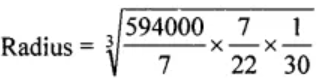= √900 = 30 cm

∴ Radius of the third cylinder = 30 cm

14. A wooden pole is 7 m high and 20 cm in diameter. Find its weight if the wood weighs 225 kg per m3.
Solution:

Given,

Height of pole (h) = 7 m

Diameter = 20 cm

So, radius (r) = 20/2 = 10 cm = 10/100 = 1/10 m

And,

Volume = πr2h

= 22/7 x 1/10 x 1/10 x 7 m3

= 22/100 m3

Weight of wood = 225 kg per m3

Hence,

Total weight = 225 × (22/100) = 99/2 = 49.5 kg

15. A cylinder of maximum volume is cut from a wooden cuboid of length 30 cm and cross-section a square of side 14 cm. Find the volume of the cylinder and the volume of the wood wasted.
Solution:

A cylinder of the maximum volume is cut from a wooden cuboid of length 30 cm and cross-section a square side 14 cm.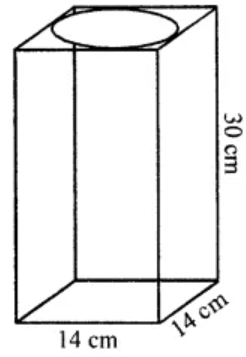So,

Diameter of the cylinder = 14 cm

⇒ Radius (r) = 14/2 = 7 cm

and height (h) = 30 cm

Volume of cuboid = 30 × 14 × 14 = 5880 cm3

Volume of cylinder = πr2h

= 22/7 × 7 × 7 × 30

= 4620 cm3

Hence,

The wastage of wood = 5880 – 4620 = 1260 cm3

Exercise 18.4

1. The surface area of a cube is 384 cm2. Find
(i) the length of an edge
(ii) volume of the cube.
Solution:

Given,

Surface area of a cube = 384 cm2

(i) Surface area of cube = 6(side)2

Hence, edge (side) = √(surface area/6)

= √(384/6)

= √64

= 8 cm

(ii) Volume = (Edge)3 = (8)= 8 × 8 × 8 cm3 = 512 cm3

2. Find the total surface area of a solid cylinder of radius 5 cm and height 10 cm. Leave your answer in terms of n.
Solution:

Given,

Radius of a solid cylinder (r) = 5 cm

Height (h) = 10 cm

Hence,

Total surface area = 2πrh + 2πr2

= 2rπ(h + r)

= 2π × 5(10 + 5)

= π × 10 × 15

= 150π cm2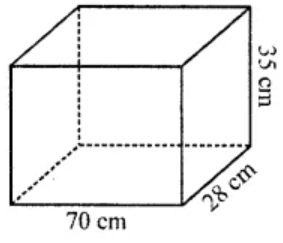3. An aquarium is in the form of a cuboid whose external measures are 70 cm × 28 cm × 35 cm. The base, side faces and back face are to be covered with coloured paper. Find the area of the paper needed.
Solution:

Given, a cuboid shaped aquarium

Length (l) = 70 cm

and height (h) = 35 cm

Now,

Area of base = 70 × 28 cm3

= 1960 cm3

Area of side face = (28 × 35) × 2 cm

= 1960 cm2

Area of back face = 70 × 35 cm2

= 2450 cm

Thus, the total area = 1960 + 1960 + 2450 = 6370 cm2

Hence, area of paper required is 6370 cm2.

4. The internal dimensions of rectangular hall are 15 m × 12 m × 4 m. There are 4 windows each of dimension 2 m × 1.5 m and 2 doors each of dimension 1.5 m × 2.5 m. Find the cost of white washing all four walls of the hall, if the cost of white washing is ₹5 per m2. What will be the cost of white washing if the ceiling of the hall is also white washed?
Solution:

Given,

Internal dimension of rectangular hall = 15m × 12 m × 4 m

Now,

Area of 4-walls = 2(l + b) × h

= 2(15 + 12) × 4

= 2 × 27 × 4 m2

= 216 m2

Area of 4 windows of size = (2 × 1.5) × 4 = 12 m2

Area of 2 door of size = 2 × (1.5 × 2.5) = 7.5 m2

So, area of remaining hall = 216 – (12 + 7.5) = 216 – 19.5 m2 = 196.5 m2

And,

Cost of white washing the walls all four halls of the house is at the rate of ₹5 per m2

= 196.5 × 5 = ₹982.50

Area of ceiling = l × b = 15 × 12 = 180 m2

Cost of white washing = 180 × 5 = ₹900

Therefore, the total cost for white washing = ₹982.50 + 900.00

= ₹1882.50

5. A swimming pool is 50 m in length, 30 m in breadth and 2.5 m in depth. Find the cost of cementing its floor and walls at the rate of ₹27 per square metre.
Solution:

Given,

Length of swimming pool = 50 m

Breadth of swimming pool = 30 m

Depth (Height) of swimming pool = 2·5 m

Now,

Area of floor = 50 × 30 = 1500 m2

Area of four walls = 2 (50 + 30) × 2.5 = 160 × 2.5 = 400 m2

So, the area to be cemented = 1500 m2 + 400 m2 = 1900 m2

Cost of cementing 1m2 = ₹27

Hence,

Cost of cementing 1900m= ₹27 × 1900 = ₹51300

6. The floor of a rectangular hall has a perimeter 236 m. Its height is 4·5 m. Find the cost of painting its four walls (doors and windows be ignored) at the rate of Rs. 8.40 per square metre.
Solution:

Given,

Perimeter of Hall = 236 m.

Height = 4.5 m

Perimeter = 2 (l + b) = 236 m

Area of four walls = 2 (l + b) × h

= 236 × 4.5

= 1062 m2

We have, cost of painting 1 m2 = ₹8.40

Hence,

Cost of painting 1062 m2 = ₹8.40 × 1062 = ₹8920.80

7. A cuboidal fish tank has a length of 30 cm, a breadth of 20 cm and a height of 20 cm. The tank is placed on a horizontal table and it is three-quarters full of water. Find the area of the tank which is in contact with water.
Solution:

Given,

Length of tank = 30 cm

Breadth of tank = 20 cm

Height of tank = 20 cm

As the tank is three-quarters full of water

So, the height of water in the tank = (20 x 3)/4 = 15 cm

Hence,

Area of the tank in contact with the water = Area of floor of Tank + Area of 4 walls upto 15 cm

= 30 × 20 + 2 (30 + 20) × 15

= 600 + 2 × 50 × 15

= 600 + 1500 = 2100 cm2

8. The volume of a cuboid is 448 cm3. Its height is 7 cm and the base is a square. Find
(i) a side of the square base
(ii) surface area of the cuboid.
Solution:

Given,

Volume of a cuboid = 448 cm3

Height = 7 cm

So, area of base = 448/7 = 64 cm2

Thus, the base is a square.

(i) Side of square base = √64 = 8 cm

(ii) Surface area of the cuboid = 2 [lb + bh + hl]

= 2[8 × 8 + 8 × 7 + 7 × 8] cm2

= 2[64 + 56 + 56]

= 2 × 176 = 352 cm2

9. The length, breadth and height of a rectangular solid are in the ratio 5 : 4 : 2. If its total surface area is 1216 cm2, find the volume of the solid.
Solution:

Given that the ratio in length, breadth and height of a rectangular solid = 5 : 4 : 2

Total surface area =1216 cm2

Let’s assume the length = 5x, breadth = 4x and height = 2x

Total surface area = 2[5x × 4x + 4x × 2x + 2x × 5x]

= 2[20x2 + 8x2 + 10x2 ]

= 2 × 38x2

= 76x2

So, 76x2 = 1216

⇒ x2 = 1216/76 = 16 = (4)2

∴ x = 4

Hence, the dimensions of the rectangular solid are

Length = 5 × 4 = 20 cm

Breadth = 4 × 4 = 16 cm

Height = 2 × 4 = 8 cm

and volume = lbh = 20 × 16 × 8 = 2560 cm3

10. A rectangular room is 6 m long, 5 m wide and 3.5 m high. It has 2 doors of size 1·1 m by 2 m and 3 windows of size 1.5 m by 1.4 m. Find the cost of whitewashing the walls and the ceiling of the room at the rate of ₹5.30 per square metre.
Solution:

Given, Length of room = 6 m

Breadth of room = 5 m

Height of room = 3.5 m

So, Area of four walls = 2 (l + b) × h

= 2 (6 + 5) × 3.5

= 77 m2

Area of 2 doors and 3 windows = (2 × 1.1 × 2 + 3 × 1.5 × 1.4)

= (44 + 6.3) m2

= 10.7 m2

Area of ceiling = l × b = 6 × 5 = 30 m2

Thus,

Total area for white washing = (77 – 10.7 + 30) m2 = 96.3 m2

Hence, the cost of white washing = ₹(96.3 × 5.30) = ₹510.39

11. A cuboidal block of metal has dimensions 36 cm by 32 cm by 0·25 m. It is melted and recast into cubes with an edge of 4 cm.
(i) How many such cubes can be made?
(ii) What is the cost of silver coating the surfaces of the cubes at the rate of ₹0·75 per square centimetre?
Solution:

(i) Given, Length of cuboid = 36 cm

Breadth of cuboid = 32 cm

Height of cuboid = 0·25 × 100 = 25 cm

So,

Volume of cuboid = lbh

= (36 × 32 × 25) cm3

= 28800 cm3

Volume of cube = (side)

= (4)2

= 64 cm2

Hence, the number of cubes recasting from cuboid = 28800/64 = 450

(ii) Surface area of 1 cube = 6 × a

= 6 × 16

= 96 cm2

So, the surface area of 450 cubes = 96 × 450

= 43200 cm2

Hence, the cost of silver coating on cubes = ₹0.75 × 43200

= ₹32400

12. Three cubes of silver with edges 3 cm, 4 cm and 5 cm are melted and recast into a single cube, find the cost of coating the surface of the new cube with gold at the rate of ₹3.50 per square centimetre?
Solution:

Let’s consider a cm to be the edge of new cube

Then, according to given conditions in the question

a3 = 33 + 43 + 53

= 27 + 64 + 125

= 216 cm3

a =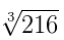∴ a = 6 cm

So, the surface area of new cube = 6 × (side)

= 6 × (6)2

= 216 cm2

Hence, the cost of coating the surface of new cube = ₹3.50 × 216 = ₹156

13. The curved surface area of a hollow cylinder is 4375 cm2, it is cut along its height and formed a rectangular sheet of width 35 cm. Find the perimeter of the rectangular sheet.
Solution:

Given, curved surface area of a hollow cylinder = 4375 cm2

By cutting it from the height,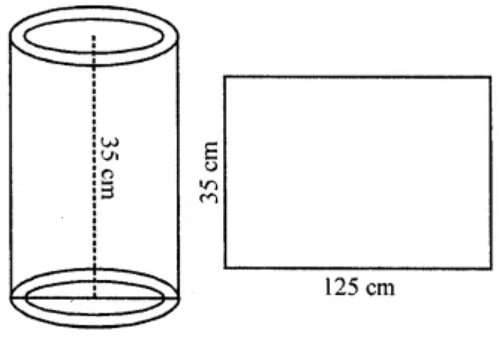It becomes a rectangular sheet whose width = 35 cm

So, the height of cylinder = 35 cm

And, length of sheet = Area/Height

= 4375/35

= 125 cm

Hence, Perimeter of the sheet = 2(l + b)

= 2 × (125 + 35)

= 2 × 160

= 320 cm

14. A road roller has a diameter 0.7 m and its width is 1.2 m. Find the least number of revolutions that the roller must take in order to level a playground of size 120 m × 44 m.
Solution:

Given,

Diameter of a road roller = 0.7 m = 70 cm

So, radius (r) = 70/2 cm = 35 cm = 35/100 m

and width (h) = 1.2 m

Now,

Curved surface area = 2πrh

= (2 × 22/7 × 35/100 × 1.2) m2

= 264/100 m2

Area of playground = 120 m × 44 m

= 120 × 44 m2

= 5280 m2

Hence, the number of revolution made by the road roller = (5280/264) × 100

= 2000 revolutions

15. A company packages its milk powder in cylindrical container whose base has a diameter of 14 cm and height 20 cm. Company places a label around the surface of the container (as shown in the figure). If the label is placed 2 cm from top and bottom, what is the area of the label?

Solution:

Given,

Diameter of cylindrical container = 14 cm

So, radius (r) = 14/2 = 7 cm

And, height (h) = 20 cm

Now,

Width of label = 2 0 – (2 + 2) cm = 20 – 4 = 16 cm

Hence, area of label = 2πrh

= 2 × (22/7) × 7 × 16

= 704 cm2

16. The sum of the radius and height of a cylinder is 37 cm and the total surface area of the cylinder is 1628 cm2. Find the height and the volume of the cylinder.
Solution:

Given,

Sum of height and radius of a cylinder = 37 cm

Total surface area = 1628 cm2

Let’s consider the radius to be r

Then, height = (37 – r) cm

Now,

Total surface area = 2π(h + r)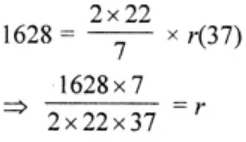⇒ r = 7 cm

Height = 37 – 7 = 30 cm

Hence,

Volume = πr2h

= (22/7) × 7 × 7 × 30 cm

= 4620 cm3

17. The ratio between the curved surface and total surface of a cylinder is 1 : 2. Find the volume of the cylinder, given that its total surface area is 616 cm3.
Solution:

Given that ratio between curved surface and total surface area of a cylinder = 1 : 2

Total surface area = 616 cm2

So, curved surface area = 616/2 = 308 cm2

And,

Area of two circular faces = 616 – 308 = 308 cm2

Area of one circular face = 308/2 = 154 cm2

Now, let’s consider the radius to be r

πr2 = 154

(22/7) × r2 = 154

r2 = (154 × 7)/ 22 = 49

⇒ r = 49 = √7 cm

Hence, the volume = πr2h = (22/7) × 7 × 7 × 7 = 1078 cm2

18. The given figure shown a metal pipe 77 cm long. The inner diameter of cross section is 4 cm and the outer one is 4.4 cm.
Find its
(i) inner curved surface area
(ii) outer curved surface area
(iii) total surface area.
Solution: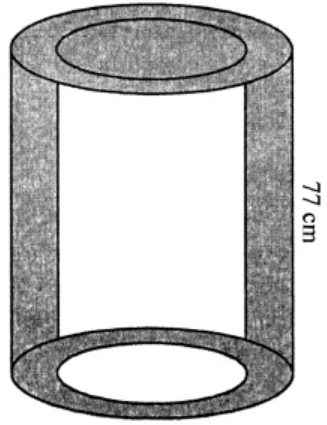Given,

Length of metal pipe (h) = 77 cm

Inner diameter = 4 cm

and outer diameter = 4.4 cm

So, inner radius (r) = 4/2 = 2 cm

And outer radius (R) = 4.4/2 = 2.2 cm

(i) Inner curved surface area = 2πrh

= 2 × 22/7 × 2 × 77 cm2

= 968 cm2

(ii) Outer surface area = 2πRH

= 2 × 22/7 × 2.2 × 77 cm2

= 1064.8 cm2

(iii) Surface area of upper and lower rings = 2[πR2 – πr2]

= 2 × 22/7 (2.22 – 22) cm2

= 44/7 × 4.2 × 0.2

= 5.28 cm2

Hence,

Total surface area = (968 + 1064.8 + 5.28) cm2 = 2038.08 cm2

1. A square field of side 65 m and rectangular field of length 75 m have the same perimeter. Which field has a larger area and by how much?
Solution:

Given,

Side of a square field = 65 m

So, perimeter = 4 × Side

= 4 × 65

= 260 m

Now, perimeter of a rectangular field = 260 m

And given, length (l) = 75 m

Perimeter of rectangle = 2(l + b)

⇒ 260 = 2(75 + b)

260 = 150 + 2b

2b = 260 – 150

b = 110/2 = 55 m

Then,

Area of square field = (side)2

= (65)2 m

= 4225 m2

And, area of rectangular field = l × b

= 75 × 55 m

= 4125 m2

Hence, it is clear that area of square field is greater.

Difference = 4225 – 4125

= 100 m2

2. The shape of a top surface of table is a trapezium. Find the area if its parallel sides are 1.5 m and 2.5 m and perpendicular distance between them is 0.8 m.
Solution: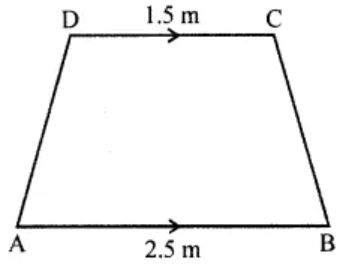Shape of the top of a table is trapezium and parallel sides are 1.5 m and 2.5 m and the perpendicular distance between them = 0.8m

Hence,

Area = ½ × (Sum of parallel sides) × height

= ½ × (1.5 + 2.5) × 0.8

= ½ × 4 × 0.8 m2

= 1.6 m2

3. The length and breadth of a hall of a school are 26 m and 22 m respectively. If one student requires 1.1 sq. m area, then find the maximum number of students to be seated in this hall.
Solution:

Given, length of a school hall (l) = 26 m and breadth (b) = 22 m

So, area = l × b

= 26 × 22 m2

= 572 m2

One student requires 1.1 sq. m area

Hence, number of students = 572/1.1

= (572 × 10)/11

= 520 students

4. It costs ₹936 to fence a square field at ₹7.80 per metre. Find the cost of levelling the field at ₹2.50 per square metre.
Solution:

Given,

Cost of fencing the square field at ₹7.80 per metre = ₹936.

So, total fence required will be = 936/7.80 = 120

Thus, the perimeter of the field = 120 m

⇒ 4 × Side = 120 m [Since, it’s a square field]

⇒ Side = 120/4

∴ Side = 30 m

Hence, Area of square field = (30)2 = 900 m2

= 900 × 2.50 = ₹2250

5. Find the area of the shaded portion in the following figures all measurements are given in cm.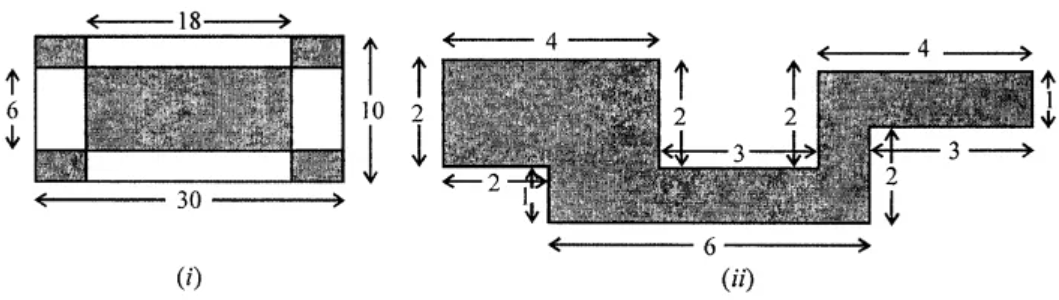Solution:

(i) Outer length = 30 cm

Side of each rectangle of the corner (l) = (30 – 18)/ 2 = 6 cm

and b = (10 – 6)/2 = 4/2 = 2 cm

So,

Area of 4 comer = (6 × 2) × 4

= 48 cm2

And area of inner rectangle = 18 × 6

= 108 cm2

Therefore,

Area of shaded portion = 108 + 48 = 156cm2

(ii) Area of rectangle I = 4 × 2 = 8 cm2

Area of rectangle II = 4 × 1 = 4 cm2

Area of rectangle III = 6 × l = 6 cm2

and area of square IV = 1 × 1 = 1 Cm2

∴ Total area of shaded portion = 8 + 4 + 6 + 1 = 19 cm2

6. Area of a trapezium is 160 sq. cm. Lengths of parallel sides are in the ratio 1:3. If smaller of the parallel sides is 10 cm in length, then find the perpendicular distance between them.
Solution:

Given,

Area of trapezium = 160 cm2

Ratio of the length of its parallel sides = 1 : 3

Smaller parallel side = 10 cm

Then,

Length of greater side = 10 × 3 = 30 cm

Now, distance between them = h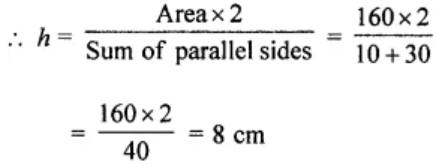7. The area of a trapezium is 729 cm2 and the distance between two parallel sides is 18 cm. If one of its parallel sides is 3 cm shorter than the other parallel side, find the lengths of its parallel sides.
Solution:

Given,

Area of a trapezium = 729 cm2

Distance between two parallel sides (Altitude) = 18 cm

So, the sum of parallel sides = (Area × 2)/ Altitude

= (729 × 2)/ 18

= 81 cm

One parallel side is shorter than the second by 3 cm

Let the longer side be taken as x

Then, shorter side = x – 3

According to the question, we have

⇒ x + x – 3 = 81

2x = 81 + 3 = 84

x = 84/2 = 42

Hence, the longer side = 42 cm and shorter side = 42 – 3 = 39 cm

8. Find the area of the polygon given in the figure: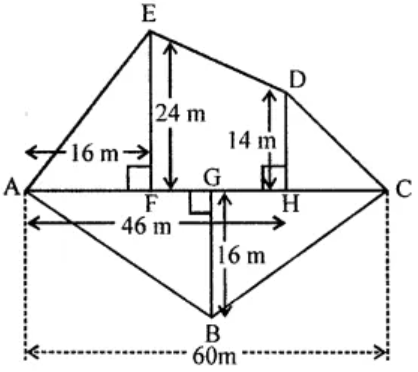Solution:

In the given figure,

AC = 60 m, AH = 46 m, AF = 16 m, EF = 24 m, DH = 14 m, BG = 16 m

∴ FH = AH – AF = 46 – 16 = 30

And, HC = AC – AH = 60 – 46 = 14

In the figure, there are 3 triangles and one trapezium.

Now,

Area of ∆ABC = ½ AC × BG

= ½ × 60 × 16

= 480 m2

Area of ∆AEF = ½ AF × EF

= ½ × 16 × 24

= 192 m2

Area of ∆DHC = ½ HC × DH

= ½ × 14 × 14

= 98 m2

Area of trapezium EFHD = ½ (EF + DH) × FH

= ½ (24 + 14) × 30

= ½ × 38 × 30

= 570 m2

Therefore, total area of the figure = Area of ∆ABC + area ∆AEF + area ∆DHC + area trapezium EFHD

= 480 + 192 + 98 + 570

= 1340 m2

9. The diagonals of a rhombus are 16 m and 12 m, find:
(i) its area
(ii) length of a side
(iii) perimeter.
Solution:

Diagonals of a rhombus are d1 = 16 cm and d2 = 12 cm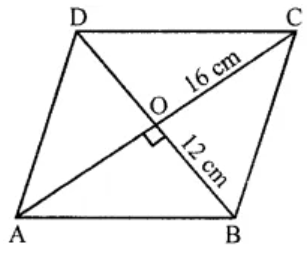(i) Area = (d1 × d2) / 2

= (16 × 12)/ 2

= 96 cm2

(ii) As the diagonals of rhombus bisect each other at right angles, we have

AO = OC and BO = OD

AO = 16/2 = 8 cm and BO = 12/2 = 6 cm

Now, in right ∆AOB

AB2 = AO2 + BO2  [By Pythagoras Theorem]

= 82 + 62

= 64 + 36

= 100 = (10)2

∴ AB = 10 cm

Therefore, the side of rhombus = 10 cm

10. The area of a parallelogram is 98 cm2. If one altitude is half the corresponding base, determine the base and the altitude of the parallelogram.
Solution:

Given,

Area of a parallelogram = 98 cm2

One altitude = Half of its corresponding base

Let’s consider the base as x cm

Then altitude = x/2 cm

So, area = Base × Altitude

⇒ 98 = x × (x/2

x2 = 98 × 2

= 196

= (14)2

∴ x = 14

Therefore, Base = 14 cm and altitude = = 7 cm

11. Preeti is painting the walls and ceiling of a hall whose dimensions are 18 m × 15 m × 5 m. From each can of paint 120 m2 of area is painted. How many cans of paint does she need to paint the hall?
Solution:

Given,

Length of a hall (l) = 18 m

height (h) = 5 m

So,

Area of 4-wall and ceiling = 2(l + b)h + lb

= 2(18 + 15) × 5 + 18 × 15 m2

= 2 × 33 × 5 + 270

= 330 + 270

= 600 m2

From 1 can an area of 120 m2 can be painted

Hence, total number of cans required to paint the area of 600 m2 = 600/120 = 5 cans

12. A rectangular paper is size 22 cm × 14 cm is rolled to form a cylinder of height 14 cm, find the volume of the cylinder. (Take π = 22/7)
Solution:

Given,

Length of a rectangular paper = 22 cm and breadth = 14 cm

By rolling it a cylinder is formed whose height is 14 cm

And, circumference of the base = 22 cm

We know that, circumference = 2πr

So, radius (r) = C/2π = (22 × 7)/(2 × 22) = 7/2 cm

Hence,

Volume of the cylinder so formed = πr2h

= 22/7 × 7/2 × 7/2 × 14

= 539cm3

13. A closed rectangular wooden box has inner dimensions 90 cm by 80 cm by 70 cm. Compute its capacity and the area of the tin foil needed to line its inner surface.
Solution:

Given,

Inner length of rectangular box = 90 cm

Inner breadth of rectangular box = 80 cm

Inner height of rectangular box = 70 cm

Now,

Capacity of rectangular box = Volume of rectangular box

= l × b × h

= 90 cm × 80 cm × 70 cm

= 504000 cm3

And,

Required area of tin foil = 2 (lb + bh + lh)

= 2(90 × 80 + 80 × 70 + 90 × 70) cm2

= 2(7200 + 5600 + 6300) cm2

= 2 × 19100 cm2

= 38200 cm2

14. The lateral surface area of a cuboid is 224 cm2. Its height is 7 cm and the base is a square. Find
(i) side of the square base
(ii) the volume of the cuboid.
Solution:

Given,

Lateral surface area of a cuboid is 224 cm2

Height (h) = 7 cm

(i) 2(l + b) × h = 224

⇒ 2(l + b) × 7 = 224

l + b = 224/14 = 16 cm

But l = b [Since, the base of cuboid is a square]

So, 2 × side = 16 cm

⇒ Side = 16/2 = 8 cm

(ii) Volume of cuboid = lbh = 8 × 8 × 7 cm3 = 448 cm3

15. The inner dimensions of a closed wooden box are 2 m by 1.2 m by 0.75 m. The thickness of the wood is 2.5 cm. Find the cost of wood required to make the box if 1 m3 of wood costs ₹5400.
Solution:

Given,

Inner dimensions of wooden box are 2 m, 1.2 m, 0.75 m

Thickness of the wood = 2.5 cm = 2.5/100 m = 0.025 m

Now,

External dimensions of wooden box are

= (2 + 2 × 0.025), (1.2 + 2 × 0.025), (0.75 + 2 × 0.025)

= (2 + 0.05), (1.2 + 0.05), (0.75 + 0.5)

= 2.05, 1.25, 0.80

Thus,

Volume of solid = External volume of box – Internal volume of box

= 2.05 × 1.25 × 0.80 m3 – 2 × 1.2 × 0.75m3

= 2.05 – 1.80 = 0.25 m3

Given, the cost of wood = ₹5400 for 1 m3

Hence,

Total cost = ₹5400 × 0.25 = ₹5400 × 25/100

= ₹54 × 25

= ₹1350

16. A car has a petrol tank 40 cm long, 28 cm wide and 25 cm deep. If the fuel consumption of the car averages 13.5 km per litre, how far can the car travel with a full tank of petrol?
Solution:

Given,

Capacity of car tank = 40 cm × 28 cm × 25 cm = (40 × 28 × 25) cm3

= (40 × 28 × 25)/1000 litre [∵ 1000 cm3 = 1 litre]

Average fuel consumption of car = 13.5 km per litres

Then, the distance travelled by car is given by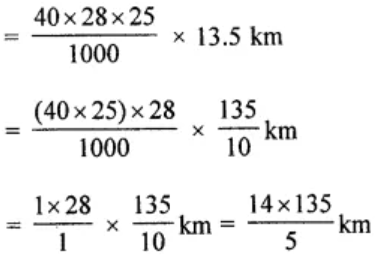= 14 × 27 km

= 378 km

Hence, the car can travel 378 km with a full tank of petrol.

17. The diameter of a garden roller is 1.4 m and it is 2 m long. How much area it will cover in 5 revolutions?
Solution:

Given,

Diameter of a garden roller = 1.4 m

So, its radius (r) = 1.4/2 = 0.7 m = 70 cm

and length (h) = 2m

Now, Curved surface area = 2πrh

= 2 × 22/7 × 70 × 200 cm2

= 88000 cm2

Hence, area covered in 5 revolutions = (88000 × 5)/10000 m2 = 44 m2

18. The capacity of an open cylindrical tank is 2079 m3 and the diameter of its base is 21m. Find the cost of plastering its inner surface at ₹40 per square metre.
Solution:

Given, capacity of an open cylindrical tank = 2079 m3

Diameter of base = 21 m

So, radius (r) = 21/2 m

Let h be the height, then we have

πr2h = 2079

22/7 × 21/2 × 21/2 × h = 2079

h = (2079 × 2)/ (11 × 3 × 21) = 6 m

Now,

Curved Surface Area + Base area = 2πrh + πr2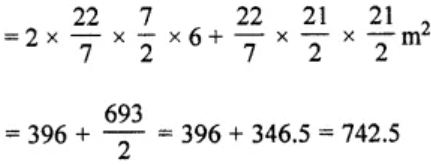Hence, the cost of plastering the surface = ₹40 × 742.5 = ₹29700

19. A solid right circular cylinder of height 1.21 m and diameter 28 cm is melted and recast into 7 equal solid cubes. Find the edge of each cube.
Solution:

Given,

Height of solid right circular cylinder = 1.21 m = 121 cm

and diameter = 28 cm

So, radius (r) = 28/2 = 14 cm

Now,

Volume of the metal used = πr2h

= 22/7 × 14 × 14 × 121 cm3

= 74536 cm3

Thus, the volume of 7 solid cubes = 74536 cm3

And, volume of 1 cube = 74536/7 = 10648 cm3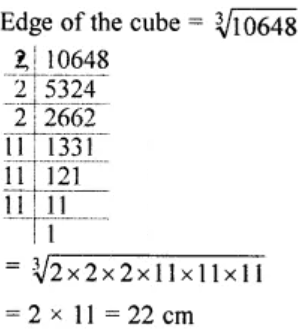Hence, the edge of each cube is 22 cm.

20. (i) How many cubic metres of soil must be dug out to make a well 20 m deep and 2 m in diameter?
(ii) If the inner curved surface of the well in part (i) above is to be plastered at the rate of ₹50 per m2, find the cost of plastering.
Solution:

(i) Given,

Depth of a well (h) = 20 m

and diameter = 2 m

Radius (r) = 2/2 = 1 m

Volume of earth dug out = πr2h

= 22/7 × 1 × 1 × 20

= 440/7 m3

(ii) Inner curved surface area = 2πrh

= 2 × 22/7 × 1 × 20

= 880/7 m2

The cost of plastering at the rate of ₹50 per m2 = ₹ 880/7 × 50

= ₹ 44000/7

= ₹ 6285.70